# US20100328500A1 - Zoom lens system, imaging device and camera - Google Patents

## Info

Publication number
US20100328500A1
US20100328500A1 US12/864,900 US86490009A US2010328500A1 US 20100328500 A1 US20100328500 A1 US 20100328500A1 US 86490009 A US86490009 A US 86490009A US 2010328500 A1 US2010328500 A1 US 2010328500A1
Authority
US
United States
Prior art keywords
lens
lens unit
image
zoom
zoom lens
Prior art date
Legal status (The legal status is an assumption and is not a legal conclusion. Google has not performed a legal analysis and makes no representation as to the accuracy of the status listed.)
Granted
Application number
US12/864,900
Other versions
US8300318B2 (en
Inventor
Keiki Yoshitsugu
Takakazu Bito
Current Assignee (The listed assignees may be inaccurate. Google has not performed a legal analysis and makes no representation or warranty as to the accuracy of the list.)
Panasonic Corp
Original Assignee
Panasonic Corp
Priority date (The priority date is an assumption and is not a legal conclusion. Google has not performed a legal analysis and makes no representation as to the accuracy of the date listed.)
Filing date
Publication date
Priority to JP2008015987 priority Critical
Priority to JP2008-015987 priority
Priority to JP2008-315079 priority
Priority to JP2008315079 priority
Application filed by Panasonic Corp filed Critical Panasonic Corp
Priority to PCT/JP2009/000198 priority patent/WO2009096156A1/en
Assigned to PANASONIC CORPORATION reassignment PANASONIC CORPORATION ASSIGNMENT OF ASSIGNORS INTEREST (SEE DOCUMENT FOR DETAILS). Assignors: YOSHITSUGU, KEIKI, BITO, TAKAKAZU
Publication of US20100328500A1 publication Critical patent/US20100328500A1/en
Application granted granted Critical
Publication of US8300318B2 publication Critical patent/US8300318B2/en
Active legal-status Critical Current

• 238000003384 imaging method Methods 0.000 title claims description 28
• 230000003287 optical Effects 0.000 claims abstract description 159
• 230000005499 meniscus Effects 0.000 claims description 95
• 230000004075 alteration Effects 0.000 description 102
• 238000010586 diagram Methods 0.000 description 93
• 230000000694 effects Effects 0.000 description 29
• 230000000875 corresponding Effects 0.000 description 22
• 239000004568 cement Substances 0.000 description 17
• 235000020127 ayran Nutrition 0.000 description 13
• 238000010276 construction Methods 0.000 description 9
• 230000015556 catabolic process Effects 0.000 description 6
• 230000004059 degradation Effects 0.000 description 6
• 238000006731 degradation reaction Methods 0.000 description 6
• 206010010071 Coma Diseases 0.000 description 4
• 201000009310 astigmatism Diseases 0.000 description 4
• 230000004308 accommodation Effects 0.000 description 3
• 239000004973 liquid crystal related substance Substances 0.000 description 3
• 239000007787 solid Substances 0.000 description 3
• 238000005549 size reduction Methods 0.000 description 2
• 210000001747 Pupil Anatomy 0.000 description 1
• 238000005452 bending Methods 0.000 description 1
• 230000000295 complement Effects 0.000 description 1
• 230000003247 decreasing Effects 0.000 description 1
• 230000000593 degrading Effects 0.000 description 1
• 229910044991 metal oxide Inorganic materials 0.000 description 1
• 150000004706 metal oxides Chemical class 0.000 description 1
• 239000004065 semiconductor Substances 0.000 description 1
• 230000035945 sensitivity Effects 0.000 description 1
• 230000001629 suppression Effects 0.000 description 1

## Images

•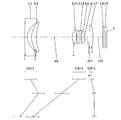••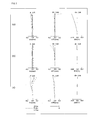•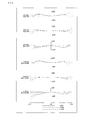•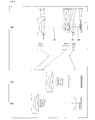•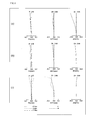•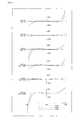•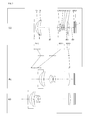•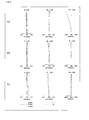•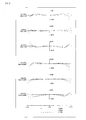•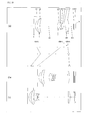••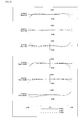•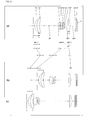•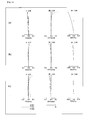•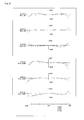•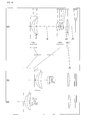•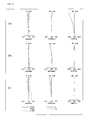•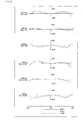•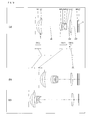•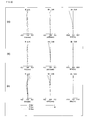•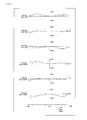•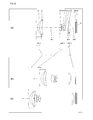•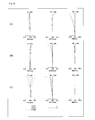•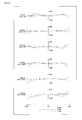•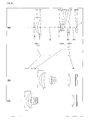•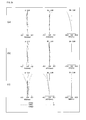•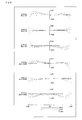•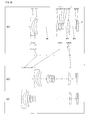•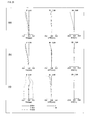•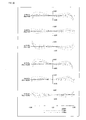•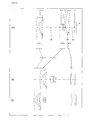•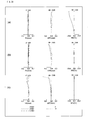•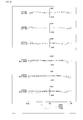•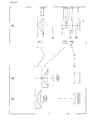•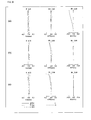•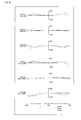•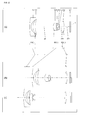•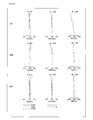•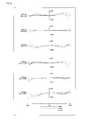•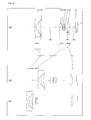•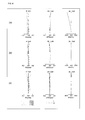••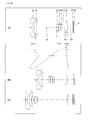•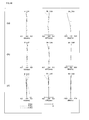•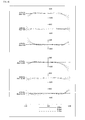•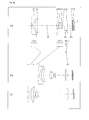•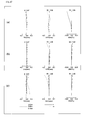•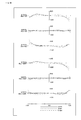•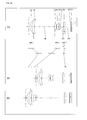•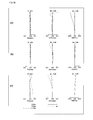•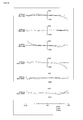•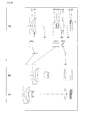•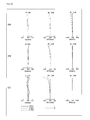•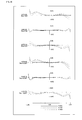•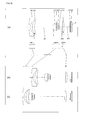•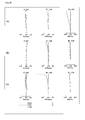•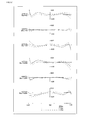•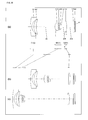•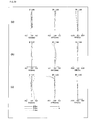•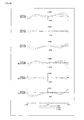•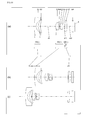•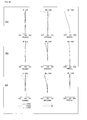•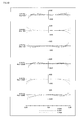••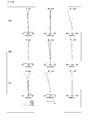•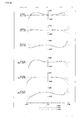•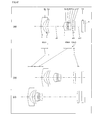•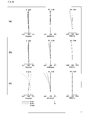•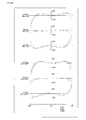•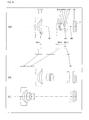•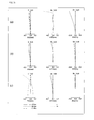•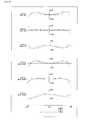•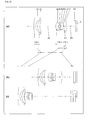•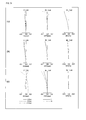•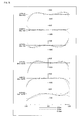•## Classifications

• GPHYSICS
• G02OPTICS
• G02BOPTICAL ELEMENTS, SYSTEMS, OR APPARATUS
• G02B27/00Optical systems or apparatus not provided for by any of the groups G02B1/00 - G02B26/00, G02B30/00
• G02B27/64Imaging systems using optical elements for stabilisation of the lateral and angular position of the image
• G02B27/646Imaging systems using optical elements for stabilisation of the lateral and angular position of the image compensating for small deviations, e.g. due to vibration or shake
• GPHYSICS
• G02OPTICS
• G02BOPTICAL ELEMENTS, SYSTEMS, OR APPARATUS
• G02B15/00Optical objectives with means for varying the magnification
• G02B15/14Optical objectives with means for varying the magnification by axial movement of one or more lenses or groups of lenses relative to the image plane for continuously varying the equivalent focal length of the objective
• G02B15/143Optical objectives with means for varying the magnification by axial movement of one or more lenses or groups of lenses relative to the image plane for continuously varying the equivalent focal length of the objective having three groups only
• G02B15/1435Optical objectives with means for varying the magnification by axial movement of one or more lenses or groups of lenses relative to the image plane for continuously varying the equivalent focal length of the objective having three groups only the first group being negative
• G02B15/143507Optical objectives with means for varying the magnification by axial movement of one or more lenses or groups of lenses relative to the image plane for continuously varying the equivalent focal length of the objective having three groups only the first group being negative arranged -++

## Abstract

A zoom lens system of the present invention has a plurality of lens units each composed of at least one lens element and, in order from the object side to the image side, comprises: a first lens unit having negative optical power and composed of two lens elements; a second lens unit having positive optical power; and a third lens unit having positive optical power, wherein in zooming from a wide-angle limit to a telephoto limit during image taking, the individual lens units are moved along an optical axis such that an interval between the first lens unit and the second lens unit should decrease and that an interval between the second lens unit and the third lens unit should increase, so that magnification change is achieved, and wherein on the image side relative to the second lens unit, an aperture diaphragm is arranged that moves along the optical axis integrally with the second lens unit during zooming.

## Description

TECHNICAL FIELD
• The present invention relates to a zoom lens system, an imaging device and a camera. In particular, the present invention relates to: a zoom lens system that has not only a high resolution but also a reduced overall optical length (overall length of lens system) and a variable magnification ratio as high as approximately 5 and that has a view angle of approximately 70° at a wide-angle limit and hence is satisfactorily adaptable for wide-angle image taking; an imaging device employing this zoom lens system; and a thin and remarkably compact camera employing this imaging device.
• BACKGROUND ART
• With recent progress in the development of solid-state image sensors such as a CCD (Charge Coupled Device) and a CMOS (Complementary Metal-Oxide Semiconductor) having a high pixel, digital still cameras and digital video cameras (simply referred to as “digital cameras”, hereinafter) are rapidly spreading that employ an imaging device including an imaging optical system of high optical performance corresponding to the above-mentioned solid-state image sensors of a high pixel. Among these digital cameras of high optical performance, demands are increasing especially for digital cameras of compact type.
• In digital cameras of compact type described above, from the perspective of easiness in carrying and accommodation, further thickness reduction is required. For the purpose of realizing such compact and thin digital cameras, in the conventional art, variable zoom lens systems have been proposed that have a three-unit construction of negative lead type, in order from the object side to the image side, comprising a first lens unit having negative optical power, a second lens unit having positive optical power and a third lens unit having positive optical power and that have a reduced overall optical length (overall length of lens system: the distance measured from the vertex of a lens surface on the most object side in the entire lens system to the image surface).
• For example, Japanese Patent Publication No. 3513369 discloses a zoom lens which, in order from the object side to the image side, comprises three lens units of negative, positive and positive and in which: at a telephoto limit in comparison with a wide-angle limit, the individual lens units are moved such that the interval between first and second lens units and the interval between second and third lens units should decrease so that magnification change is achieved; the first lens unit is composed of two lenses of negative and positive; the second lens unit is composed of independent two lenses of positive and negative; the third lens unit is composed of one positive lens; and a particular relation is satisfied by the radius of curvature of the object side surface of the negative lens contained in the second lens unit and the focal length of the entire system at a wide-angle limit. In this zoom lens disclosed in Japanese Patent Publication No. 3513369, overall optical length is reduced, and still high optical performance is obtained over the entire variable magnification range.
• Further, Japanese Laid-Open Patent Publication No. 2006-301154 discloses a zoom lens which, in order from the object side to the image side, comprises three lens units of negative, positive and positive and in which: the intervals between the individual lens units vary at the time of magnification change; particular relations are satisfied respectively by the taken-image height and the focal length of the entire system at a wide-angle limit, by the axial interval between the first and the second lens units and the focal length of the first lens unit, and by the axial interval between the first and the second lens units and the focal length of the second lens unit; and a variable magnification ratio that falls within a particular range is obtained. This zoom lens disclosed in Japanese Laid-Open Patent Publication No. 2006-301154 has a wide view angle at a wide-angle limit as well as a relatively high variable magnification ratio.
• Moreover, Japanese Laid-Open Patent Publication No. 2006-065034 discloses a zoom lens which, in order from the object side to the image side, comprises three lens units of negative, positive and positive and in which: the intervals between the individual lens units vary at the time of magnification change; the first lens unit is composed of two lenses of negative and positive; the second lens unit is constructed from a 2a-th lens unit composed of two lenses of positive and negative and a 2b-th lens unit composed of at least one positive lens arranged on the image side relative to the 2a-th lens unit; the third lens unit is composed of at least one positive lens; and particular relations are satisfied by the imaging magnifications of the second lens unit at a wide-angle limit and a telephoto limit, the interval between the first and the second lens units at a wide-angle limit, and the interval between the second and the third lens units at a telephoto limit. This zoom lens disclosed in Japanese Laid-Open Patent Publication No. 2006-065034 achieves desired optical performance and still has a reduced number of component lenses and relative compactness.
• Patent Document 1: Japanese Patent Publication No. 3513369 Patent Document 2: Japanese Laid-Open Patent Publication No. 2006-301154 Patent Document 3: Japanese Laid-Open Patent Publication No. 2006-065034 DISCLOSURE OF THE INVENTION Problems to be Solved by the Invention
• The above-mentioned zoom lens disclosed in Japanese Patent Publication No. 3513369 has high optical performance, a view angle as wide as 65° to 75° at a wide-angle limit, and a reduced overall optical length. This permits further thickness reduction in digital cameras of compact type. Nevertheless, the zoom lens has as small a variable magnification ratio as approximately 3, and hence does not satisfy a requirement in digital cameras of compact type in recent years.
• Further, the zoom lens disclosed in Japanese Laid-Open Patent Publication No. 2006-301154 has a sufficient view angle for wide-angle image taking and a higher variable magnification ratio than the zoom lens disclosed in Japanese Patent Publication No. 3513369. Nevertheless, in this lens configuration, the amount of movement of the second lens unit along the optical axis at the time of magnification change is large. Thus, the overall optical length increases, and hence further thickness reduction cannot be achieved in digital cameras of compact type.
• Moreover, similarly to the zoom lens disclosed in Japanese Patent Publication No. 3513369, the zoom lens disclosed in Japanese Laid-Open Patent Publication No. 2006-065034 achieves desired optical performance and still has a sufficient view angle for wide-angle image taking and a reduced overall optical length. This permits further thickness reduction in digital cameras of compact type. Nevertheless, this zoom lens has as small a variable magnification ratio as approximately 3, and hence does not satisfy a requirement in digital cameras of compact type in recent years.
• An object of the present invention is to provide: a zoom lens system that has not only a high resolution but also a reduced overall optical length and a variable magnification ratio as high as approximately 5 and that has a view angle of approximately 70° at a wide-angle limit and hence is satisfactorily adaptable for wide-angle image taking; an imaging device employing this zoom lens system; and a thin and remarkably compact camera employing this imaging device.
• Solution to the Problems
• One of the above-mentioned objects is achieved by the following zoom lens system. That is, the present invention relates to
• a zoom lens system having a plurality of lens units each composed of at least one lens element and,
• in order from an object side to an image side, comprising:
• a first lens unit having negative optical power and composed of two lens elements;
• a second lens unit having positive optical power; and
• a third lens unit having positive optical power, wherein
• in zooming from a wide-angle limit to a telephoto limit during image taking, the individual lens units are moved along an optical axis such that an interval between the first lens unit and the second lens unit should decrease and that an interval between the second lens unit and the third lens unit should increase, so that magnification change is achieved, and wherein on the image side relative to the second lens unit, an aperture diaphragm is arranged that moves along the optical axis integrally with the second lens unit during zooming.
• One of the above-mentioned objects is achieved by the following imaging device. That is, the present invention relates to
• an imaging device capable of outputting an optical image of an object as an electric image signal, comprising:
• a zoom lens system that forms the optical image of the object; and
• an image sensor that converts the optical image formed by the zoom lens system into the electric image signal, wherein
• the zoom lens system has a plurality of lens units each composed of at least one lens element and,
• in order from an object side to an image side, comprises:
• a first lens unit having negative optical power and composed of two lens elements;
• a second lens unit having positive optical power; and
• a third lens unit having positive optical power, wherein
• in zooming from a wide-angle limit to a telephoto limit during image taking, the individual lens units are moved along an optical axis such that an interval between the first lens unit and the second lens unit should decrease and that an interval between the second lens unit and the third lens unit should increase, so that magnification change is achieved, and wherein on the image side relative to the second lens unit, an aperture diaphragm is arranged that moves along the optical axis integrally with the second lens unit during zooming.
• One of the above-mentioned objects is achieved by the following camera. That is, the present invention relates to
• a camera for converting an optical image of an object into an electric image signal and then performing at least one of displaying and storing of the converted image signal, comprising
• an imaging device including a zoom lens system that forms the optical image of the object and an image sensor that converts the optical image formed by the zoom lens system into the electric image signal, wherein
• the zoom lens system has a plurality of lens units each composed of at least one lens element and,
• in order from an object side to an image side, comprises:
• a first lens unit having negative optical power and composed of two lens elements;
• a second lens unit having positive optical power; and
• a third lens unit having positive optical power, wherein
• in zooming from a wide-angle limit to a telephoto limit during image taking, the individual lens units are moved along an optical axis such that an interval between the first lens unit and the second lens unit should decrease and that an interval between the second lens unit and the third lens unit should increase, so that magnification change is achieved, and wherein
• on the image side relative to the second lens unit, an aperture diaphragm is arranged that moves along the optical axis integrally with the second lens unit during zooming.
• EFFECT OF THE INVENTION
• According to the present invention, a zoom lens system is provided that has not only a high resolution but also a reduced overall optical length and a variable magnification ratio as high as approximately 5 and that has a view angle of approximately 70° at a wide-angle limit and hence is satisfactorily adaptable for wide-angle image taking. Further, the present invention provides: an imaging device employing this zoom lens system; and a thin and remarkably compact camera employing this imaging device.
• BRIEF DESCRIPTION OF THE DRAWINGS
• FIG. 1 is a lens arrangement diagram showing an infinity in-focus condition of a zoom lens system according to Embodiment 1 (Example 1).
• FIG. 2 is a longitudinal aberration diagram of an infinity in-focus condition of a zoom lens system according to Example 1.
• FIG. 3 is a lateral aberration diagram of a zoom lens system according to Example 1 at a telephoto limit in a basic state where image blur compensation is not performed and in a blur compensation state.
• FIG. 4 is a lens arrangement diagram showing an infinity in-focus condition of a zoom lens system according to Embodiment 2 (Example 2).
• FIG. 5 is a longitudinal aberration diagram of an infinity in-focus condition of a zoom lens system according to Example 2.
• FIG. 6 is a lateral aberration diagram of a zoom lens system according to Example 2 at a telephoto limit in a basic state where image blur compensation is not performed and in a blur compensation state.
• FIG. 7 is a lens arrangement diagram showing an infinity in-focus condition of a zoom lens system according to Embodiment 3 (Example 3).
• FIG. 8 is a longitudinal aberration diagram of an infinity in-focus condition of a zoom lens system according to Example 3.
• FIG. 9 is a lateral aberration diagram of a zoom lens system according to Example 3 at a telephoto limit in a basic state where image blur compensation is not performed and in a blur compensation state.
• FIG. 10 is a lens arrangement diagram showing an infinity in-focus condition of a zoom lens system according to Embodiment 4 (Example 4).
• FIG. 11 is a longitudinal aberration diagram of an infinity in-focus condition of a zoom lens system according to Example 4.
• FIG. 12 is a lateral aberration diagram of a zoom lens system according to Example 4 at a telephoto limit in a basic state where image blur compensation is not performed and in a blur compensation state.
• FIG. 13 is a lens arrangement diagram showing an infinity in-focus condition of a zoom lens system according to Embodiment 5 (Example 5).
• FIG. 14 is a longitudinal aberration diagram of an infinity in-focus condition of a zoom lens system according to Example 5.
• FIG. 15 is a lateral aberration diagram of a zoom lens system according to Example 5 at a telephoto limit in a basic state where image blur compensation is not performed and in a blur compensation state.
• FIG. 16 is a lens arrangement diagram showing an infinity in-focus condition of a zoom lens system according to Embodiment 6 (Example 6).
• FIG. 17 is a longitudinal aberration diagram of an infinity in-focus condition of a zoom lens system according to Example 6.
• FIG. 18 is a lateral aberration diagram of a zoom lens system according to Example 6 at a telephoto limit in a basic state where image blur compensation is not performed and in a blur compensation state.
• FIG. 19 is a lens arrangement diagram showing an infinity in-focus condition of a zoom lens system according to Embodiment 7 (Example 7).
• FIG. 20 is a longitudinal aberration diagram of an infinity in-focus condition of a zoom lens system according to Example 7.
• FIG. 21 is a lateral aberration diagram of a zoom lens system according to Example 7 at a telephoto limit in a basic state where image blur compensation is not performed and in a blur compensation state.
• FIG. 22 is a lens arrangement diagram showing an infinity in-focus condition of a zoom lens system according to Embodiment 8 (Example 8).
• FIG. 23 is a longitudinal aberration diagram of an infinity in-focus condition of a zoom lens system according to Example 8.
• FIG. 24 is a lateral aberration diagram of a zoom lens system according to Example 8 at a telephoto limit in a basic state where image blur compensation is not performed and in a blur compensation state.
• FIG. 25 is a lens arrangement diagram showing an infinity in-focus condition of a zoom lens system according to Embodiment 9 (Example 9).
• FIG. 26 is a longitudinal aberration diagram of an infinity in-focus condition of a zoom lens system according to Example 9.
• FIG. 27 is a lateral aberration diagram of a zoom lens system according to Example 9 at a telephoto limit in a basic state where image blur compensation is not performed and in a blur compensation state.
• FIG. 28 is a lens arrangement diagram showing an infinity in-focus condition of a zoom lens system according to Embodiment 10 (Example 10).
• FIG. 29 is a longitudinal aberration diagram of an infinity in-focus condition of a zoom lens system according to Example 10.
• FIG. 30 is a lateral aberration diagram of a zoom lens system according to Example 10 at a telephoto limit in a basic state where image blur compensation is not performed and in a blur compensation state.
• FIG. 31 is a lens arrangement diagram showing an infinity in-focus condition of a zoom lens system according to Embodiment 11 (Example 11).
• FIG. 32 is a longitudinal aberration diagram of an infinity in-focus condition of a zoom lens system according to Example 11.
• FIG. 33 is a lateral aberration diagram of a zoom lens system according to Example 11 at a telephoto limit in a basic state where image blur compensation is not performed and in a blur compensation state.
• FIG. 34 is a lens arrangement diagram showing an infinity in-focus condition of a zoom lens system according to Embodiment 12 (Example 12).
• FIG. 35 is a longitudinal aberration diagram of an infinity in-focus condition of a zoom lens system according to Example 12.
• FIG. 36 is a lateral aberration diagram of a zoom lens system according to Example 12 at a telephoto limit in a basic state where image blur compensation is not performed and in a blur compensation state.
• FIG. 37 is a lens arrangement diagram showing an infinity in-focus condition of a zoom lens system according to Embodiment 13 (Example 13).
• FIG. 38 is a longitudinal aberration diagram of an infinity in-focus condition of a zoom lens system according to Example 13.
• FIG. 39 is a lateral aberration diagram of a zoom lens system according to Example 13 at a telephoto limit in a basic state where image blur compensation is not performed and in a blur compensation state.
• FIG. 40 is a lens arrangement diagram showing an infinity in-focus condition of a zoom lens system according to Embodiment 14 (Example 14).
• FIG. 41 is a longitudinal aberration diagram of an infinity in-focus condition of a zoom lens system according to Example 14.
• FIG. 42 is a lateral aberration diagram of a zoom lens system according to Example 14 at a telephoto limit in a basic state where image blur compensation is not performed and in a blur compensation state.
• FIG. 43 is a lens arrangement diagram showing an infinity in-focus condition of a zoom lens system according to Embodiment 15 (Example 15).
• FIG. 44 is a longitudinal aberration diagram of an infinity in-focus condition of a zoom lens system according to Example 15.
• FIG. 45 is a lateral aberration diagram of a zoom lens system according to Example 15 at a telephoto limit in a basic state where image blur compensation is not performed and in a blur compensation state.
• FIG. 46 is a lens arrangement diagram showing an infinity in-focus condition of a zoom lens system according to Embodiment 16 (Example 16).
• FIG. 47 is a longitudinal aberration diagram of an infinity in-focus condition of a zoom lens system according to Example 16.
• FIG. 48 is a lateral aberration diagram of a zoom lens system according to Example 16 at a telephoto limit in a basic state where image blur compensation is not performed and in a blur compensation state.
• FIG. 49 is a lens arrangement diagram showing an infinity in-focus condition of a zoom lens system according to Embodiment 17 (Example 17).
• FIG. 50 is a longitudinal aberration diagram of an infinity in-focus condition of a zoom lens system according to Example 17.
• FIG. 51 is a lateral aberration diagram of a zoom lens system according to Example 17 at a telephoto limit in a basic state where image blur compensation is not performed and in a blur compensation state.
• FIG. 52 is a lens arrangement diagram showing an infinity in-focus condition of a zoom lens system according to Embodiment 18 (Example 18).
• FIG. 53 is a longitudinal aberration diagram of an infinity in-focus condition of a zoom lens system according to Example 18.
• FIG. 54 is a lateral aberration diagram of a zoom lens system according to Example 18 at a telephoto limit in a basic state where image blur compensation is not performed and in a blur compensation state.
• FIG. 55 is a lens arrangement diagram showing an infinity in-focus condition of a zoom lens system according to Embodiment 19 (Example 19).
• FIG. 56 is a longitudinal aberration diagram of an infinity in-focus condition of a zoom lens system according to Example 19.
• FIG. 57 is a lateral aberration diagram of a zoom lens system according to Example 19 at a telephoto limit in a basic state where image blur compensation is not performed and in a blur compensation state.
• FIG. 58 is a lens arrangement diagram showing an infinity in-focus condition of a zoom lens system according to Embodiment 20 (Example 20).
• FIG. 59 is a longitudinal aberration diagram of an infinity in-focus condition of a zoom lens system according to Example 20.
• FIG. 60 is a lateral aberration diagram of a zoom lens system according to Example 20 at a telephoto limit in a basic state where image blur compensation is not performed and in a blur compensation state.
• FIG. 61 is a lens arrangement diagram showing an infinity in-focus condition of a zoom lens system according to Embodiment 21 (Example 21).
• FIG. 62 is a longitudinal aberration diagram of an infinity in-focus condition of a zoom lens system according to Example 21.
• FIG. 63 is a lateral aberration diagram of a zoom lens system according to Example 21 at a telephoto limit in a basic state where image blur compensation is not performed and in a blur compensation state.
• FIG. 64 is a lens arrangement diagram showing an infinity in-focus condition of a zoom lens system according to Embodiment 22 (Example 22).
• FIG. 65 is a longitudinal aberration diagram of an infinity in-focus condition of a zoom lens system according to Example 22.
• FIG. 66 is a lateral aberration diagram of a zoom lens system according to Example 22 at a telephoto limit in a basic state where image blur compensation is not performed and in a blur compensation state.
• FIG. 67 is a lens arrangement diagram showing an infinity in-focus condition of a zoom lens system according to Embodiment 23 (Example 23).
• FIG. 68 is a longitudinal aberration diagram of an infinity in-focus condition of a zoom lens system according to Example 23.
• FIG. 69 is a lateral aberration diagram of a zoom lens system according to Example 23 at a telephoto limit in a basic state where image blur compensation is not performed and in a blur compensation state.
• FIG. 70 is a lens arrangement diagram showing an infinity in-focus condition of a zoom lens system according to Embodiment 24 (Example 24).
• FIG. 71 is a longitudinal aberration diagram of an infinity in-focus condition of a zoom lens system according to Example 24.
• FIG. 72 is a lateral aberration diagram of a zoom lens system according to Example 24 at a telephoto limit in a basic state where image blur compensation is not performed and in a blur compensation state.
• FIG. 73 is a lens arrangement diagram showing an infinity in-focus condition of a zoom lens system according to Embodiment 25 (Example 25).
• FIG. 74 is a longitudinal aberration diagram of an infinity in-focus condition of a zoom lens system according to Example 25.
• FIG. 75 is a lateral aberration diagram of a zoom lens system according to Example 25 at a telephoto limit in a basic state where image blur compensation is not performed and in a blur compensation state.
• FIG. 76 is a schematic construction diagram of a digital still camera according to Embodiment 26.
• DESCRIPTION OF THE REFERENCE CHARACTERS
• G1 First lens unit
• G2 Second lens unit
• G3 Third lens unit
• L1 First lens element
• L2 Second lens element
• L3 Third lens element
• L4 Fourth lens element
• L5 Fifth lens element
• L6 Sixth lens element
• L7 Seventh lens element, Plane parallel plate
• L8 Plane parallel plate
• L9 Plane parallel plate
• A Aperture diaphragm
• S Image surface
• 1 Zoom lens system
• 2 Image sensor
• 3 Liquid crystal display monitor
• 4 Body
• 5 Main barrel
• 6 Moving barrel
• 7 Cylindrical cam
BEST MODE FOR CARRYING OUT THE INVENTION Embodiments 1 to 25
• FIGS. 1, 4, 7, 10, 13, 16, 19, 22, 25, 28, 31, 34, 37, 40, 43, 46, 49, 52, 55, 58, 61, 64, 67, 70 and 73 are lens arrangement diagrams of zoom lens systems according to Embodiments 1 to 25, respectively.
• Each of FIGS. 1, 4, 7, 10, 13, 16, 19, 22, 25, 28, 31, 34, 37, 40, 43, 46, 49, 52, 55, 58, 61, 64, 67, 70 and 73 shows a zoom lens system in an infinity in-focus condition. In each FIG., part (a) shows a lens configuration at a wide-angle limit (in the minimum focal length condition: focal length fW), part (b) shows a lens configuration at a middle position (in an intermediate focal length condition: focal length fM=√{square root over ( )}(fW*fT)), and part (c) shows a lens configuration at a telephoto limit (in the maximum focal length condition: focal length fT). Further, in each FIG., each bent arrow located between part (a) and part (b) indicates a line obtained by connecting the positions of each lens unit respectively at, in order from the upper, a wide-angle limit, a middle position and a telephoto limit. Thus, in the part between the wide-angle limit and the middle position and the part between the middle position and the telephoto limit, the positions are connected simply with a straight line, and hence this line does not indicate actual motion of each lens unit. Moreover, in each FIG., an arrow imparted to a lens unit indicates focusing from an infinity in-focus condition to a close-object in-focus condition. That is, the arrow indicates the moving direction at the time of focusing from an infinity in-focus condition to a close-object in-focus condition.
• The zoom lens system according to each embodiment, in order from the object side to the image side, comprises a first lens unit G1 having negative optical power, a second lens unit G2 having positive optical power and a third lens unit G3 having positive optical power. Then, in zooming from a wide-angle limit to a telephoto limit during image taking, the individual lens units move along the optical axis such that the interval between the first lens unit G1 and the second lens unit G2 should decrease and that the interval between the second lens unit G2 and the third lens unit G3 should increase (this lens configuration is referred to as the basic configuration of the embodiment, hereinafter). In the zoom lens system according to each embodiment, when these lens units are arranged in a desired optical power configuration, high optical performance is obtained and still size reduction is achieved in the entire lens system.
• Further, in FIGS. 1, 4, 7, 10, 13, 16, 19, 22, 25, 28, 31, 34, 37, 40, 43, 46, 49, 52, 55, 58, 61, 64, 67, 70 and 73, an asterisk * imparted to a particular surface indicates that the surface is aspheric. In each FIG., symbol (+) or (−) imparted to the symbol of each lens unit corresponds to the sign of the optical power of the lens unit. In each FIG., the straight line located on the most right-hand side indicates the position of the image surface S. On the object side relative to the image surface S (that is, between the image surface S and the most image side lens surface of the third lens unit G3), a plane parallel plate such as an optical low-pass filter and a face plate of an image sensor is provided.
• Moreover, in FIGS. 1, 4, 7, 10, 13, 16, 19, 22, 25, 28, 31, 34, 37, 40, 43, 46, 49, 52, 55, 58, 61, 64, 67, 70 and 73, an aperture diaphragm A is provided on the image side relative to the second lens unit G2 (that is, between the most image side lens surface of the second lens unit G2 and the most object side lens surface of the third lens unit G3). In zooming from a wide-angle limit to a telephoto limit during image taking, the aperture diaphragm A moves along the optical axis integrally with the second lens unit G2. As such, in the zoom lens system according to each embodiment, on the image side relative to the second lens unit G2, the aperture diaphragm A is arranged that moves along the optical axis integrally with the second lens unit G2 during zooming from a wide-angle limit to a telephoto limit in image taking. This permits length reduction in the air space between the first lens unit G1 and the second lens unit G2. As a result, in spite of being a three-unit construction of negative lead type, a reduced overall optical length and a variable magnification ratio as high as approximately 5 are achieved simultaneously.
• As shown in FIG. 1, in the zoom lens system according to Embodiment 1, the first lens unit G1, in order from the object side to the image side, comprises: a negative meniscus first lens element L1 with the convex surface facing the object side; and a positive meniscus second lens element L2 with the convex surface facing the object side. The first lens element L1 has two aspheric surfaces, while the second lens element L2 has an aspheric object side surface.
• Further, in the zoom lens system according to Embodiment 1, the second lens unit G2, in order from the object side to the image side, comprises: a positive meniscus third lens element L3 with the convex surface facing the object side; a bi-convex fourth lens element L4; a bi-concave fifth lens element L5; and a bi-convex sixth lens element L6. Among these, the fourth lens element L4 and the fifth lens element L5 are cemented with each other. In the surface data in the corresponding numerical example described later, surface number 8 indicates the cement layer between the fourth lens elements L4 and the fifth lens element L5. Further, the third lens element L3 has an aspheric object side surface.
• Moreover, in the zoom lens system according to Embodiment 1, the third lens unit G3 comprises solely a positive meniscus seventh lens element L7 with the convex surface facing the image side. The seventh lens element L7 has two aspheric surfaces.
• In the zoom lens system according to Embodiment 1, in zooming from a wide-angle limit to a telephoto limit during image taking, the first lens unit G1 moves to the object side with locus of a convex to the image side. Further, the second lens unit G2 moves to the object side together with the aperture diaphragm A, while the third lens unit G3 moves to the image side. That is, in zooming from a wide-angle limit to a telephoto limit during image taking, the individual lens units are moved along the optical axis such that the interval between the first lens unit G1 and the second lens unit G2 should decrease and that the interval between the second lens unit G2 and the third lens unit G3 should increase.
• As shown in FIG. 4, in the zoom lens system according to Embodiment 2, the first lens unit G1, in order from the object side to the image side, comprises: a negative meniscus first lens element L1 with the convex surface facing the object side; and a positive meniscus second lens element L2 with the convex surface facing the object side. The first lens element L1 has an aspheric image side surface, while the second lens element L2 has an aspheric object side surface.
• In the zoom lens system of Embodiment 2, the second lens unit G2, in order from the object side to the image side, comprises: a positive meniscus third lens element L3 with the convex surface facing the object side; a bi-convex fourth lens element L4; a bi-concave fifth lens element L5; and a positive meniscus sixth lens element L6 with the convex surface facing the object side. Among these, the fourth lens element L4 and the fifth lens element L5 are cemented with each other. In the surface data in the corresponding numerical example described later, surface number 8 indicates the cement layer between the fourth lens elements L4 and the fifth lens element L5. Further, the sixth lens element L6 has two aspheric surfaces.
• Further, in the zoom lens system of Embodiment 2, the third lens unit G3 comprises solely a bi-convex seventh lens element L7. The seventh lens element L7 has two aspheric surfaces.
• In the zoom lens system according to Embodiment 2, in zooming from a wide-angle limit to a telephoto limit during image taking, the first lens unit G1 moves to the object side with locus of a convex to the image side. Further, the second lens unit G2 moves to the object side together with the aperture diaphragm A, while the third lens unit G3 moves to the image side. That is, in zooming from a wide-angle limit to a telephoto limit during image taking, the individual lens units are moved along the optical axis such that the interval between the first lens unit G1 and the second lens unit G2 should decrease and that the interval between the second lens unit G2 and the third lens unit G3 should increase.
• As shown in FIG. 7, in the zoom lens system according to Embodiment 3, the first lens unit G1, in order from the object side to the image side, comprises: a negative meniscus first lens element L1 with the convex surface facing the object side; and a positive meniscus second lens element L2 with the convex surface facing the object side. The first lens element L1 has two aspheric surfaces, while the second lens element L2 has an aspheric object side surface.
• Further, in the zoom lens system according to Embodiment 3, the second lens unit G2, in order from the object side to the image side, comprises: a positive meniscus third lens element L3 with the convex surface facing the object side; a bi-convex fourth lens element L4; a bi-concave fifth lens element L5; and a bi-convex sixth lens element L6. Among these, the fourth lens element L4 and the fifth lens element L5 are cemented with each other. In the surface data in the corresponding numerical example described later, surface number 8 indicates the cement layer between the fourth lens elements L4 and the fifth lens element L5. Further, the third lens element L3 has an aspheric object side surface.
• Moreover, in the zoom lens system according to Embodiment 3, the third lens unit G3 comprises solely a positive meniscus seventh lens element L7 with the convex surface facing the image side. The seventh lens element L7 has two aspheric surfaces.
• In the zoom lens system according to Embodiment 3, in zooming from a wide-angle limit to a telephoto limit during image taking, the first lens unit G1 moves to the object side with locus of a convex to the image side. Further, the second lens unit G2 moves to the object side together with the aperture diaphragm A, while the third lens unit G3 moves to the image side. That is, in zooming from a wide-angle limit to a telephoto limit during image taking, the individual lens units are moved along the optical axis such that the interval between the first lens unit G1 and the second lens unit G2 should decrease and that the interval between the second lens unit G2 and the third lens unit G3 should increase.
• As shown in FIG. 10, in the zoom lens system according to Embodiment 4, the first lens unit G1, in order from the object side to the image side, comprises: a negative meniscus first lens element L1 with the convex surface facing the object side; and a positive meniscus second lens element L2 with the convex surface facing the object side. The first lens element L1 has two aspheric surfaces, while the second lens element L2 has an aspheric object side surface.
• Further, in the zoom lens system according to Embodiment 4, the second lens unit G2, in order from the object side to the image side, comprises: a positive meniscus third lens element L3 with the convex surface facing the object side; a bi-convex fourth lens element L4; a bi-concave fifth lens element L5; and a bi-convex sixth lens element L6. Among these, the fourth lens element L4 and the fifth lens element L5 are cemented with each other. In the surface data in the corresponding numerical example described later, surface number 8 indicates the cement layer between the fourth lens elements L4 and the fifth lens element L5. Further, the third lens element L3 has an aspheric object side surface.
• Moreover, in the zoom lens system according to Embodiment 4, the third lens unit G3 comprises solely a positive meniscus seventh lens element L7 with the convex surface facing the image side. The seventh lens element L7 has two aspheric surfaces.
• In the zoom lens system according to Embodiment 4, in zooming from a wide-angle limit to a telephoto limit during image taking, the first lens unit G1 moves to the object side with locus of a convex to the image side. Further, the second lens unit G2 moves to the object side together with the aperture diaphragm A, while the third lens unit G3 moves to the image side. That is, in zooming from a wide-angle limit to a telephoto limit during image taking, the individual lens units are moved along the optical axis such that the interval between the first lens unit G1 and the second lens unit G2 should decrease and that the interval between the second lens unit G2 and the third lens unit G3 should increase.
• As shown in FIG. 13, in the zoom lens system according to Embodiment 5, the first lens unit G1, in order from the object side to the image side, comprises: a negative meniscus first lens element L1 with the convex surface facing the object side; and a positive meniscus second lens element L2 with the convex surface facing the object side. The first lens element L1 has two aspheric surfaces, while the second lens element L2 has an aspheric object side surface.
• Further, in the zoom lens system according to Embodiment 5, the second lens unit G2, in order from the object side to the image side, comprises: a positive meniscus third lens element L3 with the convex surface facing the object side; a bi-convex fourth lens element L4; a bi-concave fifth lens element L5; and a bi-convex sixth lens element L6. Among these, the fourth lens element L4 and the fifth lens element L5 are cemented with each other. In the surface data in the corresponding numerical example described later, surface number 8 indicates the cement layer between the fourth lens elements L4 and the fifth lens element L5. Further, the third lens element L3 has an aspheric object side surface.
• Moreover, in the zoom lens system according to Embodiment 5, the third lens unit G3 comprises solely a positive meniscus seventh lens element L7 with the convex surface facing the image side. The seventh lens element L7 has two aspheric surfaces.
• In the zoom lens system according to Embodiment 5, in zooming from a wide-angle limit to a telephoto limit during image taking, the first lens unit G1 moves to the object side with locus of a convex to the image side. Further, the second lens unit G2 moves to the object side together with the aperture diaphragm A, while the third lens unit G3 moves to the image side. That is, in zooming from a wide-angle limit to a telephoto limit during image taking, the individual lens units are moved along the optical axis such that the interval between the first lens unit G1 and the second lens unit G2 should decrease and that the interval between the second lens unit G2 and the third lens unit G3 should increase.
• As shown in FIG. 16, in the zoom lens system according to Embodiment 6, the first lens unit G1, in order from the object side to the image side, comprises: a negative meniscus first lens element L1 with the convex surface facing the object side; and a positive meniscus second lens element L2 with the convex surface facing the object side. The first lens element L1 has two aspheric surfaces, while the second lens element L2 has an aspheric object side surface.
• Further, in the zoom lens system according to Embodiment 6, the second lens unit G2, in order from the object side to the image side, comprises: a positive meniscus third lens element L3 with the convex surface facing the object side; a bi-convex fourth lens element L4; a bi-concave fifth lens element L5; and a bi-convex sixth lens element L6. Among these, the fourth lens element L4 and the fifth lens element L5 are cemented with each other. In the surface data in the corresponding numerical example described later, surface number 8 indicates the cement layer between the fourth lens elements L4 and the fifth lens element L5. Further, the third lens element L3 has an aspheric object side surface.
• Moreover, in the zoom lens system according to Embodiment 6, the third lens unit G3 comprises solely a positive meniscus seventh lens element L7 with the convex surface facing the image side. The seventh lens element L7 has two aspheric surfaces.
• In the zoom lens system according to Embodiment 6, in zooming from a wide-angle limit to a telephoto limit during image taking, the first lens unit G1 moves to the object side with locus of a convex to the image side. Further, the second lens unit G2 moves to the object side together with the aperture diaphragm A, while the third lens unit G3 moves to the image side. That is, in zooming from a wide-angle limit to a telephoto limit during image taking, the individual lens units are moved along the optical axis such that the interval between the first lens unit G1 and the second lens unit G2 should decrease and that the interval between the second lens unit G2 and the third lens unit G3 should increase.
• As shown in FIG. 19, in the zoom lens system according to Embodiment 7, the first lens unit G1, in order from the object side to the image side, comprises: a negative meniscus first lens element L1 with the convex surface facing the object side; and a positive meniscus second lens element L2 with the convex surface facing the object side. The first lens element L1 has an aspheric image side surface, while the second lens element L2 has an aspheric object side surface.
• Further, in the zoom lens system according to Embodiment 7, the second lens unit G2, in order from the object side to the image side, comprises: a positive meniscus third lens element L3 with the convex surface facing the object side; a bi-convex fourth lens element L4; a bi-concave fifth lens element L5; and a bi-convex sixth lens element L6. Among these, the fourth lens element L4 and the fifth lens element L5 are cemented with each other. In the surface data in the corresponding numerical example described later, surface number 8 indicates the cement layer between the fourth lens elements L4 and the fifth lens element L5. Further, the third lens element L3 has an aspheric object side surface.
• Moreover, in the zoom lens system according to Embodiment 7, the third lens unit G3 comprises solely a positive meniscus seventh lens element L7 with the convex surface facing the image side. The seventh lens element L7 has two aspheric surfaces.
• In the zoom lens system according to Embodiment 7, in zooming from a wide-angle limit to a telephoto limit during image taking, the first lens unit G1 moves to the object side with locus of a convex to the image side. Further, the second lens unit G2 moves to the object side together with the aperture diaphragm A, while the third lens unit G3 moves to the image side. That is, in zooming from a wide-angle limit to a telephoto limit during image taking, the individual lens units are moved along the optical axis such that the interval between the first lens unit G1 and the second lens unit G2 should decrease and that the interval between the second lens unit G2 and the third lens unit G3 should increase.
• As shown in FIG. 22, in the zoom lens system according to Embodiment 8, the first lens unit G1, in order from the object side to the image side, comprises: a negative meniscus first lens element L1 with the convex surface facing the object side; and a positive meniscus second lens element L2 with the convex surface facing the object side. The first lens element L1 has two aspheric surfaces, while the second lens element L2 has an aspheric object side surface.
• Further, in the zoom lens system according to Embodiment 8, the second lens unit G2, in order from the object side to the image side, comprises: a positive meniscus third lens element L3 with the convex surface facing the object side; a bi-convex fourth lens element L4; a bi-concave fifth lens element L5; and a bi-convex sixth lens element L6. Among these, the fourth lens element L4 and the fifth lens element L5 are cemented with each other. In the surface data in the corresponding numerical example described later, surface number 8 indicates the cement layer between the fourth lens elements L4 and the fifth lens element L5. Further, the third lens element L3 has an aspheric object side surface.
• Moreover, in the zoom lens system according to Embodiment 8, the third lens unit G3 comprises solely a positive meniscus seventh lens element L7 with the convex surface facing the image side. The seventh lens element L7 has two aspheric surfaces.
• In the zoom lens system according to Embodiment 8, in zooming from a wide-angle limit to a telephoto limit during image taking, the first lens unit G1 moves to the object side with locus of a convex to the image side. Further, the second lens unit G2 moves to the object side together with the aperture diaphragm A, while the third lens unit G3 moves to the image side. That is, in zooming from a wide-angle limit to a telephoto limit during image taking, the individual lens units are moved along the optical axis such that the interval between the first lens unit G1 and the second lens unit G2 should decrease and that the interval between the second lens unit G2 and the third lens unit G3 should increase.
• As shown in FIG. 25, in the zoom lens system according to Embodiment 9, the first lens unit G1, in order from the object side to the image side, comprises: a negative meniscus first lens element L1 with the convex surface facing the object side; and a positive meniscus second lens element L2 with the convex surface facing the object side. The first lens element L1 has two aspheric surfaces, while the second lens element L2 has an aspheric object side surface.
• Further, in the zoom lens system according to Embodiment 9, the second lens unit G2, in order from the object side to the image side, comprises: a positive meniscus third lens element L3 with the convex surface facing the object side; a bi-convex fourth lens element L4; a bi-concave fifth lens element L5; and a bi-convex sixth lens element L6. Among these, the fourth lens element L4 and the fifth lens element L5 are cemented with each other. In the surface data in the corresponding numerical example described later, surface number 8 indicates the cement layer between the fourth lens elements L4 and the fifth lens element L5. Further, the third lens element L3 has an aspheric object side surface.
• Moreover, in the zoom lens system according to Embodiment 9, the third lens unit G3 comprises solely a positive meniscus seventh lens element L7 with the convex surface facing the image side. The seventh lens element L7 has two aspheric surfaces.
• In the zoom lens system according to Embodiment 9, in zooming from a wide-angle limit to a telephoto limit during image taking, the first lens unit G1 moves to the object side with locus of a convex to the image side. Further, the second lens unit G2 moves to the object side together with the aperture diaphragm A, while the third lens unit G3 moves to the image side. That is, in zooming from a wide-angle limit to a telephoto limit during image taking, the individual lens units are moved along the optical axis such that the interval between the first lens unit G1 and the second lens unit G2 should decrease and that the interval between the second lens unit G2 and the third lens unit G3 should increase.
• As shown in FIG. 28, in the zoom lens system according to Embodiment 10, the first lens unit G1, in order from the object side to the image side, comprises: a negative meniscus first lens element L1 with the convex surface facing the object side; and a positive meniscus second lens element L2 with the convex surface facing the object side. The first lens element L1 has two aspheric surfaces.
• Further, in the zoom lens system according to Embodiment 10, the second lens unit G2, in order from the object side to the image side, comprises: a bi-convex third lens element L3; a bi-convex fourth lens element L4; a bi-concave fifth lens element L5; and a bi-convex sixth lens element L6. Among these, the fourth lens element L4 and the fifth lens element L5 are cemented with each other. In the surface data in the corresponding numerical example described later, surface number 8 indicates the cement layer between the fourth lens element L4 and the fifth lens element L5. Further, the third lens element L3 has an aspheric object side surface.
• Moreover, in the zoom lens system according to Embodiment 10, the third lens unit G3 comprises solely a positive meniscus seventh lens element L7 with the convex surface facing the image side. The seventh lens element L7 has an aspheric image side surface.
• In the zoom lens system according to Embodiment 10, in zooming from a wide-angle limit to a telephoto limit during image taking, the first lens unit G1 moves to the object side with locus of a convex to the image side. Further, the second lens unit G2 moves to the object side together with the aperture diaphragm A, while the third lens unit G3 moves to the image side. That is, in zooming from a wide-angle limit to a telephoto limit during image taking, the individual lens units are moved along the optical axis such that the interval between the first lens unit G1 and the second lens unit G2 should decrease and that the interval between the second lens unit G2 and the third lens unit G3 should increase.
• As shown in FIG. 31, in the zoom lens system according to Embodiment 11, the first lens unit G1, in order from the object side to the image side, comprises: a negative meniscus first lens element L1 with the convex surface facing the object side; and a positive meniscus second lens element L2 with the convex surface facing the object side. The first lens element L1 has two aspheric surfaces, while the second lens element L2 has an aspheric object side surface.
• Further, in the zoom lens system according to Embodiment 11, the second lens unit G2, in order from the object side to the image side, comprises: a bi-convex third lens element L3; a bi-concave fourth lens element L4; and a bi-convex fifth lens element L5. Among these, the third lens element L3 and the fourth lens element L4 are cemented with each other. In the surface data in the corresponding numerical example described later, surface number 6 indicates the cement layer between the third lens element L3 and the fourth lens element L4. Further, the third lens element L3 has an aspheric object side surface.
• Further, in the zoom lens system of Embodiment 11, the third lens unit G3 comprises solely a bi-convex sixth lens element L6. The sixth lens element L6 has two aspheric surfaces.
• In the zoom lens system according to Embodiment 11, in zooming from a wide-angle limit to a telephoto limit during image taking, the first lens unit G1 moves to the object side with locus of a convex to the image side. Further, the second lens unit G2 moves to the object side together with the aperture diaphragm A, while the third lens unit G3 moves to the image side. That is, in zooming from a wide-angle limit to a telephoto limit during image taking, the individual lens units are moved along the optical axis such that the interval between the first lens unit G1 and the second lens unit G2 should decrease and that the interval between the second lens unit G2 and the third lens unit G3 should increase.
• As shown in FIG. 34, in the zoom lens system according to Embodiment 12, the first lens unit G1, in order from the object side to the image side, comprises: a negative meniscus first lens element L1 with the convex surface facing the object side; and a positive meniscus second lens element L2 with the convex surface facing the object side. The first lens element L1 has two aspheric surfaces, while the second lens element L2 has an aspheric object side surface.
• Further, in the zoom lens system according to Embodiment 12, the second lens unit G2, in order from the object side to the image side, comprises: a bi-convex third lens element L3; a bi-concave fourth lens element L4; and a bi-convex fifth lens element L5. Among these, the third lens element L3 and the fourth lens element L4 are cemented with each other. In the surface data in the corresponding numerical example described later, surface number 6 indicates the cement layer between the third lens element L3 and the fourth lens element L4. Further, the third lens element L3 has an aspheric object side surface.
• Further, in the zoom lens system of Embodiment 12, the third lens unit G3 comprises solely a bi-convex sixth lens element L6. The sixth lens element L6 has two aspheric surfaces.
• In the zoom lens system according to Embodiment 12, in zooming from a wide-angle limit to a telephoto limit during image taking, the first lens unit G1 moves to the object side with locus of a convex to the image side. Further, the second lens unit G2 moves to the object side together with the aperture diaphragm A, while the third lens unit G3 moves to the image side. That is, in zooming from a wide-angle limit to a telephoto limit during image taking, the individual lens units are moved along the optical axis such that the interval between the first lens unit G1 and the second lens unit G2 should decrease and that the interval between the second lens unit G2 and the third lens unit G3 should increase.
• As shown in FIG. 37, in the zoom lens system according to Embodiment 13, the first lens unit G1, in order from the object side to the image side, comprises: a negative meniscus first lens element L1 with the convex surface facing the object side; and a positive meniscus second lens element L2 with the convex surface facing the object side. The first lens element L1 has an aspheric image side surface, while the second lens element L2 has an aspheric object side surface.
• Further, in the zoom lens system according to Embodiment 13, the second lens unit G2, in order from the object side to the image side, comprises: a bi-convex third lens element L3; a bi-concave fourth lens element L4; and a bi-convex fifth lens element L5. Among these, the third lens element L3 and the fourth lens element L4 are cemented with each other. In the surface data in the corresponding numerical example described later, surface number 6 indicates the cement layer between the third lens element L3 and the fourth lens element L4. Further, the third lens element L3 has an aspheric object side surface.
• Further, in the zoom lens system of Embodiment 13, the third lens unit G3 comprises solely a bi-convex sixth lens element L6. The sixth lens element L6 has two aspheric surfaces.
• In the zoom lens system according to Embodiment 13, in zooming from a wide-angle limit to a telephoto limit during image taking, the first lens unit G1 moves to the object side with locus of a convex to the image side. Further, the second lens unit G2 moves to the object side together with the aperture diaphragm A, while the third lens unit G3 moves to the image side. That is, in zooming from a wide-angle limit to a telephoto limit during image taking, the individual lens units are moved along the optical axis such that the interval between the first lens unit G1 and the second lens unit G2 should decrease and that the interval between the second lens unit G2 and the third lens unit G3 should increase.
• As shown in FIG. 40, in the zoom lens system according to Embodiment 14, the first lens unit G1, in order from the object side to the image side, comprises: a negative meniscus first lens element L1 with the convex surface facing the object side; and a positive meniscus second lens element L2 with the convex surface facing the object side. The first lens element L1 has two aspheric surfaces, while the second lens element L2 has an aspheric object side surface.
• Further, in the zoom lens system according to Embodiment 14, the second lens unit G2, in order from the object side to the image side, comprises: a positive meniscus third lens element L3 with the convex surface facing the object side; a negative meniscus fourth lens element L4 with the convex surface facing the object side; and a bi-convex fifth lens element L5. Among these, the third lens element L3 and the fourth lens element L4 are cemented with each other. In the surface data in the corresponding numerical example described later, surface number 6 indicates the cement layer between the third lens element L3 and the fourth lens element L4. Further, the third lens element L3 has an aspheric object side surface.
• Moreover, in the zoom lens system according to Embodiment 14, the third lens unit G3 comprises solely a positive meniscus sixth lens element L6 with the convex surface facing the image side. The sixth lens element L6 has two aspheric surfaces.
• In the zoom lens system according to Embodiment 14, in zooming from a wide-angle limit to a telephoto limit during image taking, the first lens unit G1 moves to the object side with locus of a convex to the image side. Further, the second lens unit G2 moves to the object side together with the aperture diaphragm A, while the third lens unit G3 moves to the image side. That is, in zooming from a wide-angle limit to a telephoto limit during image taking, the individual lens units are moved along the optical axis such that the interval between the first lens unit G1 and the second lens unit G2 should decrease and that the interval between the second lens unit G2 and the third lens unit G3 should increase.
• As shown in FIG. 43, in the zoom lens system according to Embodiment 15, the first lens unit G1, in order from the object side to the image side, comprises: a negative meniscus first lens element L1 with the convex surface facing the object side; and a positive meniscus second lens element L2 with the convex surface facing the object side. The first lens element L1 has an aspheric image side surface, while the second lens element L2 has an aspheric object side surface.
• Further, in the zoom lens system according to Embodiment 15, the second lens unit G2, in order from the object side to the image side, comprises: a bi-convex third lens element L3; a bi-convex fourth lens element L4; and a bi-concave fifth lens element L5. Among these, the fourth lens element L4 and the fifth lens element L5 are cemented with each other. In the surface data in the corresponding numerical example described later, surface number 8 indicates the cement layer between the fourth lens element L4 and the fifth lens element L5. Further, the third lens element L3 has an aspheric object side surface.
• Moreover, in the zoom lens system according to Embodiment 15, the third lens unit G3 comprises solely a positive meniscus sixth lens element L6 with the convex surface facing the image side. The sixth lens element L6 has two aspheric surfaces.
• In the zoom lens system according to Embodiment 15, in zooming from a wide-angle limit to a telephoto limit during image taking, the first lens unit G1 moves to the object side with locus of a convex to the image side. Further, the second lens unit G2 moves to the object side together with the aperture diaphragm A, while the third lens unit G3 moves to the image side. That is, in zooming from a wide-angle limit to a telephoto limit during image taking, the individual lens units are moved along the optical axis such that the interval between the first lens unit G1 and the second lens unit G2 should decrease and that the interval between the second lens unit G2 and the third lens unit G3 should increase.
• As shown in FIG. 46, in the zoom lens system according to Embodiment 16, the first lens unit G1, in order from the object side to the image side, comprises: a negative meniscus first lens element L1 with the convex surface facing the object side; and a positive meniscus second lens element L2 with the convex surface facing the object side. The first lens element L1 has an aspheric image side surface, while the second lens element L2 has an aspheric object side surface.
• Further, in the zoom lens system according to Embodiment 16, the second lens unit G2, in order from the object side to the image side, comprises: a bi-convex third lens element L3; a bi-convex fourth lens element L4; and a bi-concave fifth lens element L5. Among these, the fourth lens element L4 and the fifth lens element L5 are cemented with each other. In the surface data in the corresponding numerical example described later, surface number 8 indicates the cement layer between the fourth lens element L4 and the fifth lens element L5. Further, the third lens element L3 has an aspheric object side surface.
• Moreover, in the zoom lens system according to Embodiment 16, the third lens unit G3 comprises solely a positive meniscus sixth lens element L6 with the convex surface facing the image side. The sixth lens element L6 has two aspheric surfaces.
• In the zoom lens system according to Embodiment 16, in zooming from a wide-angle limit to a telephoto limit during image taking, the first lens unit G1 moves to the object side with locus of a convex to the image side. Further, the second lens unit G2 moves to the object side together with the aperture diaphragm A, while the third lens unit G3 moves to the image side. That is, in zooming from a wide-angle limit to a telephoto limit during image taking, the individual lens units are moved along the optical axis such that the interval between the first lens unit G1 and the second lens unit G2 should decrease and that the interval between the second lens unit G2 and the third lens unit G3 should increase.
• As shown in FIG. 49, in the zoom lens system according to Embodiment 17, the first lens unit G1, in order from the object side to the image side, comprises: a negative meniscus first lens element L1 with the convex surface facing the object side; and a positive meniscus second lens element L2 with the convex surface facing the object side. The first lens element L1 has an aspheric image side surface, while the second lens element L2 has an aspheric object side surface.
• Further, in the zoom lens system according to Embodiment 17, the second lens unit G2, in order from the object side to the image side, comprises: a bi-convex third lens element L3; a bi-convex fourth lens element L4; and a bi-concave fifth lens element L5. Among these, the fourth lens element L4 and the fifth lens element L5 are cemented with each other. In the surface data in the corresponding numerical example described later, surface number 8 indicates the cement layer between the fourth lens element L4 and the fifth lens element L5. Further, the third lens element L3 has an aspheric object side surface.
• Moreover, in the zoom lens system according to Embodiment 17, the third lens unit G3 comprises solely a positive meniscus sixth lens element L6 with the convex surface facing the image side. The sixth lens element L6 has two aspheric surfaces.
• In the zoom lens system according to Embodiment 17, in zooming from a wide-angle limit to a telephoto limit during image taking, the first lens unit G1 moves to the object side with locus of a convex to the image side. Further, the second lens unit G2 moves to the object side together with the aperture diaphragm A, while the third lens unit G3 moves to the image side. That is, in zooming from a wide-angle limit to a telephoto limit during image taking, the individual lens units are moved along the optical axis such that the interval between the first lens unit G1 and the second lens unit G2 should decrease and that the interval between the second lens unit G2 and the third lens unit G3 should increase.
• As shown in FIG. 52, in the zoom lens system according to Embodiment 18, the first lens unit G1, in order from the object side to the image side, comprises: a negative meniscus first lens element L1 with the convex surface facing the object side; and a positive meniscus second lens element L2 with the convex surface facing the object side. The first lens element L1 has an aspheric image side surface, while the second lens element L2 has an aspheric object side surface.
• Further, in the zoom lens system according to Embodiment 18, the second lens unit G2, in order from the object side to the image side, comprises: a bi-convex third lens element L3; a bi-concave fourth lens element L4; and a bi-convex fifth lens element L5. Among these, the third lens element L3 and the fourth lens element L4 are cemented with each other. Further, the third lens element L3 has an aspheric object side surface.
• Moreover, in the zoom lens system according to Embodiment 18, the third lens unit G3 comprises solely a positive meniscus sixth lens element L6 with the convex surface facing the image side. The sixth lens element L6 has two aspheric surfaces.
• In the zoom lens system according to Embodiment 18, in zooming from a wide-angle limit to a telephoto limit during image taking, the first lens unit G1 moves to the object side with locus of a convex to the image side. Further, the second lens unit G2 moves to the object side together with the aperture diaphragm A, while the third lens unit G3 moves to the image side. That is, in zooming from a wide-angle limit to a telephoto limit during image taking, the individual lens units are moved along the optical axis such that the interval between the first lens unit G1 and the second lens unit G2 should decrease and that the interval between the second lens unit G2 and the third lens unit G3 should increase.
• As shown in FIG. 55, in the zoom lens system according to Embodiment 19, the first lens unit G1, in order from the object side to the image side, comprises: a negative meniscus first lens element L1 with the convex surface facing the object side; and a positive meniscus second lens element L2 with the convex surface facing the object side. The first lens element L1 has an aspheric image side surface, while the second lens element L2 has an aspheric object side surface.
• Further, in the zoom lens system according to Embodiment 19, the second lens unit G2, in order from the object side to the image side, comprises: a bi-convex third lens element L3; a bi-concave fourth lens element L4; and a bi-convex fifth lens element L5. Among these, the third lens element L3 and the fourth lens element L4 are cemented with each other. Further, the third lens element L3 has an aspheric object side surface.
• Further, in the zoom lens system of Embodiment 19, the third lens unit G3 comprises solely a bi-convex sixth lens element L6. The sixth lens element L6 has two aspheric surfaces.
• In the zoom lens system according to Embodiment 19, in zooming from a wide-angle limit to a telephoto limit during image taking, the first lens unit G1 moves to the object side with locus of a convex to the image side. Further, the second lens unit G2 moves to the object side together with the aperture diaphragm A, while the third lens unit G3 moves to the image side. That is, in zooming from a wide-angle limit to a telephoto limit during image taking, the individual lens units are moved along the optical axis such that the interval between the first lens unit G1 and the second lens unit G2 should decrease and that the interval between the second lens unit G2 and the third lens unit G3 should increase.
• As shown in FIG. 58, in the zoom lens system according to Embodiment 20, the first lens unit G1, in order from the object side to the image side, comprises: a negative meniscus first lens element L1 with the convex surface facing the object side; and a positive meniscus second lens element L2 with the convex surface facing the object side. The first lens element L1 has an aspheric image side surface, while the second lens element L2 has an aspheric object side surface.
• Further, in the zoom lens system according to Embodiment 20, the second lens unit G2, in order from the object side to the image side, comprises: a bi-convex third lens element L3; a bi-concave fourth lens element L4; and a bi-convex fifth lens element L5. Among these, the third lens element L3 and the fourth lens element L4 are cemented with each other. Further, the third lens element L3 has an aspheric object side surface.
• Further, in the zoom lens system of Embodiment 20, the third lens unit G3 comprises solely a bi-convex sixth lens element L6. The sixth lens element L6 has two aspheric surfaces.
• In the zoom lens system according to Embodiment 20, in zooming from a wide-angle limit to a telephoto limit during image taking, the first lens unit G1 moves to the object side with locus of a convex to the image side. Further, the second lens unit G2 moves to the object side together with the aperture diaphragm A, while the third lens unit G3 moves to the image side. That is, in zooming from a wide-angle limit to a telephoto limit during image taking, the individual lens units are moved along the optical axis such that the interval between the first lens unit G1 and the second lens unit G2 should decrease and that the interval between the second lens unit G2 and the third lens unit G3 should increase.
• As shown in FIG. 61, in the zoom lens system according to Embodiment 21, the first lens unit G1, in order from the object side to the image side, comprises: a negative meniscus first lens element L1 with the convex surface facing the object side; and a positive meniscus second lens element L2 with the convex surface facing the object side. The first lens element L1 has two aspheric surfaces.
• In the zoom lens system according to Embodiment 21, the second lens unit G2, in order from the object side to the image side, comprises: a positive meniscus third lens element L3 with the convex surface facing the object side; a negative meniscus fourth lens element L4 with the convex surface facing the object side; a negative meniscus fifth lens element L5 with the convex surface facing the object side; and a bi-convex sixth lens element L6. Among these, the third lens element L3 and the fourth lens element L4 are cemented with each other, while the fifth lens element L5 and the sixth lens element L6 are cemented with each other. Further, the third lens element L3 has an aspheric object side surface.
• Further, in the zoom lens system of Embodiment 21, the third lens unit G3 comprises solely a bi-convex seventh lens element L7.
• In the zoom lens system according to Embodiment 21, in zooming from a wide-angle limit to a telephoto limit during image taking, the first lens unit G1 moves to the object side with locus of a convex to the image side. Further, the second lens unit G2 moves to the object side together with the aperture diaphragm A, while the third lens unit G3 moves to the image side. That is, in zooming from a wide-angle limit to a telephoto limit during image taking, the individual lens units are moved along the optical axis such that the interval between the first lens unit G1 and the second lens unit G2 should decrease and that the interval between the second lens unit G2 and the third lens unit G3 should increase.
• As shown in FIG. 64, in the zoom lens system according to Embodiment 22, the first lens unit G1, in order from the object side to the image side, comprises: a negative meniscus first lens element L1 with the convex surface facing the object side; and a positive meniscus second lens element L2 with the convex surface facing the object side. The first lens element L1 has two aspheric surfaces.
• In the zoom lens system according to Embodiment 22, the second lens unit G2, in order from the object side to the image side, comprises: a positive meniscus third lens element L3 with the convex surface facing the object side; a negative meniscus fourth lens element L4 with the convex surface facing the object side; a negative meniscus fifth lens element L5 with the convex surface facing the object side; and a bi-convex sixth lens element L6. Among these, the third lens element L3 and the fourth lens element L4 are cemented with each other, while the fifth lens element L5 and the sixth lens element L6 are cemented with each other. Further, the third lens element L3 has an aspheric object side surface.
• Further, in the zoom lens system of Embodiment 22, the third lens unit G3 comprises solely a bi-convex seventh lens element L7.
• In the zoom lens system according to Embodiment 22, in zooming from a wide-angle limit to a telephoto limit during image taking, the first lens unit G1 moves to the object side with locus of a convex to the image side. Further, the second lens unit G2 moves to the object side together with the aperture diaphragm A, while the third lens unit G3 moves to the image side. That is, in zooming from a wide-angle limit to a telephoto limit during image taking, the individual lens units are moved along the optical axis such that the interval between the first lens unit G1 and the second lens unit G2 should decrease and that the interval between the second lens unit G2 and the third lens unit G3 should increase.
• As shown in FIG. 67, in the zoom lens system according to Embodiment 23, the first lens unit G1, in order from the object side to the image side, comprises: a negative meniscus first lens element L1 with the convex surface facing the object side; and a positive meniscus second lens element L2 with the convex surface facing the object side. The first lens element L1 has two aspheric surfaces.
• In the zoom lens system according to Embodiment 23, the second lens unit G2, in order from the object side to the image side, comprises: a positive meniscus third lens element L3 with the convex surface facing the object side; a negative meniscus fourth lens element L4 with the convex surface facing the object side; a negative meniscus fifth lens element L5 with the convex surface facing the object side; and a bi-convex sixth lens element L6. Among these, the third lens element L3 and the fourth lens element L4 are cemented with each other, while the fifth lens element L5 and the sixth lens element L6 are cemented with each other. Further, the third lens element L3 has an aspheric object side surface.
• Further, in the zoom lens system of Embodiment 23, the third lens unit G3 comprises solely a bi-convex seventh lens element L7.
• In the zoom lens system according to Embodiment 23, in zooming from a wide-angle limit to a telephoto limit during image taking, the first lens unit G1 moves to the object side with locus of a convex to the image side. Further, the second lens unit G2 moves to the object side together with the aperture diaphragm A, while the third lens unit G3 moves to the image side. That is, in zooming from a wide-angle limit to a telephoto limit during image taking, the individual lens units are moved along the optical axis such that the interval between the first lens unit G1 and the second lens unit G2 should decrease and that the interval between the second lens unit G2 and the third lens unit G3 should increase.
• As shown in FIG. 70, in the zoom lens system according to Embodiment 24, the first lens unit G1, in order from the object side to the image side, comprises: a negative meniscus first lens element L1 with the convex surface facing the object side; and a positive meniscus second lens element L2 with the convex surface facing the object side. The first lens element L1 has two aspheric surfaces.
• In the zoom lens system according to Embodiment 24, the second lens unit G2, in order from the object side to the image side, comprises: a positive meniscus third lens element L3 with the convex surface facing the object side; a negative meniscus fourth lens element L4 with the convex surface facing the object side; a negative meniscus fifth lens element L5 with the convex surface facing the object side; and a bi-convex sixth lens element L6. Among these, the third lens element L3 and the fourth lens element L4 are cemented with each other, while the fifth lens element L5 and the sixth lens element L6 are cemented with each other. Further, the third lens element L3 has an aspheric object side surface.
• Moreover, in the zoom lens system according to Embodiment 24, the third lens unit G3 comprises solely a positive meniscus seventh lens element L7 with the convex surface facing the image side.
• In the zoom lens system according to Embodiment 24, in zooming from a wide-angle limit to a telephoto limit during image taking, the first lens unit G1 moves to the object side with locus of a convex to the image side. Further, the second lens unit G2 moves to the object side together with the aperture diaphragm A, while the third lens unit G3 moves to the image side. That is, in zooming from a wide-angle limit to a telephoto limit during image taking, the individual lens units are moved along the optical axis such that the interval between the first lens unit G1 and the second lens unit G2 should decrease and that the interval between the second lens unit G2 and the third lens unit G3 should increase.
• As shown in FIG. 73, in the zoom lens system according to Embodiment 25, the first lens unit G1, in order from the object side to the image side, comprises: a negative meniscus first lens element L1 with the convex surface facing the object side; and a positive meniscus second lens element L2 with the convex surface facing the object side. The first lens element L1 has two aspheric surfaces.
• In the zoom lens system according to Embodiment 25, the second lens unit G2, in order from the object side to the image side, comprises: a positive meniscus third lens element L3 with the convex surface facing the object side; a negative meniscus fourth lens element L4 with the convex surface facing the object side; a negative meniscus fifth lens element L5 with the convex surface facing the object side; and a bi-convex sixth lens element L6. Among these, the third lens element L3 and the fourth lens element L4 are cemented with each other, while the fifth lens element L5 and the sixth lens element L6 are cemented with each other. Further, the third lens element L3 has an aspheric object side surface.
• Moreover, in the zoom lens system according to Embodiment 25, the third lens unit G3 comprises solely a positive meniscus seventh lens element L7 with the convex surface facing the image side.
• In the zoom lens system according to Embodiment 25, in zooming from a wide-angle limit to a telephoto limit during image taking, the first lens unit G1 moves to the object side with locus of a convex to the image side. Further, the second lens unit G2 moves to the object side together with the aperture diaphragm A, while the third lens unit G3 moves to the image side. That is, in zooming from a wide-angle limit to a telephoto limit during image taking, the individual lens units are moved along the optical axis such that the interval between the first lens unit G1 and the second lens unit G2 should decrease and that the interval between the second lens unit G2 and the third lens unit G3 should increase.
• In particular, in the zoom lens systems according to Embodiments 1 to 25, the first lens unit G1, in order from the object side to the image side, comprises: a lens element having negative optical power; and a meniscus lens element having positive optical power with the convex surface facing the object side. By virtue of this, a reduced overall optical length can be realized in a state that various kinds of aberration, especially, distortion at a wide-angle limit, are compensated satisfactorily.
• In the zoom lens system according to Embodiments 1 to 25, the first lens unit G1 includes at least one lens element having an aspheric surface, or alternatively includes at least two aspheric surfaces. By virtue of this, aberration is compensated more successfully.
• In the zoom lens system according to Embodiments 1 to 25, the third lens unit G3 is composed of one lens element. Accordingly, the total number of lens elements is reduced, and so is the overall optical length in the lens system. Further, according to embodiments where the one lens element constituting the third lens unit G3 includes an aspheric surface, aberration is compensated more successfully.
• In the zoom lens system according to Embodiments 1 to 25, the second lens unit G2 is constructed from three or four lens elements that include one or two sets of cemented lens elements. By virtue of this, the second lens unit G2 has a reduced thickness, and a reduced overall optical length is realized in the lens system.
• Further, in the zoom lens system according to Embodiments 1 to 25, in zooming from a wide-angle limit to a telephoto limit during image taking, the first lens unit G1, the second lens unit G2 and the third lens unit G3 are moved individually along the optical axis so that magnification change is achieved. Here, among these lens units, for example, the second lens unit G2 is moved in a direction perpendicular to the optical axis, so that image blur caused by hand blurring, vibration and the like can be compensated optically.
• When the image blur is to be compensated optically, the second lens unit G2 is moved in a direction perpendicular to the optical axis as described above, so that image blur is compensated in a state that size increase in the entire zoom lens system is suppressed and a compact construction is realized and that excellent imaging characteristics such as small decentering coma aberration and small decentering astigmatism are satisfied.
• Conditions are described below that are preferable to be satisfied by a zoom lens system like the zoom lens system according to Embodiments 1 to 25 which has the above-mentioned basic configuration and in which on the image side relative to the second lens unit, an aperture diaphragm A is arranged that moves along the optical axis integrally with the second lens unit in zooming from a wide-angle limit to a telephoto limit during image taking. Here, a plurality of preferable conditions are set forth for the zoom lens system according to each embodiment. A construction that satisfies all the plural conditions is most desirable for the zoom lens system. However, when an individual condition is satisfied, a zoom lens system having the corresponding effect can be obtained.
• For example, in a zoom lens system like the zoom lens system according to Embodiments 1 to 25, it is preferable that the following condition (1) is satisfied.

• 0.10<D 2/(I r ×Z 2)<0.30  (1)
• (here, Z=fT/fW>4.0 and ωW>35)
• where,
• D2 is an amount of movement of the second lens unit in a direction from a telephoto limit to a wide-angle limit (defined as positive for the motion from the image side to the object side),
• Ir is a maximum image height (Ir=fT×tan(ωT)),
• fT is a focal length of the entire system at a telephoto limit,
• fW is a focal length of the entire system at a wide-angle limit,
• ωW is a half value (°) of a maximum view angle at a wide-angle limit, and
• ωT is a half value (°) of a maximum view angle at a telephoto limit.
• The condition (1) relates to the amount of movement of the second lens unit. When the value exceeds the upper limit of the condition (1), the amount of movement of the second lens unit necessary in association with zooming increases. This can cause difficulty in compensating aberration fluctuation during zooming. In contrast, when the value goes below the lower limit of the condition (1), difficulty can arise in simultaneously compensating distortion and curvature of field especially at a wide-angle limit.
• Here, when at least one of the following conditions (1)′ and (1)″ is satisfied, the above-mentioned effect is achieved more successfully.

• 0.15<D 2/(I r ×Z 2)  (1)′

• D 2/(I r ×Z 2)<0.25  (1)″
• (here, Z=fT/fW>4.0 and ωW>35)
• Further, it is more preferable that the conditions (1), (1)′ and (1)″ are satisfied with a condition ωW>40.
• For example, in a zoom lens system like the zoom lens system according to Embodiments 1 to 25 in which the second lens unit moves in a direction perpendicular to the optical axis, it is preferable that the entire system satisfies the following conditions (2) and (3).

• YT>Y  (2)

• 0.05<(Y/Y T)/(f/f T)<0.60  (3)
• (here, Z=fT/fW>4.0 and ωW>35)
• where,
• f is a focal length of the entire system,
• fT is a focal length of the entire system at a telephoto limit,
• Y is an amount of movement in a direction perpendicular to the optical axis at the time of maximum blur compensation in the second lens unit with a focal length f of the entire system,
• YT is an amount of movement in a direction perpendicular to the optical axis at the time of maximum blur compensation in the second lens unit with a focal length fT of the entire system at a telephoto limit,
• fW is a focal length of the entire system at a wide-angle limit, and
• ωW is a half value (°) of the maximum view angle at a wide-angle limit.
• The conditions (2) and (3) relate to the amount of movement at the time of maximum blur compensation in the second lens unit that moves in a direction perpendicular to the optical axis. In the case of a zoom lens system, when the compensation angle is constant over the entire zoom range, a larger zoom ratio requires a larger amount of movement of the lens unit or the lens element that moves in a direction perpendicular to the optical axis. On the contrary, a smaller zoom ratio requires merely a smaller amount of movement of the lens unit or the lens element that moves in a direction perpendicular to the optical axis. When the condition (2) is not satisfied, alternatively when the value exceeds the upper limit of the condition (3), blur compensation becomes excessive. This causes a possibility of enhanced degradation in the optical performance. In contrast, when the value goes below the lower limit of the condition (3), a possibility of insufficient blur compensation arises.
• Here, when at least one of the following conditions (3)′ and (3)″ is satisfied, the above-mentioned effect is achieved more successfully.

• 0.08<(Y/Y T)/(f/f T)  (3)′

• (Y/Y T)/(f/f T)<0.50  (3)″
• (here, Z=fT/fW>4.0 and ωW>35)
• Further, it is more preferable that the conditions (3), (3)′ and (3)″ are satisfied with a condition ωW>40.
• For example, in a zoom lens system like the zoom lens system according to Embodiments 1 to 25, it is preferable that the following condition (4) is satisfied.

• 0.10<(D 2T −D 2W)/(I r ×Z 2)<0.30  (4)
• (here, Z=fT/fW>4.0 and ωW>35)
• where,
• D2T is an axial interval from the most image side of the second lens unit to the most object side of the third lens unit at a telephoto limit,
• D2W is an axial interval from the most image side of the second lens unit to the most object side of the third lens unit at a wide-angle limit,
• Ir is a maximum image height (Ir=fT×tan(ωT)),
• fT is a focal length of the entire system at a telephoto limit,
• fW is a focal length of the entire system at a wide-angle limit,
• ωW is a half value (°) of the maximum view angle at a wide-angle limit, and
• ωT is a half value (°) of a maximum view angle at a telephoto limit.
• The condition (4) relates to the amount of movement of the second lens unit. When the value exceeds the upper limit of the condition (4), the amount of movement of the second lens unit necessary in association with zooming increases. This can cause difficulty in compensating aberration fluctuation during zooming. In contrast, when the value goes below the lower limit of the condition (4), difficulty can arise in simultaneously compensating distortion and curvature of field especially at a wide-angle limit.
• Here, when at least one of the following conditions (4)′ and (4)″ is satisfied, the above-mentioned effect is achieved more successfully.

• 0.15<(D 2T −D 2W)/(I r ×Z 2)  (4)′

• (D 2T −D 2W)/(I r ×Z 2)<0.27  (4)″
• (here, Z=fT/fW>4.0 and ωW>35)
• Further, it is more preferable that the conditions (4), (4)′ and (4)″ are satisfied with a condition ωW>40.
• For example, in a zoom lens system like the zoom lens system according to Embodiments 1 to 25, it is preferable that the following condition (5) is satisfied.

• −1.60<f G1 /f G2<−0.90  (5)
• where,
• fG1 is a focal length of the first lens unit, and
• fG2 is a focal length of the second lens unit.
• The condition (5) sets forth the ratio of the focal lengths of the first lens unit and the second lens unit. When the value exceeds the upper limit of the condition (5), the focal length of the second lens unit becomes excessively small relatively. This can cause difficulty in compensating aberration generated in the second lens unit. In contrast, when the value goes below the lower limit of the condition (5), the focal length of the first lens unit becomes excessively small relatively. This causes difficulty in maintaining the variable magnification function of the second lens unit, and hence can cause difficulty in constructing a zoom lens system having a zoom ratio exceeding 4 in a state that satisfactory optical performance is obtained.
• Here, when at least one of the following conditions (5)′ and (5)″ is satisfied, the above-mentioned effect is achieved more successfully.

• −1.50<f G1 /f G2  (5)′

• f G1 /f G2<−1.00  (5)″
• For example, in a zoom lens system like the zoom lens system according to Embodiments 1 to 25, it is preferable that the following condition (6) is satisfied.

• −0.80<f G1 /f G3<0.20  (6)
• where,
• fG1 is a focal length of the first lens unit, and
• fG3 is a focal length of the third lens unit.
• The condition (6) sets forth the ratio of the focal lengths of the first lens unit and the third lens unit. When the value exceeds the upper limit of the condition (6), the focal length of the first lens unit becomes excessively large relatively. This can cause difficulty in achieving a compact zoom lens system. In contrast, when the value goes below the lower limit of the condition (6), the focal length of the third lens unit becomes excessively large relatively. This can cause difficulty in ensuring satisfactory illuminance on the image surface.
• Here, when at least one of the following conditions (6)′ and (6)″ is satisfied, the above-mentioned effect is achieved more successfully.

• −0.70<f G1 /f G3  (6)′

• f G1 /f G3<−0.50  (6)″
• For example, in a zoom lens system like the zoom lens system according to Embodiments 1 to 25, it is preferable that the following condition (7) is satisfied.

• 0.20<f G2 /f G3<0.80  (7)
• where,
• fG2 is a focal length of the second lens unit, and
• fG3 is a focal length of the third lens unit.
• The condition (7) sets forth the ratio of the focal lengths of the second lens unit and the third lens unit. When the value exceeds the upper limit of the condition (7), the focal length of the second lens unit becomes excessively large relatively. This can cause difficulty in compensating aberration fluctuation generated in the second lens unit in association with zooming. In contrast, when the value goes below the lower limit of the condition (7), the focal length of the third lens unit becomes excessively large relatively. This can cause difficulty in ensuring satisfactory illuminance on the image surface.
• Here, when at least one of the following conditions (7)′ and (7)″ is satisfied, the above-mentioned effect is achieved more successfully.

• 0.30<f G2 /f G3  (7)′

• f G2 /f G3<0.50  (7)″
• For example, in a zoom lens system like the zoom lens system according to Embodiments 1 to 25, it is preferable that the following condition (8) is satisfied.

• −0.80<f G1 /f T<−0.30  (8)
• (here, fT/fW>4.0 and ωW>35)
• where,
• fG1 is a focal length of the first lens unit,
• fT is a focal length of the entire system at a telephoto limit,
• fW is a focal length of the entire system at a wide-angle limit, and
• ωW is a half value (°) of the maximum view angle at a wide-angle limit.
• The condition (8) substantially sets forth the focal length of the first lens unit. When the value exceeds the upper limit of the condition (8), the focal length of the first lens unit becomes excessively large, and hence the amount of movement of the first lens unit increases. This causes difficulty in achieving a compact zoom lens system. In contrast, when the value goes below the lower limit of the condition (8), the focal length of the first lens unit becomes excessively small, and hence difficulty arises in maintaining a sufficient air space for ensuring the movement of the second lens unit during zooming. This can cause difficulty in achieving a zoom lens system having a variable magnification ratio of 4 or greater.
• Here, when at least one of the following conditions (8)′ and (8)″ is satisfied, the above-mentioned effect is achieved more successfully.

• −0.60<f G1 /f T  (8)′

• f G1 /f T<−0.40  (8)″
• (here, fT/fW>4.0 and ωW>35)
• Further, it is more preferable that the conditions (8), (8)′ and (8)″ are satisfied with a condition ωW>40.
• For example, in a zoom lens system like the zoom lens system according to Embodiments 1 to 25, it is preferable that the following condition (9) is satisfied.

• 0.20<f G2 /f T<0.80  (9)
• (here, fT/fW>4.0 and ωW>35)
• where,
• fG2 is a focal length of the second lens unit,
• fT is a focal length of the entire system at a telephoto limit,
• fW is a focal length of the entire system at a wide-angle limit, and
• ωW is a half value (°) of the maximum view angle at a wide-angle limit.
• The condition (9) substantially sets forth the focal length of the second lens unit. When the value exceeds the upper limit of the condition (9), the focal length of the second lens unit becomes excessively large, and hence the amount of movement of the second lens unit during zooming increases. This can cause difficulty in achieving a compact zoom lens system having a variable magnification ratio of 4 or greater. In contrast, when the value goes below the lower limit of the condition (9), the focal length of the second lens unit becomes excessively small. This can cause difficulty in compensating aberration fluctuation generated in association with the movement of the second lens unit. Further, when the value goes below the lower limit of the condition (9), difficulty can arise also in compensating distortion.
• Here, when at least one of the following conditions (9)′ and (9)″ is satisfied, the above-mentioned effect is achieved more successfully.

• 0.30<f G2 /f T  (9)′

• f G2 /f T<0.50  (9)″
• (here, fT/fW>4.0 and ωW>35)
• Further, it is more preferable that the conditions (9), (9)′ and (9)″ are satisfied with a condition ωW>40.
• For example, in a zoom lens system like the zoom lens system according to Embodiments 1 to 25, it is preferable that the following condition (10) is satisfied.

• 0.60<f G3 /f T<1.50  (10)
• (here, fT/fW>4.0 and ωW>35)
• where,
• fG3 is a focal length of the third lens unit,
• fT is a focal length of the entire system at a telephoto limit,
• fW is a focal length of the entire system at a wide-angle limit, and
• ωW is a half value (°) of the maximum view angle at a wide-angle limit.
• The condition (10) substantially sets forth the focal length of the third lens unit. When the value exceeds the upper limit of the condition (10), the focal length of the third lens unit becomes excessively large. This can cause difficulty in ensuring appropriate illuminance on the image surface. In contrast, when the value goes below the lower limit of the condition (10), the focal length of the third lens unit becomes excessively small. This can cause that aberration generated in the third lens unit becomes difficult to be compensated by the second lens unit.
• Here, when at least one of the following conditions (10)′ and (10)″ is satisfied, the above-mentioned effect is achieved more successfully.

• 0.70<f G3 /f T  (9)′

• f G3 /f T<1.30  (9)″
• (here, fT/fW>4.0 and ωW>35)
• Further, it is more preferable that the conditions (10), (10)′ and (10)″ are satisfied with a condition ωW>40.
• For example, in a zoom lens system like the zoom lens system according to Embodiments 1 to 25, it is preferable that the following condition (11) is satisfied.

• 0.35<(D 1W +D 2W)/(D 1T +D 2T)<1.20  (11)
• (here, fT/fW>4.0 and ωW>35)
• where,
• D1W is an axial interval from the most image side of the first lens unit to the most object side of the second lens unit at a wide-angle limit,
• D2W is an axial interval from the most image side of the second lens unit to the most object side of the third lens unit at a wide-angle limit,
• D1T is an axial interval from the most image side of the first lens unit to the most object side of the second lens unit at a telephoto limit,
• D2T is an axial interval from the most image side of the second lens unit to the most object side of the third lens unit at a telephoto limit,
• fT is a focal length of the entire system at a telephoto limit,
• fT is a focal length of the entire system at a wide-angle limit, and
• ωW is a half value (°) of the maximum view angle at a wide-angle limit.
• The condition (11) relates to the amount of movement of the first lens unit and the second lens unit during zooming. When the value exceeds the upper limit of the condition (11), compensation becomes insufficient for distortion at a wide-angle limit, and hence difficulty can arise in achieving satisfactory optical performance. In contrast, when the value goes below the lower limit of the condition (11), the amount of movement of the individual lens units necessary in association with zooming increases. This can cause difficulty in compensating aberration fluctuation during zooming.
• Here, when at least one of the following conditions (11)′ and (11)″ is satisfied, the above-mentioned effect is achieved more successfully.

• 0.45<(D 1W +D 2W)/(D 1T +D 2T)  (11)′

• (D 1W +D 2W)/(D 1T +D 2T)<0.80  (11)″
• (here, fT/fW>4.0 and (ωW>35)
• Further, it is more preferable that the conditions (11), (11)′ and (11)″ are satisfied with a condition ωW>40.
• For example, in a zoom lens system like the zoom lens system according to Embodiments 1 to 25, it is preferable that the following condition (12) is satisfied.

• 2.00<(D 2T −D 2W)/f W<6.00  (12)
• (here, fT/fW>4.0 and ωW>35)
• where,
• D2T is an axial interval from the most image side of the second lens unit to the most object side of the third lens unit at a telephoto limit,
• D2W is an axial interval from the most image side of the second lens unit to the most object side of the third lens unit at a wide-angle limit,
• fT is a focal length of the entire system at a telephoto limit,
• fW is a focal length of the entire system at a wide-angle limit, and
• ωW is a half value (°) of the maximum view angle at a wide-angle limit.
• The condition (12) relates to the amount of movement of the second lens unit. When the value exceeds the upper limit of the condition (12), the amount of movement of the second lens unit necessary in association with zooming increases. This can cause difficulty in compensating aberration fluctuation during zooming. In contrast, when the value goes below the lower limit of the condition (12), a tendency becomes dominant that the focal length of the second lens unit becomes small. This can cause difficulty in compensating distortion especially at a wide-angle limit.
• Here, when at least one of the following conditions (12)′ and (12)″ is satisfied, the above-mentioned effect is achieved more successfully.

• 3.00<(D 2T −D 2W)/f W  (12)′

• (D 2T −D 2W)/f W<5.50  (12)″
• (here, fT/fW>4.0 and ωW>35)
• Further, it is more preferable that the conditions (12), (12)′ and (12)″ are satisfied with a condition ωW>40.
• For example, in a zoom lens system like the zoom lens system according to Embodiments 1 to 25, it is preferable that the following condition (13) is satisfied.

• 0.65<(D 2T −D 2W)/f T<1.10  (13)
• (here, fT/fW>4.0 and ωW>35)
• where,
• D2T is an axial interval from the most image side of the second lens unit to the most object side of the third lens unit at a telephoto limit,
• D2W is an axial interval from the most image side of the second lens unit to the most object side of the third lens unit at a wide-angle limit,
• fT is a focal length of the entire system at a telephoto limit,
• fW is a focal length of the entire system at a wide-angle limit, and
• ωW is a half value (°) of the maximum view angle at a wide-angle limit.
• The condition (13) relates to the amount of movement of the second lens unit. When the value exceeds the upper limit of the condition (13), the amount of movement of the second lens unit necessary in association with zooming increases. This can cause difficulty in compensating aberration fluctuation during zooming. In contrast, when the value goes below the lower limit of the condition (13), a tendency becomes dominant that the focal length of the second lens unit becomes small. This can cause difficulty in simultaneously compensating distortion and curvature of field especially at a wide-angle limit.
• Here, when at least one of the following conditions (13)′ and (13)″ is satisfied, the above-mentioned effect is achieved more successfully.

• 0.75<(D 2T −D 2W)/f T  (13)′

• (D 2T −D 2W)/f T<0.95  (13)″
• (here, fT/fW>4.0 and ωW>35)
• Further, it is more preferable that the conditions (13), (13)′ and (13)″ are satisfied with a condition ωW>40.
• For example, in a zoom lens system like the zoom lens system according to Embodiments 1 to 25, it is preferable that the following condition (14) is satisfied.

• 0.00<D 1T /I r<0.10  (14)
• (here, fT/fW>4.0 and ωW>35)
• where,
• D1T is an axial interval from the most image side of the first lens unit to the most object side of the second lens unit at a telephoto limit,
• Ir is a maximum image height (Ir=fT×tan(ωT)),
• fT is a focal length of the entire system at a telephoto limit,
• fW is a focal length of the entire system at a wide-angle limit,
• ωW is a half value (°) of the maximum view angle at a wide-angle limit, and
• ωT is a half value (°) of a maximum view angle at a telephoto limit.
• The condition (14) relates to the air space between the first lens unit and the second lens unit. When the value exceeds the upper limit of the condition (14), the air space between the first lens unit and the second lens unit becomes excessively large. This causes difficulty in obtaining satisfactory magnification in the zoom lens system, and can cause difficulty in compensating distortion especially at a wide-angle limit. In contrast, when the value goes below the lower limit of the condition (14), the air space between the first lens unit and the second lens unit becomes excessively small. This similarly can cause difficulty in compensating distortion at a wide-angle limit.
• Further, it is more preferable that the condition (14) is satisfied with a condition ωW>40.
• For example, in a zoom lens system like the zoom lens system according to Embodiments 1 to 25, it is preferable that the following condition (15) is satisfied.

• 0.10<(f W /I r)×(f W /f T)<0.40  (15)
• (here, Z=fT/fW>4.0 and ωW>35)
• where,
• Ir is a maximum image height (Ir=fT×tan(ωT)),
• fT is a focal length of the entire system at a telephoto limit,
• fW is a focal length of the entire system at a wide-angle limit, and
• ωW is a half value (°) of the maximum view angle at a wide-angle limit.
• The condition (15) relates to the variable magnification ratio of the zoom lens system. When the value falls outside the range of the condition (15), difficulty can arise in ensuring a zoom ratio of 4 or the like in a state that a satisfactory view angle at a wide-angle limit is obtained.
• Here, when at least one of the following conditions (15)′ and (15)″ is satisfied, the above-mentioned effect is achieved more successfully.

• 0.20<(f W /I r)×(f W /f T)  (15)′

• (f W /I r)×(f W /f T)<0.35  (15)″
• (here, fT/fW>4.0 and ωW>35)
• Further, it is more preferable that the conditions (15), (15)′ and (15)″ are satisfied with a condition ωW>40.
• For example, in a zoom lens system like the zoom lens system according to Embodiments 1 to 25, it is preferable that the following condition (16) is satisfied.

• 2.50<tan(ωWZ<6.00  (16)
• (here, Z=fT/fW>4.0 and ωW>35)
• where,
• fT is a focal length of the entire system at a telephoto limit,
• fW is a focal length of the entire system at a wide-angle limit, and
• ωW is a half value (°) of the maximum view angle at a wide-angle limit.
• The condition (16) relates to the variable magnification ratio of the zoom lens system. When the value falls outside the range of the condition (16), difficulty can arise in ensuring a zoom ratio of 4 or the like in a state that a satisfactory view angle at a wide-angle limit is obtained.
• Here, when at least one of the following conditions (16)′ and (16)″ is satisfied, the above-mentioned effect is achieved more successfully.

• 3.00<tan(ωWZ  (16)′

• tan(ωWZ<5.50  (16)″
• (here, fT/fW>4.0 and ωW>35)
• Further, it is more preferable that the conditions (16), (16)′ and (16)″ are satisfied with a condition ωW>40.
• For example, in a zoom lens system like the zoom lens system according to Embodiments 1 to 25, it is preferable that the following condition (17) is satisfied.

• 2.00<|f W ×f G1 |/I r 2<6.00  (17)
• (here, Z=fT/fW>4.0 and ωW>35)
• where,
• Ir is a maximum image height (Ir=fT×tan(ωT)),
• fG1 is a focal length of the first lens unit,
• fT is a focal length of the entire system at a telephoto limit,
• fW is a focal length of the entire system at a wide-angle limit,
• ωW is a half value (°) of the maximum view angle at a wide-angle limit, and
• ωT is a half value (°) of a maximum view angle at a telephoto limit.
• The condition (17) substantially sets forth the focal length of the first lens unit. When the value exceeds the upper limit of the condition (17), the focal length of the first lens unit becomes excessively large, and hence the amount of movement of the first lens unit during zooming increases. This can cause difficulty in achieving a compact zoom lens system having a variable magnification ratio of 4 or greater. In contrast, when the value goes below the lower limit of the condition (17), the focal length of the first lens unit becomes excessively small. This can cause difficulty in compensating distortion in a state that a wide view angle is obtained at a wide-angle limit.
• Here, when at least one of the following conditions (17)′ and (17)″ is satisfied, the above-mentioned effect is achieved more successfully.

• 2.50<|f W ×f G1 |/I r 2  (17)′

• |fW ×f G1 |/I r 2<5.00  (17)″
• (here, fT/fW>4.0 and ωW>35)
• Further, it is more preferable that the conditions (17), (17)′ and (17)″ are satisfied with a condition ωW>40.
• For example, in a zoom lens system like the zoom lens system according to Embodiments 1 to 25, it is preferable that the following condition (18) is satisfied.

• 2.00<(f W ·f G2)/I r 2<6.00  (18)
• (here, Z=fT/fW>4.0 and ωW>35)
• where,
• Ir is a maximum image height (Ir=fT×tan(ωT)),
• fG2 is a focal length of the second lens unit,
• fT is a focal length of the entire system at a telephoto limit,
• fW is a focal length of the entire system at a wide-angle limit,
• ωW is a half value (°) of the maximum view angle at a wide-angle limit, and
• ωT is a half value (°) of a maximum view angle at a telephoto limit.
• The condition (18) substantially sets forth the focal length of the second lens unit. When the value exceeds the upper limit of the condition (18), the focal length of the second lens unit becomes excessively large, and hence the amount of movement of the second lens unit during zooming increases. This can cause difficulty in achieving a compact zoom lens system having a variable magnification ratio of 4 or greater. In contrast, when the value goes below the lower limit of the condition (18), the focal length of the second lens unit becomes excessively small. This can cause difficulty in compensating aberration fluctuation generated in association with the movement of the second lens unit. Further, when the value goes below the lower limit of the condition (18), difficulty can arise also in compensating distortion.
• Here, when at least one of the following conditions (18)′ and (18)″ is satisfied, the above-mentioned effect is achieved more successfully.

• 2.50<(f W ·f G2)/I r 2  (18)′

• (f W ·f G2)/I r 2<5.00  (18)
• (here, fT/fW>4.0 and ωW>35)
• Further, it is more preferable that the conditions (18), (18)′ and (18)″ are satisfied with a condition ωW>40.
• For example, in a zoom lens system like the zoom lens system according to Embodiments 1 to 25, it is preferable that the following condition (19) is satisfied.

• (D G1 +D G2 +D G3)/f T<0.70  (19)
• (here, fT/fW>4.0 and ωW>35)
• where,
• DG1 is an axial interval from the most object side to the most image side of the first lens unit,
• DG2 is an axial interval from the most object side to the most image side of the second lens unit,
• DG3 is an axial interval from the most object side to the most image side of the third lens unit,
• fT is a focal length of the entire system at a telephoto limit,
• fW is a focal length of the entire system at a wide-angle limit, and
• ωW is a half value (°) of the maximum view angle at a wide-angle limit.
• The condition (19) relates to the overall length at the time of accommodation. When a so-called retraction construction that is free from protrusions at the time of accommodation is to be realized, the total of the axial intervals between the individual lens units need be sufficiently small. When the value exceeds the upper limit of the condition (19), the overall length at the time of retraction becomes excessively large, and hence this situation is unpreferable.
• For example, in a zoom lens system like the zoom lens system according to Embodiments 1 to 25, it is preferable that the following condition (20) is satisfied.

• 3.5<(F W ×F T)/Z<5.0  (20)
• (here, Z=fT/fW>4.0 and ωW>35)
• where,
• FW is a minimum F-number at a wide-angle limit,
• FT is a minimum F-number at a telephoto limit,
• fT is a focal length of the entire system at a telephoto limit,
• fW is a focal length of the entire system at a wide-angle limit, and
• ωW is a half value (°) of the maximum view angle at a wide-angle limit.
• The condition (20) relates to the F-number of the zoom lens system. When the value falls outside the range of the condition (20), difficulty can arise in achieving a bright zoom lens system having a small F-number in a state that satisfactory optical performance is obtained.
• When the following condition (20)′ is satisfied, the above-mentioned effect is achieved more successfully.

• (F W ×F T)/Z<4.7  (20)′
• (here, Z=fT/fW>4.0 and ωW>35)
• Further, it is more preferable that the conditions (20) and (20)′ are satisfied with a condition ωW>40.
• For example, in a zoom lens system like the zoom lens system according to Embodiments 1 to 25, it is preferable that the following condition (21) is satisfied.

• 1.5<L T/(I r ×Z)<2.6  (21)
• (here, Z=fT/fW>4.0 and ωW>35)
• where,
• Ir is a maximum image height (Ir=fT×tan(ωT)),
• LT is an overall length at a telephoto limit (a distance from the most object side of the first lens unit to the image surface),
• fT is a focal length of the entire system at a telephoto limit,
• fW is a focal length of the entire system at a wide-angle limit,
• ωW is a half value (°) of the maximum view angle at a wide-angle limit, and
• ωT is a half value (°) of a maximum view angle at a telephoto limit.
• The condition (21) sets forth the overall length especially at a telephoto limit. When the value exceeds the upper limit of the condition (21), a tendency of increase in the overall length of the zoom lens system becomes dominant. This can cause difficulty in achieving a compact zoom lens system. In contrast, when the value goes below the lower limit of the condition (21), a tendency of decrease in the overall length of the zoom lens system becomes dominant, and hence the focal length of each lens unit becomes excessively small. This can cause difficulty in compensating various kinds of aberration.
• Here, it is more preferable that the condition (21) is satisfied with a condition ωW>40.
• For example, in a zoom lens system like the zoom lens system according to Embodiments 1 to 25, it is preferable that the following condition (22) is satisfied.

• 4.0<(D G2+(D G2A))/(D G2A)<20.0  (22)
• where,
• DG2 is an axial interval from the most object side to the most image side of the second lens unit, and
• DG2A is an axial interval from the most image side of the second lens unit to the aperture diaphragm.
• The condition (22) sets forth an appropriate interval between the second lens unit and the aperture diaphragm. When the value exceeds the upper limit of the condition (22), a tendency becomes dominant that the diaphragm position becomes distant from the second lens unit. Thus, the effective diameter of the first lens unit becomes excessively large, and difficulty can arise in compensating distortion and coma aberration especially at a wide-angle limit. In contrast, when the value goes below the lower limit of the condition (22), a tendency becomes dominant that the diaphragm position becomes close to the second lens unit. This can cause difficulty in compensation of spherical aberration to be performed by the second lens unit.
• When the following condition (22)′ is satisfied, the above-mentioned effect is achieved more successfully.

• 8.0<(D G2+(D G2A))/(D G2A)  (22)
• For example, in a zoom lens system like the zoom lens system according to Embodiments 1 to 25, in a case that the first lens unit, in order from the object side to the image side, comprises a first lens element having negative optical power and a second lens element having positive optical power, it is preferable that the following condition (23) is satisfied.

• −2.00<f L2 /f G1<−1.00  (23)
• where,
• fL2 is a focal length of the second lens element, and
• fG1 is a focal length of the first lens unit.
• The condition (23) sets forth the focal length of the second lens element of the first lens unit. When the value exceeds the upper limit of the condition (23), the focal length of the second lens element becomes excessively large. This can cause difficulty in compensating coma aberration especially at a telephoto limit. In contrast, when the value goes below the lower limit of the condition (23), the focal length of the second lens element becomes excessively small. This can cause difficulty in compensating distortion at a wide-angle limit.
• When the following condition (23)′ is satisfied, the above-mentioned effect is achieved more successfully.

• −1.60<f L2 /f G1  (23)′
• For example, in a zoom lens system like the zoom lens system according to Embodiments 1 to 25, in a case that the first lens unit, in order from the object side to the image side, comprises a first lens element having negative optical power and a second lens element having positive optical power, it is preferable that the following condition (24) is satisfied.

• 0.20<R 2F /f T<0.50  (24)
• (here, Z=fT/fW>4.0 and ωW>35)
• where,
• R2F is a radius of curvature of the object side surface of the second lens element,
• fT is a focal length of the entire system at a telephoto limit,
• fW is a focal length of the entire system at a wide-angle limit, and
• ωW is a half value (°) of the maximum view angle at a wide-angle limit.
• The condition (24) sets forth the object side surface of the second lens element of the first lens unit. When the value falls outside the range of the condition (24), difficulty can arise in compensating distortion at a wide-angle limit.
• When the following condition (24)′ is satisfied, the above-mentioned effect is achieved more successfully.

• R 2F /f T<0.45  (24)′
• (here, Z=fT/fW>4.0 and ωW>35)
• Further, it is more preferable that the conditions (24) and (24)′ are satisfied with a condition ωW>40.
• For example, in a zoom lens system like the zoom lens system according to Embodiments 1 to 25, in a case that the first lens unit, in order from the object side to the image side, comprises a first lens element having negative optical power and a second lens element having positive optical power, it is preferable that the following condition (25) is satisfied.

• 0.30<R 2R /f T<0.90  (25)
• (here, Z=fT/fW>4.0 and ωW>35)
• where,
• R2R is a radius of curvature of the image side surface of the second lens element,
• fT is a focal length of the entire system at a telephoto limit,
• fW is a focal length of the entire system at a wide-angle limit, and
• ωW is a half value (°) of the maximum view angle at a wide-angle limit.
• The condition (25) sets forth the image side surface of the second lens element of the first lens unit. When the value falls outside the range of the condition (25), difficulty can arise in compensating distortion at a wide-angle limit.
• Here, when the following condition (25)′ is satisfied, the above-mentioned effect is achieved more successfully.

• R 2R /f T<0.85  (25)′
• (here, Z=fT/fW>4.0 and ωW>35)
• Further, it is more preferable that the conditions (25) and (25)′ are satisfied with a condition ωW>40.
• For example, in a zoom lens system like the zoom lens system according to Embodiments 1 to 25, in a case that the first lens unit, in order from the object side to the image side, comprises a first lens element having negative optical power and a second lens element having positive optical power, it is preferable that the following condition (26) is satisfied.

• 0.50<f L2 /f T<1.00  (26)
• (here, Z=fT/fW>4.0 and ωW>35)
• where,
• fL2 is a focal length of the second lens element,
• fT is a focal length of the entire system at a telephoto limit,
• fW is a focal length of the entire system at a wide-angle limit, and
• ωW is a half value (°) of the maximum view angle at a wide-angle limit.
• The condition (26) sets forth the focal length of the second lens element of the first lens unit. When the value exceeds the upper limit of the condition (26), the focal length of the second lens element becomes excessively large, and hence the negative optical power of the entire first lens unit becomes small. This can cause difficulty in compensating various kinds of aberration, especially distortion, in a state that the focal length is reduced at a wide-angle limit. Further, when the value exceeds the upper limit of the condition (26), magnification chromatic aberration can be generated remarkably. In contrast, when the value goes below the lower limit of the condition (26), the focal length of the second lens element becomes excessively small. This can cause difficulty in ensuring a variable magnification ratio as high as 4 or greater in a state that satisfactory optical performance is obtained. Further, compensation of distortion can become insufficient.
• When the following condition (26)′ is satisfied, the above-mentioned effect is achieved more successfully.

• f L2 /f T<0.90  (26)′
• (here, Z=fT/fW>4.0 and ωW>35)
• Further, it is more preferable that the conditions (26) and (26)′ are satisfied with a condition ωW>40.
• For example, in a zoom lens system like the zoom lens system according to Embodiments 1 to 25, in a case that the second lens unit has a positive lens element on the most object side, it is preferable that the following condition (27) is satisfied.

• 0.40<f L3 /f G2<1.00  (27)
• where,
• fL3 is a focal length of the positive lens element arranged on the most object side of the second lens unit, and
• fG2 is a focal length of the second lens unit.
• The condition (27) sets forth the positive lens element arranged on the most object side of the second lens unit. When the value exceeds the upper limit of the condition (27), difficulty can arise in compensating distortion at a wide-angle limit. In contrast, when the value goes below the lower limit of the condition (27), difficulty arises in compensating spherical aberration over the entire zoom range, and hence size reduction and optical performance cannot simultaneously be achieved. This causes a possibility of degradation in the basic imaging performance as an optical system.
• When the following condition (27)′ is satisfied, the above-mentioned effect is achieved more successfully.

• f L3 /f G2≦0.92  (27)′
• For example, in a zoom lens system like the zoom lens system according to Embodiments 21 to 25, in a case that the second lens unit, in order from the object side to the image side, comprises a first cemented lens element constructed by cementing two lens elements with each other and a second cemented lens element constructed by cementing two lens elements with each other, it is preferable that the following condition (28) is satisfied.

• 2.00<f G2a /f G2b<3.00  (28)
• where,
• fG2a is a focal length of the first cemented lens element, and
• fG2b is a focal length of the second cemented lens element.
• The condition (28) sets forth appropriate focal lengths of cemented lens elements in a case that the second lens unit is composed of two sets of the cemented lens elements. When the value exceeds the upper limit of the condition (28), decentering error sensitivity of the second lens unit becomes excessively high. Thus, performance degradation can be caused by an assembling error. In particular, degradation in image surface property can be caused by relative decentering. In contrast, when the value goes below the lower limit of the condition (28), difficulty can arise in compensating spherical aberration generated in the second lens unit.
• When the following condition (28)′ is satisfied, the above-mentioned effect is achieved more successfully.

• 2.25<f G2a /f G2b  (28)′
• For example, in a zoom lens system like the zoom lens system according to Embodiments 1 to 25 in which the second lens unit moves in a direction perpendicular to the optical axis, it is preferable that the following condition (29) is satisfied.

• 2.00<(1−m 2Tm 3T<5.00  (29)
• (here, Z=fT/fW>4.0 and ωW>35)
• where,
• m2T is a lateral magnification of the second lens unit at a telephoto limit in an infinity in-focus condition,
• m3T is a lateral magnification of the third lens unit at a telephoto limit in an infinity in-focus condition,
• fT is a focal length of the entire system at a telephoto limit,
• fT is a focal length of the entire system at a wide-angle limit, and
• ωW is a half value (°) of the maximum view angle at a wide-angle limit.
• The condition (29) is a condition for obtaining satisfactory imaging characteristics in a case that image blur compensation is performed by moving the second lens unit in a direction perpendicular to the optical axis. When the value exceeds the upper limit of the condition (29), the amount of movement of the second lens unit required for decentering the image by a predetermined amount becomes excessively small. Thus, difficulty arises in causing the second lens unit to perform parallel movement with precision. Accordingly, pixel deviation during image taking cannot sufficiently be reduced. This can cause difficulty in achieving satisfactory imaging characteristics in an image blur compensation state. In contrast, when the value goes below the lower limit of the condition (29), the amount of decentering of the second lens unit required for decentering the image by a predetermined amount becomes excessively large. Thus, a large aberration change is generated in association with the parallel movement of the second lens unit. This causes a possibility of degradation in the imaging characteristics in the image periphery part.
• When the following condition (29)′ is satisfied, the above-mentioned effect is achieved more successfully.

• 2.50<(1−m 2Tm 3T  (29)′
• (here, Z=fT/fW>4.0 and ωW>35)
• Further, it is more preferable that the conditions (29) and (29)′ are satisfied with a condition ωW>40.
• For example, in a zoom lens system like the zoom lens system according to Embodiments 1 to 25, it is preferable that the following condition (30) is satisfied.

• 3.50<m 2T /m 2W<5.50  (30)
• (here, Z=fT/fW>4.0 and ωW>35)
• where,
• m2T is a lateral magnification of the second lens unit at a telephoto limit in an infinity in-focus condition,
• m2W is a lateral magnification of the second lens unit at a wide-angle limit in an infinity in-focus condition,
• fT is a focal length of the entire system at a telephoto limit,
• fW is a focal length of the entire system at a wide-angle limit, and
• ωW is a half value (°) of the maximum view angle at a wide-angle limit.
• The condition (30) sets forth magnification change in the second lens unit, and substantially optimizes a variable magnification load to the second lens unit during zooming. When the value falls outside the range of the condition (30), the variable magnification load to the second lens unit becomes inappropriate. This can cause difficulty in constructing a compact zoom lens system having satisfactory optical performance.
• When the following condition (30)′ is satisfied, the above-mentioned effect is achieved more successfully.

• 4.00<m 2T /m 2W  (30)′
• (here, Z=fT/fW>4.0 and ωW>35)
• Further, it is more preferable that the conditions (30) and (30)′ are satisfied with a condition ωW>40.
• For example, in a zoom lens system like the zoom lens system according to Embodiments 1 to 25, it is preferable that the following condition (31) is satisfied.

• −6.00<(1−m 2T /m 2W)×(m 3T /m 3W)<−3.00  (31)
• (here, Z=fT/fW>4.0 and ωW>35)
• where,
• m2T is a lateral magnification of the second lens unit at a telephoto limit in an infinity in-focus condition,
• m2W is a lateral magnification of the second lens unit at a wide-angle limit in an infinity in-focus condition,
• m3T is a lateral magnification of the third lens unit at a telephoto limit in an infinity in-focus condition,
• m3W is a lateral magnification of the third lens unit at a wide-angle limit in an infinity in-focus condition,
• fT is a focal length of the entire system at a telephoto limit,
• fW is a focal length of the entire system at a wide-angle limit, and
• ωW is a half value (°) of the maximum view angle at a wide-angle limit.
• The condition (31) sets forth magnification change in the second lens unit and the third lens unit, and substantially optimizes a variable magnification load to the second lens unit and the third lens unit during zooming. When the value falls outside the range of the condition (31), distribution of the variable magnification load between the second lens unit and the third lens unit becomes inappropriate. This can cause difficulty in constructing a compact zoom lens system having satisfactory optical performance.
• When the following condition (31)′ is satisfied, the above-mentioned effect is achieved more successfully.

• −4.00<(1−m 2T /m 2W)×(m 3T /m 3W)  (31)′
• (here, Z=fT/fW>4.0 and ωW>35)
• Further, it is more preferable that the conditions (31) and (31)′ are satisfied with a condition ωW>40.
• For example, in a zoom lens system like the zoom lens system according to Embodiments 1 to 25 in which the second lens unit moves in a direction perpendicular to the optical axis, it is preferable that the following condition (32) is satisfied.

• 1.00<(1−m 2Wm 3W<1.50  (32)
• (here, Z=fT/fW>4.0 and ωW>35)
• where,
• m2W is a lateral magnification of the second lens unit at a wide-angle limit in an infinity in-focus condition,
• m3W is a lateral magnification of the third lens unit at a wide-angle limit in an infinity in-focus condition,
• fT is a focal length of the entire system at a telephoto limit,
• fW is a focal length of the entire system at a wide-angle limit, and
• ωW is a half value (°) of the maximum view angle at a wide-angle limit.
• The condition (32) is a condition for obtaining satisfactory imaging characteristics in a case that image blur compensation is performed by moving the second lens unit in a direction perpendicular to the optical axis. When the value exceeds the upper limit of the condition (32), the amount of movement of the second lens unit required for decentering the image by a predetermined amount becomes excessively small. Thus, difficulty can arise in causing the second lens unit to perform parallel movement with precision. Accordingly, pixel deviation during image taking cannot sufficiently be reduced. This can cause difficulty in achieving satisfactory imaging characteristics in an image blur compensation state. In contrast, when the value goes below the lower limit of the condition (32), the amount of decentering of the second lens unit required for decentering the image by a predetermined amount becomes excessively large. Thus, a large aberration change is generated in association with the parallel movement of the second lens unit. This causes a possibility of degradation in the imaging characteristics in the image periphery part.
• When the following condition (32)′ is satisfied, the above-mentioned effect is achieved more successfully.

• 1.15<(1−m 2Tm 3T  (32)′
• (here, Z=fT/fW>4.0 and ωW>35)
• Further, it is more preferable that the conditions (32) and (32)′ are satisfied with a condition ωW>40.
• The lens units constituting the zoom lens system of Embodiments 1 to 25 are composed exclusively of refractive type lens elements that deflect the incident light by refraction (that is, lens elements of a type in which deflection is achieved at the interface between media each having a distinct refractive index). However, the present invention is not limited to the zoom lens system of this construction. For example, the lens units may employ diffractive type lens elements that deflect the incident light by diffraction; refractive-diffractive hybrid type lens elements that deflect the incident light by a combination of diffraction and refraction; or gradient index type lens elements that deflect the incident light by distribution of refractive index in the medium.
• Moreover, in each embodiment, a configuration has been described that on the object side relative to the image surface S (that is, between the image surface S and the most image side lens surface of the third lens unit G3), a plane parallel plate such as an optical low-pass filter and a face plate of an image sensor is provided. This low-pass filter may be: a birefringent type low-pass filter made of, for example, a crystal whose predetermined crystal orientation is adjusted; or a phase type low-pass filter that achieves required characteristics of optical cut-off frequency by diffraction.
• Embodiment 26
• FIG. 76 is a schematic construction diagram of a digital still camera according to Embodiment 26. In FIG. 76, the digital still camera comprises: an imaging device having a zoom lens system 1 and an image sensor 2 that is a CCD; a liquid crystal display monitor 3; and a body 4. The employed zoom lens system 1 is a zoom lens system according to Embodiment 1. In FIG. 76, the zoom lens system 1 comprises a first lens unit G1, a second lens unit G2, an aperture diaphragm A and a third lens unit G3. In the body 4, the zoom lens system 1 is arranged on the front side, while the image sensor 2 is arranged on the rear side of the zoom lens system 1. On the rear side of the body 4, the liquid crystal display monitor 3 is arranged, while an optical image of a photographic object generated by the zoom lens system 1 is formed on an image surface S.
• The lens barrel comprises a main barrel 5, a moving barrel 6 and a cylindrical cam 7. When the cylindrical cam 7 is rotated, the first lens unit G1, the second lens unit G2, the aperture diaphragm A and the third lens unit G3 move to predetermined positions relative to the image sensor 2, so that magnification change can be achieved ranging from a wide-angle limit to a telephoto limit. The third lens unit G3 is movable in an optical axis direction by a motor for focus adjustment.
• As such, when the zoom lens system according to Embodiment 1 is employed in a digital still camera, a small digital still camera is obtained that has a high resolution and high capability of compensating the curvature of field and that has a short overall optical length at the time of non-use. Here, in the digital still camera shown in FIG. 76, any one of the zoom lens systems according to Embodiments 2 to 25 may be employed in place of the zoom lens system according to Embodiment 1. Further, the optical system of the digital still camera shown in FIG. 76 is applicable also to a digital video camera for moving images. In this case, moving images with high resolution can be acquired in addition to still images.
• Here, the digital still camera according to the present Embodiment 26 has been described for a case that the employed zoom lens system 1 is a zoom lens system according to Embodiments 1 to 25. However, in these zoom lens systems, the entire zooming range need not be used. That is, in accordance with a desired zooming range, a range where satisfactory optical performance is obtained may exclusively be used. Then, the zoom lens system may be used as one having a lower magnification than the zoom lens system described in Embodiments 1 to 25.
• Further, Embodiment 26 has been described for a case that the zoom lens system is applied to a lens barrel of so-called retraction construction. However, the present invention is not limited to this. For example, to a lens barrel of so-called bending configuration may be applied the zoom lens system where a prism having an internal reflective surface or a front surface reflective mirror is arranged at an arbitrary position within the first lens unit G1 or the like. Further, in Embodiment 26, the zoom lens system may be applied to a so-called sliding lens barrel where a part, such as the entire second lens unit G2, of the lens units that constitute the zoom lens system is retracted from the optical axis at the time of retraction.
• Further, an imaging device comprising a zoom lens system according to Embodiments 1 to 25 described above and an image sensor such as a CCD or a CMOS may be applied to a mobile telephone, a PDA (Personal Digital Assistance), a surveillance camera in a surveillance system, a Web camera, a vehicle-mounted camera or the like.
• Numerical examples are described below in which the zoom lens systems according to Embodiments 1 to 25 are implemented. In the numerical examples, the units of the length in the tables are all “mm”, while the units of the view angle are all “°”. Moreover, in the numerical examples, r is the radius of curvature, d is the axial distance, nd is the refractive index to the d-line, and vd is the Abbe number to the d-line. In the numerical examples, the surfaces marked with * are aspheric surfaces, and the aspheric surface configuration is defined by the following expression.
• $z = h 2 / r 1 + 1 - ( 1 - κ )  ( h / r ) 2 + A   4  h 4 + A   6  h 6 + A   8  h 8 + A   10  h 10 + A   12  h 12 + A   14  h 14$
• Here, κ is the conic constant, A4, A6, A8, A10, A12 and A14 are a fourth-order, sixth-order, eighth-order, tenth-order, twelfth-order and fourteenth-order aspherical coefficients, respectively.
• FIGS. 2, 5, 8, 11, 14, 17, 20, 23, 26, 29, 32, 35, 38, 41, 44, 47, 50, 53, 56, 59, 62, 65, 68, 71 and 74 are longitudinal aberration diagrams of the zoom lens systems according to Embodiments 1 to 25, respectively.
• In each longitudinal aberration diagram, part (a) shows the aberration at a wide-angle limit, part (b) shows the aberration at a middle position, and part (c) shows the aberration at a telephoto limit. Each longitudinal aberration diagram, in order from the left-hand side, shows the spherical aberration (SA (mm)), the astigmatism (AST (mm)) and the distortion (DIS (%)). In each spherical aberration diagram, the vertical axis indicates the F-number (in each FIG., indicated as “F”), and the solid line, the short dash line and the long dash line indicate the characteristics to the d-line, the F-line and the C-line, respectively. In each astigmatism diagram, the vertical axis indicates the image height (in each FIG., indicated as “H”), and the solid line and the dash line indicate the characteristics to the sagittal plane (in each FIG., indicated as “s”) and the meridional plane (in each FIG., indicated as “m”), respectively. In each distortion diagram, the vertical axis indicates the image height (in each FIG., indicated as “H”).
• FIGS. 3, 6, 9, 12, 15, 18, 21, 24, 27, 30, 33, 36, 39, 42, 45, 48, 51, 54, 57, 60, 63, 66, 69, 72 and 75 are lateral aberration diagrams of the zoom lens systems at a telephoto limit according to Embodiments 1 to 25, respectively.
• In each lateral aberration diagram, the aberration diagrams in the upper three parts correspond to a basic state where image blur compensation is not performed at a telephoto limit, while the aberration diagrams in the lower three parts correspond to an image blur compensation state where the entire second lens unit G2 is moved by a predetermined amount in a direction perpendicular to the optical axis at a telephoto limit. Among the lateral aberration diagrams of a basic state, the upper part shows the lateral aberration at an image point of 75% of the maximum image height, the middle part shows the lateral aberration at the axial image point, and the lower part shows the lateral aberration at an image point of −75% of the maximum image height. Among the lateral aberration diagrams of an image blur compensation state, the upper part shows the lateral aberration at an image point of 75% of the maximum image height, the middle part shows the lateral aberration at the axial image point, and the lower part shows the lateral aberration at an image point of −75% of the maximum image height. In each lateral aberration diagram, the horizontal axis indicates the distance from the principal ray on the pupil surface, and the solid line, the short dash line and the long dash line indicate the characteristics to the d-line, the F-line and the C-line, respectively. In each lateral aberration diagram, the meridional plane is adopted as the plane containing the optical axis of the first lens unit G1 and the optical axis of the second lens unit G2.
• In the zoom lens system according to each example, the amount (YT) of movement of the second lens unit G2 in a direction perpendicular to the optical axis in an image blur compensation state at a telephoto limit is as follows.
•  Example Amount of movement YT (mm)
 1 0.0820 2 0.0848 3 0.0838 4 0.0838 5 0.0838 6 0.1025 7 0.0935 8 0.0847 9 0.0860 10 0.1038 11 0.0829 12 0.0854 13 0.0933 14 0.0841 15 0.1016 16 0.0972 17 0.0966 18 0.0974 19 0.0940 20 0.0989 21 0.0650 22 0.0707 23 0.0762 24 0.0678 25 0.0775
• Here, when the shooting distance is infinity, at a telephoto limit, the amount of image decentering in a case that the zoom lens system inclines by 0.6° is equal to the amount of image decentering in a case that the entire second lens unit G2 moves in parallel by each of the above-mentioned values in a direction perpendicular to the optical axis.
• As seen from the lateral aberration diagrams, satisfactory symmetry is obtained in the lateral aberration at the axial image point. Further, when the lateral aberration at the +75% image point and the lateral aberration at the −75% image point are compared with each other in a basic state, all have a small degree of curvature and almost the same inclination in the aberration curve. Thus, decentering coma aberration and decentering astigmatism are small. This indicates that sufficient imaging performance is obtained even in an image blur compensation state. Further, when the image blur compensation angle of a zoom lens system is the same, the amount of parallel movement required for image blur compensation decreases with decreasing focal length of the entire zoom lens system. Thus, at arbitrary zoom positions, sufficient image blur compensation can be performed for image blur compensation angles up to 0.6° without degrading the imaging characteristics.
• Numerical Example 1
• The zoom lens system of Numerical Example 1 corresponds to Embodiment 1 shown in FIG. 1. Table 1 shows the surface data of the zoom lens system of Numerical Example 1. Table 2 shows the aspherical data. Table 3 shows various data.
•  TABLE 1 (Surface data)
 Surface number r d nd vd
 Object surface ∞ 1* 188.92300 1.06000 1.85976 40.6 2* 5.44500 1.73200 3* 9.22600 1.98000 1.99537 20.7 4 17.36000 Variable 5* 4.94900 1.55900 1.80434 40.8 6 117.92500 0.15300 7 13.15200 1.05000 1.72916 54.7 8 −21.47500 0.01000 1.56732 42.8 9 −21.47500 0.40000 1.76182 26.6 10 3.74800 0.58300 11 22.33900 1.01500 1.69680 55.5 12 −19.41000 0.40000 13 (Diaphragm) ∞ Variable 14* −116.08400 1.40700 1.68863 52.8 15* −12.09600 Variable 16 ∞ 0.28000 1.51680 64.2 17 ∞ 0.50000 18 ∞ 0.50000 1.51680 64.2 19 ∞ (BF) Image surface ∞
•  TABLE 2 (Aspherical data)
 Surface No. 1 K = 0.00000E+00, A4 = −1.00660E−06, A6 = 1.42786E−06, A8 = −2.21841E−08, A10 = 4.62309E−11, A12 = 0.00000E+00, A14 = 0.00000E+00 Surface No. 2 K = −1.50376E+00, A4 = 9.16971E−04, A6 = 9.94477E−06, A8 = −3.69570E−06, A10 = 2.88772E−07, A12 = −9.37503E−09, A14 = 1.08167E−10 Surface No. 3 K = 0.00000E+00, A4 = 1.33735E−04, A6 = 8.26828E−06, A8 = −2.36263E−06, A10 = 1.72041E−07, A12 = −5.39358E−09, A14 = 6.14991E−11 Surface No. 5 K = 0.00000E+00, A4 = −7.21745E−04, A6 = −2.78703E−06, A8 = −1.01123E−05, A10 = 2.41573E−06, A12 = −3.18270E−07, A14 = 1.76444E−08 Surface No. 14 K = 0.00000E+00, A4 = 3.84582E−04, A6 = −4.88167E−05, A8 = 2.35198E−06, A10 = 4.74331E−08, A12 = −3.53285E−09, A14 = 0.00000E+00 Surface No. 15 K = 0.00000E+00, A4 = 5.69667E−04, A6 = −3.94000E−05, A8 = 1.79407E−06, A10 = 3.36301E−08, A12 = −2.29056E−09, A14 = 0.00000E+00
•  TABLE 3 (Various data) Zooming ratio 5.02077
 Wide-angle Middle Telephoto limit position limit Focal length 4.2071 10.2045 21.1228 F-number 2.90782 5.02380 6.11771 View angle 46.1595 20.5403 10.1174 Image height 3.8000 3.8000 3.8000 Overall length 33.0753 29.8672 37.3253 of lens system BF 0.42136 0.37974 0.40715 d4 14.3760 4.3000 0.2000 d13 1.7728 9.7004 21.4167 d15 3.8761 2.8581 2.6724
 Zoom lens unit data
 Lens unit Initial surface Focal length 1 1 −11.10099 2 5 9.35617 3 14 19.50093
• Numerical Example 2
• The zoom lens system of Numerical Example 2 corresponds to Embodiment 2 shown in FIG. 4. Table 4 shows the surface data of the zoom lens system of Numerical Example 2. Table 5 shows the aspherical data. Table 6 shows various data.
•  TABLE 4 (Surface data)
 Surface number r d nd vd Object surface ∞ 1 91.71600 1.06000 1.85976 40.6 2* 5.02500 1.73200 3* 8.10500 1.98000 1.99537 20.7 4 15.41300 Variable 5 4.67900 1.55000 1.80434 40.8 6 20.06000 0.15000 7 17.38100 1.05000 1.72916 54.7 8 −7.78900 0.01000 1.56732 42.8 9 −7.78900 0.40000 1.76182 26.6 10 5.54400 0.58300 11* 9.60700 1.03000 1.69680 55.5 12* 24.77100 0.40000 13 (Diaphragm) ∞ Variable 14* 143.86300 1.40700 1.68863 52.8 15* −14.99700 Variable 16 ∞ 0.28000 1.51680 64.2 17 ∞ 0.50000 18 ∞ 0.50000 1.51680 64.2 19 ∞ (BF) Image surface ∞
•  TABLE 5 (Aspherical data)
 Surface No. 2 K = −1.72393E+00, A4 = 8.21522E−04, A6 = 2.55266E−05, A8 = −3.88679E−06, A10 = 2.77924E−07, A12 = −9.47533E−09, A14 = 1.16437E−10 Surface No. 3 K = 0.00000E+00, A4 = −2.24219E−04, A6 = 2.10672E−05, A8 = −2.55993E−06, A10 = 1.68943E−07, A12 = −5.44312E−09, A14 = 6.31627E−11 Surface No. 11 K = 0.00000E+00, A4 = −1.79281E−03, A6 = −2.82240E−04, A8 = 1.33862E−05, A10 = 7.24137E−06, A12 = 0.00000E+00, A14 = 0.00000E+00 Surface No. 12 K = 0.00000E+00, A4 = 8.20695E−04, A6 = −3.73734E−05, A8 = −4.11489E−07, A10 = 1.63224E−05, A12 = 0.00000E+00, A14 = 0.00000E+00 Surface No. 14 K = 0.00000E+00, A4 = −1.43793E−03, A6 = 6.22989E−05, A8 = −3.57284E−06, A10 = 4.27742E−08, A12 = 1.29183E−09, A14 = 0.00000E+00 Surface No. 15 K = 0.00000E+00, A4 = −1.03151E−03, A6 = −6.84282E−06, A8 = 2.21877E−06, A10 = −1.02480E−07, A12 = 1.11563E−09, A14 = 0.00000E+00
•  TABLE 6 (Various data) Zooming ratio 4.78728
 Wide-angle Middle Telephoto limit position limit Focal length 4.5625 10.3339 21.8419 F-number 2.91681 4.41216 6.27025 View angle 43.7744 20.6796 9.7181 Image height 3.8000 3.8000 3.8000 Overall length 32.9851 26.5722 37.4677 of lens system BF 0.42089 0.40791 0.39091 d4 13.9363 2.2741 0.2000 d13 2.4243 4.3279 21.6993 d15 3.5716 6.9303 2.5455
 Zoom lens unit data
 Lens unit Initial surface Focal length 1 1 −11.49994 2 5 9.44980 3 14 19.79358
• Numerical Example 3
• The zoom lens system of Numerical Example 3 corresponds to Embodiment 3 shown in FIG. 7. Table 7 shows the surface data of the zoom lens system of Numerical Example 3. Table 8 shows the aspherical data. Table 9 shows various data.
•  TABLE 7 (Surface data)
 Surface number r d nd vd Object surface ∞ 1* 140.23000 1.06000 1.89816 34.5 2* 5.45300 1.73200 3* 9.42700 1.98000 2.13854 17.8 4 17.36000 Variable 5* 4.99100 1.55000 1.80434 40.8 6 117.92500 0.15000 7 12.94200 1.05000 1.72916 54.7 8 −13.72800 0.01000 1.56732 42.8 9 −13.72800 0.40000 1.76182 26.6 10 3.74800 0.58300 11 20.43300 1.03000 1.69680 55.5 12 −21.48900 0.40000 13 (Diaphragm) ∞ Variable 14* −116.08400 1.40700 1.68863 52.8 15* −12.26900 Variable 16 ∞ 0.28000 1.51680 64.2 17 ∞ 0.50000 18 ∞ 0.50000 1.51680 64.2 19 ∞ (BF) Image surface ∞
•  TABLE 8 Aspherical data)
 Surface No. 1 K = 0.00000E+00, A4 = −5.16032E−06, A6 = 1.36006E−06, A8 = −2.35032E−08, A10 = 9.64467E−12, A12 = 0.00000E+00, A14 = 0.00000E+00 Surface No. 2 K = −1.54603E+00, A4 = 8.66310E−04, A6 = 1.05013E−05, A8 = −3.56556E−06, A10 = 2.87567E−07, A12 = −9.59572E−09, A14 = 1.13274E−10 Surface No. 3 K = 0.00000E+00, A4 = 5.82564E−05, A6 = 1.23467E−05, A8 = −2.44842E−06, A10 = 1.70937E−07, A12 = −5.28376E−09, A14 = 6.04276E−11 Surface No. 5 K = 0.00000E+00, A4 = −6.59982E−04, A6 = −1.07316E−05, A8 = −7.67478E−06, A10 = 2.20031E−06, A12 = −3.14693E−07, A14 = 1.71160E−08 Surface No. 14 K = 0.00000E+00, A4 = 3.98783E−04, A6 = −4.87903E−05, A8 = 2.32347E−06, A10 = 4.49831E−08, A12 = −3.64603E−09, A14 = 0.00000E+00 Surface No. 15 K = 0.00000E+00, A4 = 6.66651E−04, A6 = −6.35825E−05, A8 = 3.80613E−06, A10 = −2.17291E−08, A12 = −2.43698E−09, A14 = 0.00000E+00
•  TABLE 9 (Various data) Zooming ratio 4.75067
 Wide-angle Middle Telephoto limit position limit Focal length 4.5762 10.2956 21.7403 F-number 2.90973 4.76492 6.12812 View angle 43.6578 20.3579 9.8270 Image height 3.8000 3.8000 3.8000 Overall length 32.9778 29.9914 37.7234 of lens system BF 0.40883 0.36012 0.36629 d4 13.7226 4.3000 0.2000 d13 2.4223 9.4455 21.9297 d15 3.7921 3.2538 2.5954
 Zoom lens unit data
 Lens unit Initial surface Focal length 1 1 −11.37494 2 5 9.50394 3 14 19.81261
• Numerical Example 4
• The zoom lens system of Numerical Example 4 corresponds to Embodiment 4 shown in FIG. 10. Table 10 shows the surface data of the zoom lens system of Numerical Example 4. Table 11 shows the aspherical data. Table 12 shows various data.
•  TABLE 10 (Surface data)
 Surface number r d nd vd Object surface ∞ 1* 277.61100 1.06000 1.80470 41.0 2* 5.18600 1.73200 3* 9.15000 1.98000 1.99537 20.7 4 17.36000 Variable 5* 5.00400 1.55000 1.80434 40.8 6 117.92500 0.15000 7 12.83700 1.05000 1.72916 54.7 8 −16.64100 0.01000 1.56732 42.8 9 −16.64100 0.40000 1.76182 26.6 10 3.74800 0.58300 11 19.27500 1.03000 1.69680 55.5 12 −23.38700 0.40000 13 (Diaphragm) ∞ Variable 14* −116.08400 1.40700 1.68863 52.8 15* −12.26800 Variable 16 ∞ 0.28000 1.51680 64.2 17 ∞ 0.50000 18 ∞ 0.50000 1.51680 64.2 19 ∞ (BF) Image surface ∞
•  TABLE 11 (Aspherical data)
 Surface No. 1 K = 0.00000E+00, A4 = −5.16032E−06, A6 = 1.36006E−06, A8 = −2.35032E−08, A10 = 9.64467E−12, A12 = 0.00000E+00, A14 = 0.00000E+00 Surface No. 2 K = −1.36045E+00, A4 = 9.62829E−04, A6 = 9.75296E−06, A8 = −3.60697E−06, A10 = 2.88964E−07, A12 = −9.50399E−09, A14 = 1.08374E−10 Surface No. 3 K = 0.00000E+00, A4 = 1.46718E−04, A6 = 9.99932E−06, A8 = −2.39751E−06, A10 = 1.71641E−07, A12 = −5.32077E−09, A14 = 5.98708E−11 Surface No. 5 K = 0.00000E+00, A4 = −6.52447E−04, A6 = −7.02093E−06, A8 = −1.00791E−05, A10 = 2.75597E−06, A12 = −3.51282E−07, A14 = 1.65967E−08 Surface No. 14 K = 0.00000E+00, A4 = 3.98783E−04, A6 = −4.87903E−05, A8 = 2.32347E−06, A10 = 4.49831E−08, A12 = −3.64603E−09, A14 = 0.00000E+00 Surface No. 15 K = 0.00000E+00, A4 = 6.34167E−04, A6 = −6.11751E−05, A8 = 3.80911E−06, A10 = −3.34184E−08, A12 = −2.00676E−09, A14 = 0.00000E+00
•  TABLE 12 (Various data) Zooming ratio 4.74438
 Wide-angle Middle Telephoto limit position limit Focal length 4.5794 10.3078 21.7266 F-number 2.91050 4.77133 6.13310 View angle 43.5230 20.3763 9.8525 Image height 3.8000 3.8000 3.8000 Overall length 32.9845 30.0066 37.7343 of lens system BF 0.41553 0.37528 0.37716 d4 13.7226 4.3000 0.2000 d13 2.4384 9.4758 21.9238 d15 3.7760 3.2235 2.6013
 Zoom lens unit data
 Lens unit Initial surface Focal length 1 1 −11.37119 2 5 9.50694 3 14 19.81081
• Numerical Example 5
• The zoom lens system of Numerical Example 5 corresponds to Embodiment 5 shown in FIG. 13. Table 13 shows the surface data of the zoom lens system of Numerical Example 5. Table 14 shows the aspherical data. Table 15 shows various data.
•  TABLE 13 (Surface data)
 Surface number r d nd vd Object surface ∞ 1* 277.61100 1.06000 1.80470 41.0 2* 5.18600 1.73200 3* 9.15400 1.98000 1.99537 20.7 4 17.36000 Variable 5* 5.09400 1.55000 1.87290 40.8 6 117.92500 0.15000 7 16.28000 1.05000 1.72916 54.7 8 −13.60500 0.01000 1.56732 42.8 9 −13.60500 0.40000 1.76182 26.6 10 3.74800 0.58300 11 28.27400 1.03000 1.69680 55.5 12 −16.70500 0.40000 13 (Diaphragm) ∞ Variable 14* −116.08400 1.40700 1.68863 52.8 15* −12.24500 Variable 16 ∞ 0.28000 1.51680 64.2 17 ∞ 0.50000 18 ∞ 0.50000 1.51680 64.2 19 ∞ (BF) Image surface ∞
•  TABLE 14 (Aspherical data)
 Surface No. 1 K = 0.00000E+00, A4 = −5.16032E−06, A6 = 1.36006E−06, A8 = −2.35032E−08, A10 = 9.64467E−12, A12 = 0.00000E+00, A14 = 0.00000E+00 Surface No. 2 K = −1.21146E+00, A4 = 9.42719E−04, A6 = 8.22480E−06, A8 = −3.73153E−06, A10 = 2.89294E−07, A12 = −9.56885E−09, A14 = 1.15064E−10 Surface No. 3 K = 0.00000E+00, A4 = 1.96871E−04, A6 = 9.09412E−06, A8 = −2.42115E−06, A10 = 1.68578E−07, A12 = −5.27161E−09, A14 = 6.24497E−11 Surface No. 5 K = 0.00000E+00, A4 = −5.89690E−04, A6 = −2.66456E−05, A8 = −4.67652E−06, A10 = 2.49299E−06, A12 = −4.37504E−07, A14 = 2.60253E−08 Surface No. 14 K = 0.00000E+00, A4 = 3.98783E−04, A6 = −4.87903E−05, A8 = 2.32347E−06, A10 = 4.49831E−08, A12 = −3.64603E−09, A14 = 0.00000E+00 Surface No. 15 K = 0.00000E+00, A4 = 4.95733E−04, A6 = −5.52926E−05, A8 = 4.07254E−06, A10 = −8.39574E−08, A12 = −3.60474E−10, A14 = 0.00000E+00
•  TABLE 15 (Various data) Zooming ratio 4.73379
 Wide-angle Middle Telephoto limit position limit Focal length 4.5814 10.3126 21.6875 F-number 2.90996 4.76998 6.12631 View angle 43.6298 20.5699 9.9939 Image height 3.8000 3.8000 3.8000 Overall length 32.9849 30.0104 37.7589 of lens system BF 0.41591 0.37912 0.40176 d4 13.7226 4.3000 0.2000 d13 2.4562 9.4832 21.8879 d15 3.7582 3.2161 2.6372
 Zoom lens unit data
 Lens unit Initial surface Focal length 1 1 −11.36300 2 5 9.50654 3 14 19.76931
• Numerical Example 6
• The zoom lens system of Numerical Example 6 corresponds to Embodiment 6 shown in FIG. 16. Table 16 shows the surface data of the zoom lens system of Numerical Example 6. Table 17 shows the aspherical data. Table 18 shows various data.
•  TABLE 16 (Surface data)
 Surface number r d nd vd Object surface ∞ 1* 177.47800 1.03900 1.85976 40.6 2* 6.63600 2.05700 3* 11.13100 2.32400 1.99537 20.7 4 21.12900 Variable 5* 6.03400 1.85100 1.80434 40.8 6 143.52700 0.20100 7 15.89500 1.28000 1.72916 54.7 8 −20.09100 0.01200 1.56732 42.8 9 −20.09100 0.47900 1.76182 26.6 10 4.56200 0.74600 11 24.99300 1.11300 1.69680 55.5 12 −26.97000 0.48700 13(Diaphragm) ∞ Variable 14* −141.28500 1.53800 1.68863 52.8 15* −14.74800 Variable 16 ∞ 0.34100 1.51680 64.2 17 ∞ 0.60900 18 ∞ 0.60900 1.51680 64.2 19 ∞ (BF) Image surface ∞
•  TABLE 17 (Aspherical data)
 Surface No. 1 K = 0.00000E+00, A4 = −2.86219E−06, A6 = 5.09247E−07, A8 = −5.94077E−09, A10 = 1.64570E−12, A12 = 0.00000E+00, A14 = 0.00000E+00 Surface No. 2 K = −1.53666E+00, A4 = 5.02282E−04, A6 = 4.46163E−06, A8 = −9.10715E−07, A10 = 4.91821E−08, A12 = −1.09034E−09, A14 = 8.46522E−12 Surface No. 3 K = 0.00000E+00, A4 = 5.74073E−05, A6 = 3.98544E−06, A8 = −6.02600E−07, A10 = 2.93515E−08, A12 = −6.16876E−10, A14 = 4.72214E−12 Surface No. 5 K = 0.00000E+00, A4 = −3.87012E−04, A6 = 1.94856E−06, A8 = −3.17953E−06, A10 = 4.47726E−07, A12 = −3.24123E−08, A14 = 9.30481E−10 Surface No. 14 K = 0.00000E+00, A4 = 2.21186E−04, A6 = −1.82685E−05, A8 = 5.87291E−07, A10 = 7.67561E−09, A12 = −4.19983E−10, A14 = 0.00000E+00 Surface No. 15 K = 0.00000E+00, A4 = 3.95412E−04, A6 = −2.36935E−05, A8 = 8.28888E−07, A10 = 3.84189E−09, A12 = −4.16995E−10, A14 = 0.00000E+00
•  TABLE 18 (Various data) Zooming ratio 4.78219
 Wide-angle Middle Telephoto limit position limit Focal length 5.5419 12.5134 26.5024 F-number 2.88513 4.73316 6.09875 View angle 43.7864 20.3478 9.7989 Image height 4.6250 4.6250 4.6250 Overall length 39.4596 35.8400 45.2842 of lens system BF 0.50832 0.46420 0.50531 d4 16.7018 5.2335 0.2434 d13 2.9482 11.5357 26.7513 d15 4.6153 3.9206 3.0982
 Zoom lens unit data
 Lens unit Initial surface Focal length 1 1 −13.88579 2 5 11.53034 3 14 23.79460
• Numerical Example 7
• The zoom lens system of Numerical Example 7 corresponds to Embodiment 7 shown in FIG. 19. Table 19 shows the surface data of the zoom lens system of Numerical Example 7. Table 20 shows the aspherical data. Table 21 shows various data.
•  TABLE 19 (Surface data)
 Surface number r d nd vd Object surface ∞ 1 126.42600 1.06000 1.86000 40.6 2* 5.72700 1.53700 3* 8.95800 1.77600 1.99537 20.7 4 17.36000 Variable 5* 5.19400 1.56100 1.80434 40.8 6 377.10900 0.30000 7 17.42100 1.06600 1.72916 54.7 8 −13.83000 0.01000 1.56732 42.8 9 −13.83000 0.40000 1.76182 26.6 10 4.00000 0.58300 11 19.73300 1.07700 1.69680 55.5 12 −23.72700 0.40000 13(Diaphragm) ∞ Variable 14* −1047.51300 1.40700 1.74993 45.4 15* −14.88700 Variable 16 ∞ 0.28000 1.51680 64.2 17 ∞ 0.50000 18 ∞ 0.50000 1.51680 64.2 19 ∞ (BF) Image surface ∞
•  TABLE 20 (Aspherical data)
 Surface No. 2 K = −1.57344E+00, A4 = 7.46340E−04, A6 = 1.88232E−06, A8 = −3.37126E−06, A10 = 2.89498E−07, A12 = −9.69126E−09, A14 = 1.14218E−10 Surface No. 3 K = 0.00000E+00, A4 = 6.08925E−05, A6 = 2.83846E−06, A8 = −2.14698E−06, A10 = 1.72132E−07, A12 = −5.49899E−09, A14 = 6.19799E−11 Surface No. 5 K = 0.00000E+00, A4 = −5.98636E−04, A6 = −2.84764E−06, A8 = −8.39427E−06, A10 = 2.21918E−06, A12 = −2.87429E−07, A14 = 1.45836E−08 Surface No. 14 K = 0.00000E+00, A4 = −1.30794E−04, A6 = −9.53762E−06, A8 = −1.31083E−06, A10 = 1.80961E−07, A12 = −4.51916E−09, A14 = 0.00000E+00 Surface No. 15 K = 0.00000E+00, A4 = 1.09118E−04, A6 = −3.68938E−05, A8 = 2.09767E−06, A10 = −3.35203E−08, A12 = 5.68690E−10, A14 = 0.00000E+00
•  TABLE 21 (Various data) Zooming ratio 4.61126
 Wide-angle Middle Telephoto limit position limit Focal length 5.1178 11.0963 23.5995 F-number 2.90501 4.68134 6.13237 View angle 39.2002 18.9429 9.0829 Image height 3.8000 3.8000 3.8000 Overall length 33.5786 30.7415 38.3943 of lens system BF 0.41039 0.37079 0.37158 d4 14.1000 4.7084 0.2000 d13 2.4138 9.8111 22.8264 d15 4.1974 3.3942 2.5393
 Zoom lens unit data
 Lens unit Initial surface Focal length 1 1 −12.85293 2 5 10.12689 3 14 20.12562
• Numerical Example 8
• The zoom lens system of Numerical Example 8 corresponds to Embodiment 8 shown in FIG. 22. Table 22 shows the surface data of the zoom lens system of Numerical Example 8. Table 23 shows the aspherical data. Table 24 shows various data.
•  TABLE 22 (Surface data)
 Surface number r d nd vd Object surface ∞ 1* 133.91200 1.06000 1.85976 40.6 2* 5.42900 1.73200 3* 9.15600 1.98000 1.99537 20.7 4 17.36000 Variable 5* 4.97400 1.55000 1.80434 40.8 6 117.92500 0.15000 7 13.33900 1.05000 1.72916 54.7 8 −20.65000 0.01000 1.56732 42.8 9 −20.65000 0.40000 1.76182 26.6 10 3.74800 0.58300 11 17.95000 1.03000 1.69680 55.5 12 −25.80200 0.40000 13(Diaphragm) ∞ Variable 14* −116.08400 1.40700 1.68863 52.8 15* −12.28300 Variable 16 ∞ 0.28000 1.51680 64.2 17 ∞ 0.50000 18 ∞ 0.50000 1.51680 64.2 19 ∞ (BF) Image surface ∞
•  TABLE 23 (Aspherical data)
 Surface No. 1 K = 0.00000E+00, A4 = −5.52740E−06, A6 = 1.34755E−06, A8 = −2.37945E−08, A10 = 6.53313E−12, A12 = 0.00000E+00, A14 = 0.00000E+00 Surface No. 2 K = −1.51232E+00, A4 = 9.13792E−04, A6 = 1.00193E−05, A8 = −3.69775E−06, A10 = 2.88686E−07, A12 = −9.37576E−09, A14 = 1.08259E−10 Surface No. 3 K = 0.00000E+00, A4 = 1.27176E−04, A6 = 7.89593E−06, A8 = −2.36128E−06, A10 = 1.72237E−07, A12 = −5.38467E−09, A14 = 6.18081E−11 Surface No. 5 K = 0.00000E+00, A4 = −7.06960E−04, A6 = −3.25988E−07, A8 = −9.87767E−06, A10 = 2.42687E−06, A12 = −3.19796E−07, A14 = 1.70210E−08 Surface No. 14 K = 0.00000E+00, A4 = 3.70421E−04, A6 = −5.43849E−05, A8 = 1.64888E−06, A10 = 1.80901E−09, A12 = −5.31193E−09, A14 = 0.00000E+00 Surface No. 15 K = 0.00000E+00, A4 = 5.24695E−04, A6 = −4.63237E−05, A8 = 1.20665E−06, A10 = 4.10694E−09, A12 = −4.23522E−09, A14 = 0.00000E+00
•  TABLE 24 (Various data) Zooming ratio 5.35662
 Wide-angle Middle Telephoto limit position limit Focal length 4.5928 10.2950 24.6021 F-number 2.90896 4.74737 6.91879 View angle 43.5348 20.5052 8.8865 Image height 3.8000 3.8000 3.8000 Overall length 32.9479 30.0189 38.9815 of lens system BF 0.40477 0.36130 0.37320 d4 13.7226 4.3000 0.2000 d13 2.2520 9.2104 24.8417 d15 3.9365 3.5152 0.9346
 Zoom lens unit data
 Lens unit Initial surface Focal length 1 1 −11.42384 2 5 9.55095 3 14 19.83788
• Numerical Example 9
• The zoom lens system of Numerical Example 9 corresponds to Embodiment 9 shown in FIG. 25. Table 25 shows the surface data of the zoom lens system of Numerical Example 9. Table 26 shows the aspherical data. Table 27 shows various data.
•  TABLE 25 (Surface data)
 Surface number r d nd vd Object surface ∞ 1* 102.49100 1.06000 1.85976 40.6 2* 5.38400 1.73200 3* 9.16300 1.98000 1.99537 20.7 4 17.36000 Variable 5* 4.98100 1.55000 1.80434 40.8 6 117.92500 0.15000 7 13.41700 1.05000 1.72916 54.7 8 −22.36400 0.01000 1.56732 42.8 9 −22.36400 0.40000 1.76182 26.6 10 3.74800 0.58300 11 17.49900 1.03000 1.69680 55.5 12 −27.91500 0.40000 13(Diaphragm) ∞ Variable 14* −116.08400 1.40700 1.68863 52.8 15* −12.30700 Variable 16 ∞ 0.28000 1.51680 64.2 17 ∞ 0.50000 18 ∞ 0.50000 1.51680 64.2 19 ∞ (BF) Image surface ∞
•  TABLE 26 (Aspherical data)
 Surface No. 1 K = 0.00000E+00, A4 = −9.58085E−06, A6 = 1.28804E−06, A8 = −2.45481E−08, A10 = −7.28916E−12, A12 = 0.00000E+00, A14 = 0.00000E+00 Surface No. 2 K = −1.52889E+00, A4 = 9.08403E−04, A6 = 1.00563E−05, A8 = −3.70044E−06, A10 = 2.88590E−07, A12 = −9.37676E−09, A14 = 1.08272E−10 Surface No. 3 K = 0.00000E+00, A4 = 1.17643E−04, A6 = 7.85565E−06, A8 = −2.35722E−06, A10 = 1.72387E−07, A12 = −5.38158E−09, A14 = 6.18075E−11 Surface No. 5 K = 0.00000E+00, A4 = −6.97064E−04, A6 = 1.09037E−06, A8 = −9.75291E−06, A10 = 2.43347E−06, A12 = −3.20810E−07, A14 = 1.65049E−08 Surface No. 14 K = 0.00000E+00, A4 = 3.07888E−04, A6 = −5.28977E−05, A8 = 1.68576E−06, A10 = 1.34836E−09, A12 = 1.29575E−10, A14 = 0.00000E+00 Surface No. 15 K = 0.00000E+00, A4 = 5.47465E−04, A6 = −5.13331E−05, A8 = 1.07290E−06, A10 = 4.69963E−08, A12 = −1.02369E−09, A14 = 0.00000E+00
•  TABLE 27 (Various data) Zooming ratio 5.52871
 Wide-angle Middle Telephoto limit position limit Focal length 4.6725 10.3808 25.8329 F-number 2.94730 4.77127 7.24009 View angle 42.6119 20.1748 8.3929 Image height 3.8000 3.8000 3.8000 Overall length 33.0804 30.2033 40.0342 of lens system BF 0.40551 0.36552 0.38499 d4 13.7226 4.3000 0.2000 d13 2.3123 9.1093 26.0977 d15 4.0080 3.7965 0.7195
 Zoom lens unit data
 Lens unit Initial surface Focal length 1 1 −11.50512 2 5 9.64428 3 14 19.88122
• Numerical Example 10
• The zoom lens system of Numerical Example 10 corresponds to Embodiment 10 shown in FIG. 28. Table 28 shows the surface data of the zoom lens system of Numerical Example 10. Table 29 shows the aspherical data. Table 30 shows various data.
•  TABLE 28 (Surface data)
 Surface number r d nd vd Object surface ∞ 1* 76.42751 1.00000 1.80470 41.0 2* 6.64817 1.48000 3 7.75447 1.60000 1.92286 20.9 4 10.50123 Variable 5* 5.53570 1.50000 1.80434 40.8 6 −674.52140 0.30000 7 10.79499 1.10000 1.72916 54.7 8 −15.59648 0.01000 1.56732 42.8 9 −15.59648 0.40000 1.76182 26.6 10 4.00000 0.64000 11 40.99489 1.10000 1.80146 40.2 12 −40.99489 0.30000 13(Diaphragm) ∞ Variable 14 −53.29376 1.33000 1.68863 52.8 15* −12.58029 Variable 16 ∞ 0.28000 1.51680 64.2 17 ∞ 0.50000 18 ∞ 0.50000 1.51680 64.2 19 ∞ (BF) Image surface ∞
•  TABLE 29 (Aspherical data)
 Surface No. 1 K = 0.00000E+00, A4 = 5.76012E−05, A6 = 8.73773E−07, A8 = 0.00000E+00, A10 = 0.00000E+00, A12 = 0.00000E+00, A14 = 0.00000E+00 Surface No. 2 K = −1.43352E+00, A4 = 6.73429E−04, A6 = −1.70436E−07, A8 = 1.25757E−07, A10 = 3.13106E−08, A12 = −1.68591E−09, A14 = 3.01568E−11 Surface No. 5 K = 0.00000E+00, A4 = −4.98245E−04, A6 = 4.02131E−06, A8 = −1.18557E−05, A10 = 2.68271E−06, A12 = −2.79815E−07, A14 = 1.08519E−08 Surface No. 15 K = 0.00000E+00, A4 = −3.33092E−05, A6 = 2.24255E−05, A8 = −2.42474E−06, A10 = 1.37066E−07, A12 = −2.99454E−09, A14 = 0.00000E+00
•  TABLE 30 (Various data) Zooming ratio 4.72712
 Wide-angle Middle Telephoto limit position limit Focal length 6.0022 13.0594 28.3731 F-number 3.44370 5.55842 6.33102 View angle 34.9812 16.3974 7.6997 Image height 3.8000 3.8000 3.8000 Overall length 33.8543 31.0006 39.9649 of lens system BF 0.46119 0.40554 0.37123 d4 14.2069 4.6883 0.2000 d13 2.9360 10.1917 24.3632 d15 4.2102 3.6751 2.9905
 Zoom lens unit data
 Lens unit Initial surface Focal length 1 1 −13.93476 2 5 10.14370 3 14 23.59911
• Numerical Example 11
• The zoom lens system of Numerical Example 11 corresponds to Embodiment 11 shown in FIG. 31. Table 31 shows the surface data of the zoom lens system of Numerical Example 11. Table 32 shows the aspherical data. Table 33 shows various data.
•  TABLE 31 (Surface data)
 Surface number r d nd vd Object surface ∞ 1* 59.05000 1.06000 1.85280 39.0 2* 5.46200 1.50400 3* 8.60600 1.75000 1.99537 20.7 4 14.38100 Variable 5* 4.36700 2.50000 1.80359 40.8 6 −67.53500 0.00000 7 −67.53500 0.40000 1.80518 25.5 8 3.80100 0.47700 9 12.23200 1.14400 1.77250 49.6 10 −16.77300 0.30000 11(Diaphragm) ∞ Variable 12* 145.66100 1.33400 1.60602 57.4 13* −11.92000 Variable 14 ∞ 0.78000 1.51680 64.2 15 ∞ (BF) Image surface ∞
•  TABLE 32 (Aspherical data)
 Surface No. 1 K = 0.00000E+00, A4 = 3.04043E−06, A6 = 8.38044E−08, A8 = 3.68394E−10, A10 = 1.11988E−11, A12 = 0.00000E+00, A14 = 0.00000E+00 Surface No. 2 K = −1.14246E+00, A4 = 9.52084E−04, A6 = 1.16305E−05, A8 = −3.37781E−06, A10 = 2.84249E−07, A12 = −9.68993E−09, A14 = 1.17859E−10 Surface No. 3 K = 0.00000E+00, A4 = 2.77587E−04, A6 = 7.49692E−06, A8 = −2.20563E−06, A10 = 1.70898E−07, A12 = −5.50993E−09, A14 = 6.41238E−11 Surface No. 5 K = −2.43504E−01, A4 = −3.61300E−04, A6 = 1.01452E−05, A8 = −3.95475E−06, A10 = 2.05823E−07, A12 = 0.00000E+00, A14 = 0.00000E+00 Surface No. 12 K = 0.00000E+00, A4 = −3.11808E−04, A6 = 1.60552E−05, A8 = −9.71795E−07, A10 = 2.22891E−07, A12 = −2.85194E−09, A14 = 0.00000E+00 Surface No. 13 K = 0.00000E+00, A4 = 3.67285E−05, A6 = −1.48330E−05, A8 = 2.12933E−06, A10 = 5.52463E−08, A12 = 2.05349E−09, A14 = 0.00000E+00
•  TABLE 33 (Various data) Zooming ratio 4.70964
 Wide-angle Middle Telephoto limit position limit Focal length 4.2182 10.9848 19.8661 F-number 2.91810 4.94788 6.15928 View angle 45.5442 19.1934 10.7826 Image height 3.8000 3.8000 3.8000 Overall length 32.2531 29.2032 33.9277 of lens system BF 0.89844 0.85770 0.89904 d4 14.1856 3.9014 0.2000 d11 2.1610 11.4996 19.9321 d13 3.7591 1.6955 1.6476
 Zoom lens unit data
 Lens unit Initial surface Focal length 1 1 −11.81909 2 5 9.29435 3 12 18.23972
• Numerical Example 12
• The zoom lens system of Numerical Example 12 corresponds to Embodiment 12 shown in FIG. 34. Table 34 shows the surface data of the zoom lens system of Numerical Example 12. Table 35 shows the aspherical data. Table 36 shows various data.
•  TABLE 34 (Surface data)
 Surface number r d nd vd Object surface ∞ 1* 48.20000 1.06000 1.85280 39.0 2* 5.40600 1.50400 3* 8.59700 1.75000 1.99537 20.7 4 14.38100 Variable 5* 4.37800 2.50000 1.80359 40.8 6 −74.88600 0.00000 7 −74.88600 0.40000 1.80518 25.5 8 3.79800 0.47700 9 12.73200 1.14400 1.77250 49.6 10 −16.77300 0.30000 11(Diaphragm) ∞ Variable 12* 147.88000 1.33400 1.60602 57.4 13* −13.66400 Variable 14 ∞ 0.78000 1.51680 64.2 15 ∞ (BF) Image surface ∞
•  TABLE 35 (Aspherical data)
 Surface No. 1 K = 0.00000E+00, A4 = 3.27932E−07, A6 = −4.95347E−08, A8 = 0.00000E+00, A10 = 0.00000E+00, A12 = 0.00000E+00, A14 = 0.00000E+00 Surface No. 2 K = −1.15549E+00, A4 = 9.45387E−04, A6 = 1.00448E−05, A8 = −3.40038E−06, A10 = 2.83776E−07, A12 = −9.69584E−09, A14 = 1.17520E−10 Surface No. 3 K = 0.00000E+00, A4 = 2.60379E−04, A6 = 6.67780E−06, A8 = −2.20806E−06, A10 = 1.70845E−07, A12 = −5.50808E−09, A14 = 6.38203E−11 Surface No. 5 K = −2.33677E−01, A4 = −3.37270E−04, A6 = 5.87427E−06, A8 = −3.18469E−06, A10 = 2.15900E−07, A12 = 0.00000E+00, A14 = 0.00000E+00 Surface No. 12 K = 0.00000E+00, A4 = −3.84815E−04, A6 = 1.89763E−05, A8 = −9.66009E−07, A10 = 2.07197E−07, A12 = −2.90921E−09, A14 = 0.00000E+00 Surface No. 13 K = 0.00000E+00, A4 = −8.25767E−05, A6 = −1.37702E−05, A8 = 1.82480E−06, A10 = 5.49510E−08, A12 = 2.05096E−09, A14 = 0.00000E+00
•  TABLE 36 (Various data) Zooming ratio 4.66639
 Wide-angle Middle Telephoto limit position limit Focal length 4.5138 11.0107 21.0630 F-number 2.92234 4.74573 6.11588 View angle 42.9660 19.1684 10.1843 Image height 3.8000 3.8000 3.8000 Overall length 32.9135 29.6175 34.9167 of lens system BF 0.89634 0.86350 0.87175 d4 14.3758 4.2462 0.2000 d11 2.4307 11.1258 20.7413 d13 3.9617 2.1330 1.8547
 Zoom lens unit data
 Lens unit Initial surface Focal length 1 1 −12.09887 2 5 9.49321 3 12 20.70451
• Numerical Example 13
• The zoom lens system of Numerical Example 13 corresponds to Embodiment 13 shown in FIG. 37. Table 37 shows the surface data of the zoom lens system of Numerical Example 13. Table 38 shows the aspherical data. Table 39 shows various data.
•  TABLE 37 (Surface data)
 Surface number r d nd vd Object surface ∞ 1 43.56000 1.06000 1.85280 39.0 2* 5.54700 1.50400 3* 8.64600 1.75000 1.99537 20.7 4 14.38100 Variable 5* 4.39600 2.50000 1.80359 40.8 6 −115.81400 0.00000 7 −115.81400 0.40000 1.80518 25.5 8 3.79300 0.47700 9 14.69100 1.14400 1.77250 49.6 10 −16.77300 0.30000 11(Diaphragm) ∞ Variable 12* 79.01900 1.33400 1.60602 57.4 13* −14.68200 Variable 14 ∞ 0.78000 1.51680 64.2 15 ∞ (BF) Image surface ∞
•  TABLE 38 (Aspherical data)
 Surface No. 2 K = −1.11955E+00, A4 = 9.72575E−04, A6 = 5.28421E−06, A8 = −3.33441E−06, A10 = 2.83170E−07, A12 = −9.76538E−09, A14 = 1.18913E−10 Surface No. 3 K = 0.00000E+00, A4 = 2.96666E−04, A6 = 4.70617E−06, A8 = −2.23721E−06, A10 = 1.71468E−07, A12 = −5.48027E−09, A14 = 6.24905E−11 Surface No. 5 K = −2.21945E−01, A4 = −3.12123E−04, A6 = 4.68008E−06, A8 = −3.33833E−06, A10 = 2.42304E−07, A12 = 0.00000E+00, A14 = 0.00000E+00 Surface No. 12 K = 0.00000E+00, A4 = −5.07858E−04, A6 = 1.16247E−05, A8 = −1.11086E−06, A10 = 1.55636E−07, A12 = −9.60910E−10, A14 = 0.00000E+00 Surface No. 13 K = 0.00000E+00, A4 = −4.92557E−04, A6 = −2.33283E−06, A8 = 7.70699E−07, A10 = 4.54566E−08, A12 = 2.00412E−09, A14 = 0.00000E+00
•  TABLE 39 (Various data) Zooming ratio 4.65926
 Wide-angle Middle Telephoto limit position limit Focal length 4.9826 11.0055 23.2154 F-number 2.96523 4.88875 6.11703 View angle 38.2008 18.4701 8.9029 Image height 3.6000 3.6000 3.6000 Overall length 33.4459 31.3516 38.0142 of lens system BF 0.90869 0.86454 0.89389 d4 14.2459 5.5449 0.2000 d11 2.6393 11.7655 23.1698 d13 4.4030 1.9277 2.5015
 Zoom lens unit data
 Lens unit Initial surface Focal length 1 1 −12.88044 2 5 10.10697 3 12 20.54116
• Numerical Example 14
• The zoom lens system of Numerical Example 14 corresponds to Embodiment 14 shown in FIG. 40. Table 40 shows the surface data of the zoom lens system of Numerical Example 14. Table 41 shows the aspherical data. Table 42 shows various data.
•  TABLE 40 (Surface data)
 Surface number r d nd vd Object surface ∞ 1* 65.26800 1.06000 1.85280 39.0 2* 5.43100 1.50400 3* 8.75800 1.75000 1.99537 20.7 4 14.38100 Variable 5* 4.34800 2.50000 1.80359 40.8 6 154.36000 0.00000 7 154.36000 0.40000 1.80518 25.5 8 3.78600 0.47700 9 12.80100 1.14400 1.77250 49.6 10 −16.77300 0.30000 11(Diaphragm) ∞ Variable 12* −21.93400 1.33400 1.60602 57.4 13* −8.75000 Variable 14 ∞ 0.78000 1.51680 64.2 15 ∞ (BF) Image surface ∞
•  TABLE 41 (Aspherical data)
 Surface No. 1 K = 0.00000E+00, A4 = 1.92866E−06, A6 = −2.59806E−07, A8 = 0.00000E+00, A10 = 0.00000E+00, A12 = 0.00000E+00, A14 = 0.00000E+00 Surface No. 2 K = −1.12457E+00, A4 = 9.65240E−04, A6 = 7.72275E−06, A8 = −3.45452E−06, A10 = 2.84301E−07, A12 = −9.70703E−09, A14 = 1.17484E−10 Surface No. 3 K = 0.00000E+00, A4 = 2.90216E−04, A6 = 7.30560E−06, A8 = −2.22065E−06, A10 = 1.70191E−07, A12 = −5.52242E−09, A14 = 6.43532E−11 Surface No. 5 K = −2.32994E−01, A4 = −3.37630E−04, A6 = 2.79870E−06, A8 = −3.71831E−06, A10 = 3.04308E−07, A12 = 0.00000E+00, A14 = 0.00000E+00 Surface No. 12 K = 0.00000E+00, A4 = −3.98270E−04, A6 = 1.52053E−05, A8 = −8.64592E−07, A10 = 2.48416E−07, A12 = −4.83203E−09, A14 = 0.00000E+00 Surface No. 13 K = 0.00000E+00, A4 = 1.48124E−04, A6 = −1.28334E−05, A8 = 2.23453E−06, A10 = 2.99201E−08, A12 = 1.47871E−09, A14 = 0.00000E+00
•  TABLE 42 (Various data) Zooming ratio 5.64043
 Wide-angle Middle Telephoto limit position limit Focal length 4.5204 11.0121 25.4968 F-number 2.92132 5.03801 7.49395 View angle 41.3621 18.1278 7.9812 Image height 3.6000 3.6000 3.6000 Overall length 33.3391 30.6877 39.6399 of lens system BF 0.90466 0.88115 0.85890 d4 14.3758 4.8086 0.2000 d11 2.2899 11.5361 25.6839 d13 4.5197 2.2129 1.6481
 Zoom lens unit data
 Lens unit Initial surface Focal length 1 1 −11.17647 2 5 9.42887 3 12 23.13762
• Numerical Example 15
• The zoom lens system of Numerical Example 15 corresponds to Embodiment 15 shown in FIG. 43. Table 43 shows the surface data of the zoom lens system of Numerical Example 15. Table 44 shows the aspherical data. Table 45 shows various data.
•  TABLE 43 (Surface data)
 Surface number r d nd vd Object surface ∞ 1 63.47399 1.06000 1.85280 39.0 2* 6.01722 1.50400 3* 8.59181 1.75000 1.99537 20.7 4 14.38100 Variable 5* 6.08005 1.56770 1.68863 52.8 6 −35.80408 0.10000 7 7.98466 1.48630 1.83481 42.7 8 −7.57710 0.01000 1.56732 42.8 9 −7.57710 0.40000 1.71736 29.5 10 3.50287 0.98500 11(Diaphragm) ∞ Variable 12* −122.39270 1.33400 1.68863 52.8 13* −12.51244 Variable 14 ∞ 0.28000 1.51680 64.2 15 ∞ 0.50000 16 ∞ 0.50000 1.51680 64.2 17 ∞ (BF) Image surface ∞
•  TABLE 44 (Aspherical data)
 Surface No. 2 K = −1.40153E+00, A4 = 8.22636E−04, A6 = 7.20741E−06, A8 = −3.32095E−06, A10 = 2.82431E−07, A12 = −9.82219E−09, A14 = 1.18759E−10 Surface No. 3 K = 0.00000E+00, A4 = 1.68228E−04, A6 = 3.35892E−06, A8 = −2.18948E−06, A10 = 1.71047E−07, A12 = −5.51145E−09, A14 = 6.18100E−11 Surface No. 5 K = 0.00000E+00, A4 = −8.68691E−04, A6 = −1.04599E−05, A8 = −4.13399E−07, A10 = −1.71635E−07, A12 = 3.28061E−08, A14 = −1.59341E−09 Surface No. 12 K = 0.00000E+00, A4 = 7.33143E−05, A6 = 8.19768E−07, A8 = −1.14709E−06, A10 = 1.69694E−07, A12 = −4.34250E−09, A14 = 0.00000E+00 Surface No. 13 K = 0.00000E+00, A4 = 3.98865E−04, A6 = −2.32267E−05, A8 = 1.39281E−06, A10 = 2.04809E−08, A12 = −9.18152E−10, A14 = 0.00000E+00
•  TABLE 45 (Various data) Zooming ratio 4.69249
 Wide-angle Middle Telephoto limit position limit Focal length 5.3887 11.4765 25.2865 F-number 2.90678 4.47443 6.16111 View angle 37.6440 18.1179 8.4394 Image height 3.8000 3.8000 3.8000 Overall length 33.2324 28.8126 36.4906 of lens system BF 0.41957 0.34467 0.39309 d4 14.8608 4.6809 0.2000 d11 2.6360 8.2604 21.7344 d13 3.8390 4.0496 2.6861
 Zoom lens unit data
 Lens unit Initial surface Focal length 1 1 −14.00580 2 5 9.86327 3 12 20.13942
• Numerical Example 16
• The zoom lens system of Numerical Example 16 corresponds to Embodiment 16 shown in FIG. 46. Table 46 shows the surface data of the zoom lens system of Numerical Example 16. Table 47 shows the aspherical data. Table 48 shows various data.
•  TABLE 46 (Surface data)
 Surface number r d nd vd Object surface ∞ 1 67.11508 1.06000 1.85280 39.0 2* 5.93643 1.50400 3* 8.67244 1.75000 1.99537 20.7 4 14.38100 Variable 5* 6.04644 1.50070 1.68863 52.8 6 −31.45638 0.10000 7 8.02778 1.52600 1.83481 42.7 8 −7.47219 0.01000 1.56732 42.8 9 −7.47219 0.40000 1.71736 29.5 10 3.50287 0.98500 11(Diaphragm) ∞ Variable 12* −107.31420 1.33400 1.68863 52.8 13* −12.02005 Variable 14 ∞ 0.28000 1.51680 64.2 15 ∞ 0.50000 16 ∞ 0.50000 1.51680 64.2 17 ∞ (BF) Image surface ∞
•  TABLE 47 (Aspherical data)
 Surface No. 2 K = −1.40725E+00, A4 = 8.24033E−04, A6 = 7.65767E−06, A8 = −3.31358E−06, A10 = 2.82628E−07, A12 = −9.81656E−09, A14 = 1.18891E−10 Surface No. 3 K = 0.00000E+00, A4 = 1.68357E−04, A6 = 3.35244E−06, A8 = −2.18545E−06, A10 = 1.71187E−07, A12 = −5.50659E−09, A14 = 6.20096E−11 Surface No. 5 K = 0.00000E+00, A4 = −9.09029E−04, A6 = −1.11663E−05, A8 = −3.76602E−07, A10 = −1.69774E−07, A12 = 3.26901E−08, A14 = −1.59319E−09 Surface No. 12 K = 0.00000E+00, A4 = 4.98372E−05, A6 = 2.36765E−05, A8 = −1.16504E−06, A10 = 1.33583E−07, A12 = −4.07360E−09, A14 = 0.00000E+00 Surface No. 13 K = 0.00000E+00, A4 = 5.23496E−04, A6 = −1.18940E−05, A8 = 1.57366E−06, A10 = 3.05910E−08, A12 = −2.51680E−09, A14 = 0.00000E+00
•  TABLE 48 (Various data) Zooming ratio 4.97350
 Wide-angle Middle Telephoto limit position limit Focal length 4.9440 10.9999 24.5887 F-number 2.86849 4.47181 6.02934 View angle 40.5984 18.7047 8.5997 Image height 3.8000 3.8000 3.8000 Overall length 33.3276 28.0492 36.0296 of lens system BF 0.42910 0.35221 0.38698 d4 15.4234 4.4723 0.2000 d11 2.6360 7.7519 21.3068 d13 3.3894 4.0230 2.6861
 Zoom lens unit data
 Lens unit Initial surface Focal length 1 1 −13.26565 2 5 9.49125 3 12 19.54515
• Numerical Example 17
• The zoom lens system of Numerical Example 17 corresponds to Embodiment 17 shown in FIG. 49. Table 49 shows the surface data of the zoom lens system of Numerical Example 17. Table 50 shows the aspherical data. Table 51 shows various data.
•  TABLE 49 (Surface data)
 Surface number r d nd vd Object surface ∞ 1 66.99756 1.06000 1.85280 39.0 2* 5.92693 1.50400 3* 8.66891 1.75000 1.99537 20.7 4 14.38100 Variable 5* 6.04238 1.47300 1.68863 52.8 6 −31.84957 0.10000 7 7.97831 1.52260 1.83481 42.7 8 −7.42943 0.01000 1.56732 42.8 9 −7.42943 0.40000 1.71736 29.5 10 3.50287 0.98500 11(Diaphragm) ∞ Variable 12* −124.53680 1.33400 1.68863 52.8 13* −11.63546 Variable 14 ∞ 0.28000 1.51680 64.2 15 ∞ 0.50000 16 ∞ 0.50000 1.51680 64.2 17 ∞ (BF) Image surface ∞
•  TABLE 50 (Aspherical data)
 Surface No. 2 K = −1.40989E+00, A4 = 8.22545E−04, A6 = 7.45234E−06, A8 = −3.31504E−06, A10 = 2.82561E−07, A12 = −9.82067E−09, A14 = 1.18701E−10 Surface No. 3 K = 0.00000E+00, A4 = 1.68883E−04, A6 = 3.36000E−06, A8 = −2.18923E−06, A10 = 1.71073E−07, A12 = −5.50897E−09, A14 = 6.19721E−11 Surface No. 5 K = 0.00000E+00, A4 = −9.17209E−04, A6 = −1.14922E−05, A8 = −3.86295E−07, A10 = −1.69119E−07, A12 = 3.29873E−08, A14 = −1.52387E−09 Surface No. 12 K = 0.00000E+00, A4 = 3.44434E−05, A6 = 2.52919E−05, A8 = −1.15251E−06, A10 = 1.31557E−07, A12 = −3.96388E−09, A14 = 0.00000E+00 Surface No. 13 K = 0.00000E+00, A4 = 5.44445E−04, A6 = −1.16407E−05, A8 = 1.60284E−06, A10 = 3.33080E−08, A12 = −2.54996E−09, A14 = 0.00000E+00
•  TABLE 51 (Various data) Zooming ratio 4.94889
 Wide-angle Middle Telephoto limit position limit Focal length 4.8230 9.8989 23.8686 F-number 2.92673 4.29935 6.02423 View angle 41.2896 20.6747 8.8279 Image height 3.8000 3.8000 3.8000 Overall length 33.3145 27.6252 35.5444 of lens system BF 0.42965 0.35867 0.38805 d4 15.5588 5.1363 0.2000 d11 2.6360 6.7258 20.8516 d13 3.2715 3.9858 2.6861
 Zoom lens unit data
 Lens unit Initial surface Focal length 1 1 −13.24063 2 5 9.45447 3 12 18.54849
• Numerical Example 18
• The zoom lens system of Numerical Example 18 corresponds to Embodiment 18 shown in FIG. 52. Table 52 shows the surface data of the zoom lens system of Numerical Example 18. Table 53 shows the aspherical data. Table 54 shows various data.
•  TABLE 52 (Surface data)
 Surface number r d nd vd Object surface ∞ 1 42.52694 1.06000 1.85280 39.0 2* 5.68093 1.50400 3* 8.67288 1.75000 1.99537 20.7 4 14.38100 Variable 5* 4.36525 2.50000 1.80359 40.8 6 −71.54269 0.40000 1.80518 25.5 7 3.82048 0.47690 8 17.07332 1.14410 1.77250 49.6 9 −16.77307 0.30000 10(Diaphragm) ∞ Variable 11* −80.54801 1.33400 1.68863 52.8 12* −11.93863 Variable 13 ∞ 0.28000 1.51680 64.2 14 ∞ 0.50000 15 ∞ 0.50000 1.51680 64.2 16 ∞ (BF) Image surface ∞
•  TABLE 53 (Aspherical data)
 Surface No. 2 K = −1.34333E+00, A4 = 8.43676E−04, A6 = 3.59200E−06, A8 = −3.29172E−06, A10 = 2.85355E−07, A12 = −9.76033E−09, A14 = 1.18324E−10 Surface No. 3 K = 0.00000E+00, A4 = 1.80977E−04, A6 = 4.80208E−06, A8 = −2.19007E−06, A10 = 1.70661E−07, A12 = −5.49780E−09, A14 = 6.36027E−11 Surface No. 5 K = −2.27637E−01, A4 = −3.76705E−04, A6 = 2.78981E−05, A8 = −8.69457E−06, A10 = 6.43727E−07, A12 = 0.00000E+00, A14 = 0.00000E+00 Surface No. 11 K = 0.00000E+00, A4 = −1.52329E−04, A6 = −2.60128E−06, A8 = −7.83396E−07, A10 = 1.95923E−07, A12 = −3.84055E−09, A14 = 0.00000E+00 Surface No. 12 K = 0.00000E+00, A4 = 3.23671E−05, A6 = −1.87291E−05, A8 = 1.47652E−06, A10 = 3.09913E−08, A12 = 7.47159E−10, A14 = 0.00000E+00
•  TABLE 54 (Various data) Zooming ratio 4.53687
 Wide-angle Middle Telephoto limit position limit Focal length 5.2926 11.4781 24.0120 F-number 3.04251 4.88869 6.20669 View angle 36.5361 18.3530 9.0055 Image height 3.8000 3.8000 3.8000 Overall length 33.5962 31.4434 38.5006 of lens system BF 0.42600 0.35251 0.38880 d4 14.0464 5.0701 0.2000 d10 2.6360 11.1355 23.4767 d12 4.7387 3.1363 2.6861
 Zoom lens unit data
 Lens unit Initial surface Focal length 1 1 −13.49971 2 5 10.36991 3 11 20.19342
• Numerical Example 19
• The zoom lens system of Numerical Example 19 corresponds to Embodiment 19 shown in FIG. 55. Table 55 shows the surface data of the zoom lens system of Numerical Example 19. Table 56 shows the aspherical data. Table 57 shows various data.
•  TABLE 55 (Surface data)
 Surface number r d nd vd Object surface ∞ 1 42.70102 1.06000 1.85280 39.0 2* 5.57066 1.50400 3* 8.68434 1.75000 1.99537 20.7 4 14.38100 Variable 5* 4.39069 2.50000 1.80359 40.8 6 −70.26053 0.40000 1.80518 25.5 7 3.79211 0.47690 8 14.95528 1.14410 1.77250 49.6 9 −16.77307 0.30000 10(Diaphragm) ∞ Variable 11* 75.54035 1.33400 1.68863 52.8 12* −16.87201 Variable 13 ∞ 0.28000 1.51680 64.2 14 ∞ 0.50000 15 ∞ 0.50000 1.51680 64.2 16 ∞ (BF) Image surface ∞
•  TABLE 56 (Aspherical data)
 Surface No. 2 K = −1.10895E+00, A4 = 9.80110E−04, A6 = 5.37935E−06, A8 = −3.31816E−06, A10 = 2.82550E−07, A12 = −9.79287E−09, A14 = 1.19194E−10 Surface No. 3 K = 0.00000E+00, A4 = 3.16620E−04, A6 = 4.52889E−06, A8 = −2.24766E−06, A10 = 1.71664E−07, A12 = −5.47562E−09, A14 = 6.19684E−11 Surface No. 5 K = −2.23619E−01, A4 = −3.15552E−04, A6 = 4.51483E−06, A8 = −3.56603E−06, A10 = 2.70787E−07, A12 = 0.00000E+00, A14 = 0.00000E+00 Surface No. 11 K = 0.00000E+00, A4 = −5.09159E−04, A6 = 3.02877E−06, A8 = −1.27336E−06, A10 = 1.46792E−07, A12 = −1.63257E−09, A14 = 0.00000E+00 Surface No. 12 K = 0.00000E+00, A4 = −5.90562E−04, A6 = −3.70497E−06, A8 = 3.88633E−07, A10 = 2.62396E−08, A12 = 1.43856E−09, A14 = 0.00000E+00
•  TABLE 57 (Various data) Zooming ratio 4.64119
 Wide-angle Middle Telephoto limit position limit Focal length 4.9861 11.0001 23.1414 F-number 2.95520 4.87262 6.08135 View angle 39.9116 19.5373 9.4812 Image height 3.8000 3.8000 3.8000 Overall length 33.4464 31.4551 38.2247 of lens system BF 0.41065 0.34276 0.37653 d4 14.2276 5.5541 0.2000 d10 2.6360 11.7632 23.2131 d12 4.4231 2.0461 2.6861
 Zoom lens unit data
 Lens unit Initial surface Focal length 1 1 −12.94754 2 5 10.15020 3 11 20.14624
• Numerical Example 20
• The zoom lens system of Numerical Example 20 corresponds to Embodiment 20 shown in FIG. 58. Table 58 shows the surface data of the zoom lens system of Numerical Example 20. Table 59 shows the aspherical data. Table 60 shows various data.
•  TABLE 58 (Surface data)
 Surface number r d nd vd Object surface ∞ 1 35.42244 1.06000 1.85280 39.0 2* 5.32451 1.50400 3* 8.65227 1.75000 1.99537 20.7 4 14.38100 Variable 5* 4.27762 2.50000 1.80359 40.8 6 −494.42940 0.40000 1.80518 25.5 7 3.70655 0.47690 8 17.62745 1.14410 1.77250 49.6 9 −16.77307 0.30000 10(Diaphragm) ∞ Variable 11* 46.41221 1.33400 1.68863 52.8 12* −19.53072 Variable 13 ∞ 0.28000 1.51680 64.2 14 ∞ 0.50000 15 ∞ 0.50000 1.51680 64.2 16 ∞ (BF) Image surface ∞
•  TABLE 59 (Aspherical data)
 Surface No. 2 K = −1.02588E+00, A4 = 1.00837E−03, A6 = −1.35772E−05, A8 = −2.98948E−06, A10 = 2.92183E−07, A12 = −9.57272E−09, A14 = 1.06236E−10 Surface No. 3 K = 0.00000E+00, A4 = 3.49391E−04, A6 = −3.31939E−06, A8 = −2.26288E−06, A10 = 1.85846E−07, A12 = −5.62099E−09, A14 = 5.85455E−11 Surface No. 5 K = −2.28466E−01, A4 = −3.11847E−04, A6 = −9.62733E−06, A8 = −9.01185E−08, A10 = 1.56445E−08, A12 = 0.00000E+00, A14 = 0.00000E+00 Surface No. 11 K = 0.00000E+00, A4 = −8.40972E−04, A6 = 8.55587E−05, A8 = −5.50326E−06, A10 = 9.49363E−08, A12 = 1.92040E−09, A14 = 0.00000E+00 Surface No. 12 K = 0.00000E+00, A4 = −8.48616E−04, A6 = 5.97906E−05, A8 = −1.72782E−06, A10 = −1.09232E−07, A12 = 5.79395E−09, A14 = 0.00000E+00
•  TABLE 60 (Various data) Zooming ratio 5.67343
 Wide-angle Middle Telephoto limit position limit Focal length 5.2010 12.0508 29.5073 F-number 3.08108 5.36923 7.77372 View angle 37.3653 17.8273 7.4457 Image height 3.8000 3.8000 3.8000 Overall length 33.5190 33.3381 46.5304 of lens system BF 0.41574 0.34122 0.36643 d4 13.9022 5.5477 0.2000 d10 2.6360 13.4442 31.5289 d12 4.8160 2.2560 2.6861
 Zoom lens unit data
 Lens unit Initial surface Focal length 1 1 −12.61134 2 5 10.47662 3 11 20.12769
• Numerical Example 21
• The zoom lens system of Numerical Example 21 corresponds to Embodiment 21 shown in FIG. 61. Table 61 shows the surface data of the zoom lens system of Numerical Example 21. Table 62 shows the aspherical data. Table 63 shows various data.
•  TABLE 61 (Surface data)
 Surface number r d nd vd Object surface ∞ 1* 121.77400 1.35000 1.88300 40.8 2* 4.59300 1.66900 3 7.05800 1.60000 1.92287 18.9 4 11.92800 Variable 5* 4.18500 2.00000 1.77250 49.6 6 10.87900 0.50000 1.64769 33.8 7 3.66100 0.48000 8 8.24900 0.50000 1.76183 26.5 9 3.97900 2.00000 1.60311 60.6 10 −10.51800 0.30000 11(Diaphragm) ∞ Variable 12 45.65100 1.60000 1.60311 60.6 13 −23.91400 Variable 14 ∞ 1.40000 1.51633 64.1 15 ∞ (BF) Image surface ∞
•  TABLE 62 (Aspherical data)
 Surface No. 1 K = 0.00000E+00, A4 = 3.18638E−04, A6 = −4.73036E−06, A8 = 3.76995E−08, A10 = 0.00000E+00 Surface No. 2 K = −1.47866E+00, A4 = 1.64875E−03, A6 = 1.02150E−05, A8 = −4.99629E−07, A10 = 2.42134E−08 Surface No. 5 K = −4.49065E−01, A4 = −9.97316E−05, A6 = 1.40893E−06, A8 = 0.00000E+00, A10 = 0.00000E+00
•  TABLE 63 (Various data) Zooming ratio 4.80185
 Wide-angle Middle Telephoto limit position limit Focal length 3.8997 10.4303 18.7259 F-number 2.80200 5.33669 6.11778 View angle 46.5205 19.4974 10.9872 Image height 3.6000 3.6000 3.6000 Overall length 30.7959 30.3826 37.2037 of lens system BF 1.02501 1.00139 1.01023 d4 11.4400 2.9456 0.1500 d11 1.2672 11.9186 21.1596 d13 3.6647 1.1180 1.4849
 Zoom lens unit data
 Lens unit Initial surface Focal length 1 1 −8.66678 2 5 8.54395 3 12 26.24759
• Numerical Example 22
• The zoom lens system of Numerical Example 22 corresponds to Embodiment 22 shown in FIG. 64. Table 64 shows the surface data of the zoom lens system of Numerical Example 22. Table 65 shows the aspherical data. Table 66 shows various data.
•  TABLE 64 (Surface data)
 Surface number r d nd vd Object surface ∞ 1* 54.56700 1.35000 1.88300 40.8 2* 4.76000 1.94200 3 7.01500 1.60000 1.92287 18.9 4 10.72700 Variable 5* 4.23600 2.00000 1.77250 49.6 6 9.39300 0.50000 1.64769 33.8 7 3.64800 0.48000 8 8.26300 0.50000 1.76183 26.5 9 4.00600 2.00000 1.60311 60.6 10 −11.64200 0.30000 11(Diaphragm) ∞ Variable 12 34.68300 1.60000 1.60311 60.6 13 −27.64900 Variable 14 ∞ 1.40000 1.51633 64.1 15 ∞ (BF) Image surface ∞
•  TABLE 65 (Aspherical data)
 Surface No. 1 K = 0.00000E+00, A4 = 3.61641E−04, A6 = −5.02438E−06, A8 = 2.59231E−08, A10 = 0.00000E+00 Surface No. 2 K = −1.53173E+00, A4 = 1.65738E−03, A6 = 2.09911E−05, A8 = −1.66275E−07, A10 = −3.69650E−09 Surface No. 5 K = −4.39707E−01, A4 = −2.39404E−05, A6 = 2.26135E−06, A8 = 0.00000E+00, A10 = 0.00000E+00
•  TABLE 66 (Various data) Zooming ratio 4.78672
 Wide-angle Middle Telephoto limit position limit Focal length 4.2681 10.4357 20.4301 F-number 2.86927 5.02409 6.20159 View angle 43.4719 19.4769 10.0548 Image height 3.6000 3.6000 3.6000 Overall length 31.5753 31.0990 39.8252 of lens system BF 1.02817 1.00170 1.03473 d4 11.4400 2.8570 0.1500 d11 1.2161 9.8230 23.2974 d13 4.2190 3.7453 1.6711
 Zoom lens unit data
 Lens unit Initial surface Focal length 1 1 −9.34613 2 5 9.08938 3 12 25.75745
• Numerical Example 23
• The zoom lens system of Numerical Example 23 corresponds to Embodiment 23 shown in FIG. 67. Table 67 shows the surface data of the zoom lens system of Numerical Example 23. Table 68 shows the aspherical data. Table 69 shows various data.
•  TABLE 67 (Surface data)
 Surface number r d nd vd Object surface ∞ 1* 34.18200 1.35000 1.88300 40.8 2* 4.69900 1.88700 3 7.07000 1.60000 1.92287 18.9 4 10.87800 Variable 5* 4.25100 2.00000 1.77250 49.6 6 8.92800 0.50000 1.64769 33.8 7 3.69800 0.48000 8 8.66500 0.50000 1.76183 26.5 9 4.04000 2.00000 1.60311 60.6 10 −12.32600 0.30000 11(Diaphragm) ∞ Variable 12 26.45400 1.60000 1.60311 60.6 13 −48.99600 Variable 14 ∞ 1.40000 1.51633 64.1 15 ∞ (BF) Image surface ∞
•  TABLE 68 (Aspherical data)
 Surface No. 1 K = 0.00000E+00, A4 = 3.62205E−04, A6 = −5.63958E−06, A8 = 3.53569E−08, A10 = 0.00000E+00 Surface No. 2 K = −1.52605E+00, A4 = 1.70369E−03, A6 = 2.17529E−05, A8 = −5.40577E−07, A10 = 8.14121E−09 Surface No. 5 K = −4.35512E−01, A4 = −8.44450E−07, A6 = 3.99899E−06, A8 = 0.00000E+00, A10 = 0.00000E+00
•  TABLE 69 (Various data) Zooming ratio 4.76804
 Wide-angle Middle Telephoto limit position limit Focal length 4.7145 10.4216 22.4791 F-number 2.82795 4.62162 6.42143 View angle 39.1095 19.4169 9.1025 Image height 3.6000 3.6000 3.6000 Overall length 31.8271 31.1332 41.1670 of lens system BF 1.03932 1.00578 0.97275 d4 11.4400 3.4367 0.1500 d11 0.8955 8.6718 24.7468 d13 4.8353 4.4019 1.6804
 Zoom lens unit data
 Lens unit Initial surface Focal length 1 1 −10.05331 2 5 9.42654 3 12 28.71276
• Numerical Example 24
• The zoom lens system of Numerical Example 24 corresponds to Embodiment 24 shown in FIG. 70. Table 70 shows the surface data of the zoom lens system of Numerical Example 24. Table 71 shows the aspherical data. Table 72 shows various data.
•  TABLE 70 (Surface data)
 Surface number r d nd vd Object surface ∞ 1* 132.95400 1.35000 1.88300 40.8 2* 4.68700 1.46800 3 6.81900 1.60000 1.92287 18.9 4 11.04200 Variable 5* 4.17000 2.00000 1.77632 52.6 6 10.88700 0.50000 1.64619 31.8 7 3.66300 0.48000 8 8.27600 0.50000 1.76287 27.7 9 4.01800 2.00000 1.60281 56.0 10 −11.07600 0.30000 11(Diaphragm) ∞ Variable 12 −90.89600 1.60000 1.60311 60.6 13 −17.48600 Variable 14 ∞ 1.40000 1.51633 64.1 15 ∞ (BF) Image surface ∞
•  TABLE 71 (Aspherical data)
 Surface No. 1 K = 0.00000E+00, A4 = 2.44936E−04, A6 = −4.54400E−06, A8 = 5.72566E−08, A10 = 0.00000E+00 Surface No. 2 K = −1.48880E+00, A4 = 1.58237E−03, A6 = 2.31084E−06, A8 = −5.39884E−07, A10 = 4.21354E−08 Surface No. 5 K = −4.35869E−01, A4 = −7.86886E−05, A6 = −3.25838E−06, A8 = 0.00000E+00, A10 = 0.00000E+00
•  TABLE 72 (Various data) Zooming ratio 5.57548
 Wide-angle Middle Telephoto limit position limit Focal length 4.3036 10.4658 23.9944 F-number 2.92255 5.16214 7.21745 View angle 43.8656 19.5147 8.6343 Image height 3.6000 3.6000 3.6000 Overall length 31.2161 30.7032 41.9501 of lens system BF 1.05074 1.06124 1.01753 d4 11.4400 3.5088 0.1500 d11 0.9832 10.2556 26.1962 d13 4.5442 2.6796 1.3884
 Zoom lens unit data
 Lens unit Initial surface Focal length 1 1 −8.59764 2 5 8.56522 3 12 35.60713
• Numerical Example 25
• The zoom lens system of Numerical Example 25 corresponds to Embodiment 25 shown in FIG. 73. Table 73 shows the surface data of the zoom lens system of Numerical Example 25. Table 74 shows the aspherical data. Table 75 shows various data.
•  TABLE 73 (Surface data)
 Surface number r d nd vd Object surface ∞ 1* 54.53300 1.35000 1.88300 40.8 2* 4.96100 1.47200 3 6.67300 1.60000 1.92287 18.9 4 10.19200 Variable 5* 4.20800 2.00000 1.78129 58.0 6 9.60800 0.50000 1.64147 23.9 7 3.58500 0.48000 8 7.93100 0.50000 1.75881 27.4 9 4.13600 2.00000 1.60469 40.7 10 −14.12900 0.30000 11(Diaphragm) ∞ Variable 12 −154.55700 1.60000 1.60311 60.6 13 −16.64500 Variable 14 ∞ 1.40000 1.51633 64.1 15 ∞ (BF) Image surface ∞
•  TABLE 74 (Aspherical data)
 Surface No. 1 K = 0.00000E+00, A4 = 2.53590E−04, A6 = −5.06029E−06, A8 = 7.20897E−08, A10 = 0.00000E+00 Surface No. 2 K = −1.59957E+00, A4 = 1.57219E−03, A6 = 1.11451E−05, A8 = −8.91772E−07, A10 = 5.36076E−08 Surface No. 5 K = −4.33780E−01, A4 = 2.73110E−06, A6 = 5.63913E−07, A8 = 0.00000E+00, A10 = 0.00000E+00
•  TABLE 75 (Various data) Zooming ratio 5.56401
 Wide-angle Middle Telephoto limit position limit Focal length 4.8460 10.4141 26.9631 F-number 2.90201 4.44683 7.33626 View angle 39.6112 19.5730 7.6976 Image height 3.6000 3.6000 3.6000 Overall length 31.3839 28.2301 42.7099 of lens system BF 1.04922 1.08220 0.98344 d4 11.4400 2.8111 0.1500 d11 0.5529 2.7579 28.0785 d13 5.1398 8.3769 0.2960
 Zoom lens unit data
 Lens unit Initial surface Focal length 1 1 −9.94113 2 5 9.09119 3 12 30.79516
• The following Table 76 shows the corresponding values to the individual conditions in the zoom lens systems of Numerical Examples. Here, in Table 76, YW is defined as
• an amount of movement in a direction perpendicular to the optical axis at the time of maximum blur compensation in the second lens unit with a focal length fW of the entire system at a wide-angle limit, and
• indicates a value obtained in a state that the zoom lens system is at a wide-angle limit. That is, a corresponding value (YW/YT)/(fW/fT) at the time of Y=YW (f=fW) in the condition formula (3) was obtained.
•  TABLE 76 (Values corresponding to conditions)
 Example
 Condition 1 2 3 4 5 6 7 8 9
 (1) D2/(Ir × Z2) 0.19 0.21 0.21 0.21 0.22 0.21 0.23 0.18 0.18 (2) YW 0.0397 0.0419 0.0419 0.0419 0.0419 0.0511 0.0479 0.0423 0.0430 YT 0.0820 0.0848 0.0838 0.0838 0.0838 0.1025 0.0935 0.0847 0.0860 (3) (YW/YT)/(fW/fT) 0.096 0.103 0.105 0.105 0.106 0.104 0.111 0.093 0.090 (4) (D2T − D2W)/(Ir × Z2) 0.21 0.22 0.23 0.23 0.23 0.23 0.25 0.21 0.20 (5) fG1/fG2 −1.19 −1.22 −1.20 −1.20 −1.20 −1.20 −1.27 −1.20 −1.19 (6) fG1/fG3 −0.57 −0.58 −0.57 −0.57 −0.57 −0.58 −0.64 −0.58 −0.58 (7) fG2/fG3 0.48 0.48 0.48 0.48 0.48 0.48 0.50 0.48 0.49 (8) fG1/fT −0.53 −0.53 −0.52 −0.52 −0.52 −0.52 −0.54 −0.46 −0.45 (9) fG2/fT 0.44 0.43 0.44 0.44 0.44 0.44 0.43 0.39 0.37 (10) fG3/fT 0.92 0.91 0.91 0.91 0.91 0.90 0.85 0.81 0.77 (11) (D1W + D2W)/(D1T + D2T) 0.75 0.75 0.73 0.73 0.73 0.73 0.72 0.64 0.61 (12) (D2T − D2W)/fW 4.67 4.22 4.26 4.26 4.24 4.30 3.99 4.92 5.09 (13) (D2T − D2W)/fT 0.93 0.88 0.90 0.90 0.90 0.90 0.86 0.92 0.92 (14) D1T/Ir 0.05 0.05 0.05 0.05 0.05 0.05 0.05 0.05 0.05 (15) (fW/Ir) × (fW/fT) 0.22 0.25 0.25 0.25 0.25 0.25 0.29 0.23 0.22 (16) tan(ωW) × Z 5.23 4.59 4.53 4.51 4.51 4.58 3.76 5.09 5.09 (17) |fW × fG1|/Ir 2 3.23 3.63 3.60 3.61 3.61 3.60 4.56 3.63 3.72 (18) (fW · fG2)/Ir 2 2.73 2.99 3.01 3.01 3.02 2.99 3.59 3.04 3.12 (19) (DG1 + DG2 + DG3)/fT 0.52 0.50 0.50 0.50 0.50 0.48 0.46 0.45 0.42 (20) (FW × FT)/Z 3.54 3.82 3.75 3.76 3.77 3.68 3.86 3.76 3.86 (21) LT/(Ir × Z) 1.95 2.06 2.09 2.09 2.10 2.04 2.19 1.91 1.90 (22) (DG2 + (DG2A))/(DG2A) 12.92 12.93 12.93 12.93 12.93 12.67 13.49 12.93 12.93 (23) fL2/fG1 −1.59 −1.32 −1.41 −1.53 −1.53 −1.53 −1.31 −1.52 −1.51 (24) R2F/fT 0.44 0.37 0.43 0.42 0.42 0.42 0.38 0.37 0.35 (25) R2R/fT 0.82 0.71 0.80 0.80 0.80 0.80 0.74 0.71 0.67 (26) fL2/fT 0.84 0.69 0.74 0.80 0.80 0.80 0.71 0.71 0.67 (27) fL3/fG2 0.68 0.77 0.68 0.68 0.64 0.68 0.65 0.67 0.67 (28) fG2a/fG2b — — — — — — — — — (29) (1 − m2T) × m3T 2.70 2.70 2.72 2.71 2.71 2.71 2.64 3.04 3.14 (30) m2T/m2W 4.63 4.47 4.38 4.38 4.39 4.40 4.13 4.43 4.50 (31) (1 − m2T/m2W) × (m3T/m3W) −3.94 −3.72 −3.67 −3.66 −3.66 −3.70 −3.49 −4.15 −4.30 (32) (1 − m2W) × m3W 1.11 1.14 1.14 1.14 1.15 1.14 1.12 1.14 1.14 fT/fW 5.02 4.79 4.75 4.74 4.73 4.78 4.61 5.36 5.53 ωW 46.160 43.774 43.658 43.523 43.630 43.786 39.200 43.535 42.612
 Example
 Condition 10 11 12 13 14 15 16 17 18
 (1) D2/(Ir × Z2) 0.24 0.19 0.20 0.23 0.17 0.21 0.19 0.19 0.24 (2) YW 0.0524 0.0413 0.0426 0.0476 0.0404 0.0501 0.0458 0.0453 0.0507 YT 0.1038 0.0829 0.0854 0.0933 0.0841 0.1016 0.0972 0.0966 0.0974 (3) (YW/YT)/(fW/fT) 0.107 0.106 0.107 0.109 0.085 0.105 0.095 0.095 0.115 (4) (D2T − D2W)/(Ir × Z2) 0.25 0.21 0.22 0.25 0.19 0.23 0.20 0.20 0.27 (5) fG1/fG2 −1.37 −1.27 −1.27 −1.27 −1.19 −1.42 −1.40 −1.40 −1.30 (6) fG1/fG3 −0.59 −0.65 −0.58 −0.63 −0.48 −0.70 −0.68 −0.71 −0.67 (7) fG2/fG3 0.43 0.51 0.46 0.49 0.41 0.49 0.49 0.51 0.51 (8) fG1/fT −0.49 −0.59 −0.57 −0.55 −0.44 −0.55 −0.54 −0.55 −0.56 (9) fG2/fT 0.36 0.47 0.45 0.44 0.37 0.39 0.39 0.40 0.43 (10) fG3/fT 0.83 0.92 0.98 0.88 0.91 0.80 0.79 0.78 0.84 (11) (D1W + D2W)/(D1T + D2T) 0.70 0.81 0.80 0.72 0.64 0.80 0.84 0.86 0.70 (12) (D2T − D2W)/fW 3.57 4.21 4.06 4.12 5.18 3.54 3.78 3.78 3.94 (13) (D2T − D2W)/fT 0.76 0.89 0.87 0.88 0.92 0.76 0.76 0.76 0.87 (14) D1T/Ir 0.05 0.05 0.05 0.05 0.05 0.05 0.05 0.05 0.05 (15) (fW/Ir) × (fW/fT) 0.33 0.24 0.25 0.28 0.21 0.30 0.26 0.26 0.31 (16) tan(ωW) × Z 3.36 4.54 4.10 3.67 4.97 3.64 4.29 4.38 3.38 (17) |fW × fG1|/Ir 2 5.79 3.45 3.78 4.44 3.50 5.23 4.54 4.42 4.95 (18) (fW · fG2)/Ir 2 4.22 2.72 2.97 3.49 2.95 3.68 3.25 3.16 3.80 (19) (DG1 + DG2 + DG3)/fT 0.37 0.51 0.48 0.44 0.40 0.36 0.37 0.38 0.42 (20) (FW × FT)/Z 4.61 3.82 3.83 3.89 3.88 3.82 3.48 3.56 4.16 (21) LT/(Ir × Z) 2.22 1.89 1.97 2.15 1.85 2.05 1.91 1.89 2.23 (22) (DG2 + (DG2A))/(DG2A) 17.83 16.07 16.07 16.07 16.07 4.62 4.59 4.56 16.07 (23) fL2/fG1 −1.80 −1.58 −1.54 −1.47 −1.74 −1.33 −1.44 −1.44 −1.41 (24) R2F/fT 0.27 0.43 0.41 0.37 0.34 0.34 0.35 0.36 0.36 (25) R2R/fT 0.37 0.72 0.68 0.62 0.56 0.57 0.58 0.60 0.60 (26) fL2/fT 0.88 0.94 0.89 0.81 0.76 0.74 0.77 0.80 0.79 (27) fL3/fG2 0.67 0.56 0.55 0.53 0.59 0.78 0.79 0.79 0.50 (28) fG2a/fG2b — — — — — — — — — (29) (1 − m2T) × m3T 2.86 2.51 2.58 2.61 3.17 2.61 2.65 2.59 2.58 (30) m2T/m2W 4.41 4.05 4.09 4.12 4.84 4.35 4.74 4.74 3.95 (31) (1 − m2T/m2W) × (m3T/m3W) −3.66 −3.55 −3.53 −3.53 −4.48 −3.61 −3.92 −3.90 −3.39 (32) (1 − m2W) × m3W 1.20 1.07 1.11 1.10 1.17 1.13 1.13 1.11 1.09 fT/fW 4.73 4.71 4.67 4.66 5.64 4.69 4.97 4.95 4.54 ωW 35.441 43.934 41.314 38.201 41.362 37.767 40.763 41.506 36.651
 Example
 Condition 19 20 21 22 23 24 25
 (1) D2/(Ir × Z2) 0.23 0.22 0.21 0.24 0.25 0.20 0.20 (2) YW 0.0480 0.0500 0.0334 0.0373 0.0408 0.0341 0.0403 YT 0.0940 0.0989 0.0650 0.0707 0.0762 0.0678 0.0775 (3) (YW/YT)/(fW/fT) 0.110 0.089 0.107 0.110 0.112 0.090 0.093 (4) (D2T − D2W)/(Ir × Z2) 0.25 0.24 0.24 0.27 0.29 0.23 0.25 (5) fG1/fG2 −1.28 −1.20 −1.01 −1.03 −1.07 −1.00 −1.09 (6) fG1/fG3 −0.64 −0.63 −0.33 −0.36 −0.35 −0.24 −0.32 (7) fG2/fG3 0.50 0.52 0.33 0.35 0.33 0.24 0.30 (8) fG1/fT −0.56 −0.43 −0.46 −0.46 −0.45 −0.36 −0.37 (9) fG2/fT 0.44 0.36 0.46 0.44 0.42 0.36 0.34 (10) fG3/fT 0.87 0.68 1.40 1.26 1.28 1.48 1.14 (11) (D1W + D2W)/(D1T + D2T) 0.72 0.52 0.60 0.54 0.50 0.47 0.42 (12) (D2T − D2W)/fW 4.13 5.56 5.10 5.17 5.06 5.86 5.68 (13) (D2T − D2W)/fT 0.89 0.98 1.06 1.08 1.06 1.05 1.02 (14) D1T/Ir 0.05 0.05 0.04 0.04 0.04 0.04 0.04 (15) (fW/Ir) × (fW/fT) 0.28 0.24 0.23 0.25 0.27 0.21 0.24 (16) tan(ωW) × Z 3.89 4.35 5.06 4.54 3.88 5.36 4.60 (17) |fW × fG1|/Ir 2 4.47 4.54 2.61 3.08 3.66 2.86 3.72 (18) (fW · fG2)/Ir 2 3.50 3.77 2.57 2.99 3.43 2.84 3.40 (19) (DG1 + DG2 + DG3)/fT 0.44 0.34 0.62 0.59 0.53 0.48 0.43 (20) (FW × FT)/Z 3.87 4.22 3.57 3.72 3.81 3.78 3.83 (21) LT/(Ir × Z) 2.17 2.16 2.15 2.31 2.40 2.09 2.13 (22) (DG2 + (DG2A))/(DG2A) 16.07 16.07 19.27 19.27 19.27 19.27 19.27 (23) fL2/fG1 −1.48 −1.50 −1.87 −1.95 −1.81 −1.90 −1.73 (24) R2F/fT 0.38 0.29 0.38 0.34 0.31 0.28 0.25 (25) R2R/fT 0.62 0.49 0.64 0.53 0.48 0.46 0.38 (26) fL2/fT 0.83 0.64 0.86 0.89 0.81 0.68 0.64 (27) fL3/fG2 0.51 0.50 0.94 0.94 0.94 0.90 0.91 (28) fG2a/fG2b — — 2.35 2.51 2.33 2.20 2.33 (29) (1 − m2T) × m3T 2.58 3.13 3.02 3.03 3.09 3.70 3.64 (30) m2T/m2W 4.12 4.89 4.33 4.23 4.14 5.03 4.61 (31) (1 − m2T/m2W) × (m3T/m3W) −3.52 −4.51 −3.69 −3.65 −3.62 −4.47 −4.36 (32) (1 − m2W) × m3W 1.09 1.09 1.22 1.20 1.21 1.32 1.26 fT/fW 4.64 5.67 4.80 4.79 4.77 5.58 5.56 ωW 39.994 37.452 46.521 43.472 39.109 43.866 39.611
• INDUSTRIAL APPLICABILITY
• The zoom lens system according to the present invention is applicable to a digital input device such as a digital camera, a mobile telephone, a PDA (Personal Digital Assistance), a surveillance camera in a surveillance system, a Web camera or a vehicle-mounted camera. In particular, the zoom lens system according to the present invention is suitable for a photographing optical system where high image quality is required like in a digital camera.

## Claims (12)

1. A zoom lens system having a plurality of lens units each composed of at least one lens element and,
in order from an object side to an image side, comprising:
a first lens unit having negative optical power and composed of two lens elements;
a second lens unit having positive optical power; and
a third lens unit having positive optical power, wherein
in zooming from a wide-angle limit to a telephoto limit during image taking, the individual lens units are moved along an optical axis such that an interval between the first lens unit and the second lens unit should decrease and that an interval between the second lens unit and the third lens unit should increase, so that magnification change is achieved, and wherein
on the image side relative to the second lens unit, an aperture diaphragm is arranged that moves along the optical axis integrally with the second lens unit during zooming.
2. The zoom lens system as claimed in claim 1, wherein
the first lens unit, in order from the object side to the image side, comprises:
a lens element having negative optical power; and
a meniscus lens element having positive optical power with the convex surface facing the object side.
3. The zoom lens system as claimed in claim 1, wherein the first lens unit includes at least one lens element having an aspheric surface.
4. The zoom lens system as claimed in claim 1, wherein the first lens unit includes at least two aspheric surfaces.
5. The zoom lens system as claimed in claim 1, wherein the third lens unit is composed of one lens element.
6. The zoom lens system as claimed in claim 5, wherein one lens element of the third lens unit includes an aspheric surface.
7. The zoom lens system as claimed in claim 1, wherein the second lens unit is composed of three lens elements.
8. The zoom lens system as claimed in claim 1, wherein the second lens unit is composed of four lens elements.
9. The zoom lens system as claimed in claim 1, wherein the second lens unit moves in a direction perpendicular to the optical axis.
10. The zoom lens system as claimed in claim 9, wherein the entire system satisfies the following conditions (2) and (3):

YT>Y  (2)

0.05<(Y/Y T)/(f T /f)<0.60  (3)
(here, Z=fT/fW>4.0 and ωW>35)
where,
f is a focal length of the entire system,
fT is a focal length of the entire system at a telephoto limit,
Y is an amount of movement in a direction perpendicular to the optical axis at the time of maximum blur compensation in the second lens unit with a focal length f of the entire system,
YT is an amount of movement in a direction perpendicular to the optical axis at the time of maximum blur compensation in the second lens unit with a focal length fT of the entire system at a telephoto limit,
fW is a focal length of the entire system at a wide-angle limit, and
ωW is a half value (°) of the maximum view angle at a wide-angle limit.
11. An imaging device capable of outputting an optical image of an object as an electric image signal, comprising:
a zoom lens system that forms the optical image of the object; and
an image sensor that converts the optical image formed by the zoom lens system into the electric image signal, wherein
the zoom lens system has a plurality of lens units each composed of at least one lens element and,
in order from an object side to an image side, comprises:
a first lens unit having negative optical power and composed of two lens elements;
a second lens unit having positive optical power; and
a third lens unit having positive optical power, wherein
in zooming from a wide-angle limit to a telephoto limit during image taking, the individual lens units are moved along an optical axis such that an interval between the first lens unit and the second lens unit should decrease and that an interval between the second lens unit and the third lens unit should increase, so that magnification change is achieved, and wherein
on the image side relative to the second lens unit, an aperture diaphragm is arranged that moves along the optical axis integrally with the second lens unit during zooming.
12. A camera for converting an optical image of an object into an electric image signal and then performing at least one of displaying and storing of the converted image signal, comprising
an imaging device including a zoom lens system that forms the optical image of the object and an image sensor that converts the optical image formed by the zoom lens system into the electric image signal, wherein
the zoom lens system has a plurality of lens units each composed of at least one lens element and,
in order from an object side to an image side, comprises:
a first lens unit having negative optical power and composed of two lens elements;
a second lens unit having positive optical power; and
a third lens unit having positive optical power, wherein
in zooming from a wide-angle limit to a telephoto limit during image taking, the individual lens units are moved along an optical axis such that an interval between the first lens unit and the second lens unit should decrease and that an interval between the second lens unit and the third lens unit should increase, so that magnification change is achieved, and wherein
on the image side relative to the second lens unit, an aperture diaphragm is arranged that moves along the optical axis integrally with the second lens unit during zooming.
US12/864,900 2008-01-28 2009-01-21 Zoom lens system, imaging device and camera Active 2029-07-04 US8300318B2 (en)

## Priority Applications (5)

Application Number Priority Date Filing Date Title
JP2008015987 2008-01-28
JP2008-015987 2008-01-28
JP2008-315079 2008-12-10
JP2008315079 2008-12-10
PCT/JP2009/000198 WO2009096156A1 (en) 2008-01-28 2009-01-21 Zoom lens system, image picking-up device and camera

## Related Parent Applications (1)

Application Number Title Priority Date Filing Date
PCT/JP2009/000198 A-371-Of-International WO2009096156A1 (en) 2008-01-28 2009-01-21 Zoom lens system, image picking-up device and camera

## Related Child Applications (2)

Application Number Title Priority Date Filing Date
US13/603,614 Division US8427756B2 (en) 2008-01-28 2012-09-05 Zoom lens system, imaging device and camera
US13/603,787 Division US8432616B2 (en) 2008-01-28 2012-09-05 Zoom lens system, imaging device and camera

## Publications (2)

Publication Number Publication Date
US20100328500A1 true US20100328500A1 (en) 2010-12-30
US8300318B2 US8300318B2 (en) 2012-10-30

# Family

## Family Applications (3)

Application Number Title Priority Date Filing Date
US12/864,900 Active 2029-07-04 US8300318B2 (en) 2008-01-28 2009-01-21 Zoom lens system, imaging device and camera
US13/603,614 Active US8427756B2 (en) 2008-01-28 2012-09-05 Zoom lens system, imaging device and camera
US13/603,787 Active US8432616B2 (en) 2008-01-28 2012-09-05 Zoom lens system, imaging device and camera

## Family Applications After (2)

Application Number Title Priority Date Filing Date
US13/603,614 Active US8427756B2 (en) 2008-01-28 2012-09-05 Zoom lens system, imaging device and camera
US13/603,787 Active US8432616B2 (en) 2008-01-28 2012-09-05 Zoom lens system, imaging device and camera

## Country Status (3)

US (3) US8300318B2 (en)
JP (1) JP5179519B2 (en)
WO (1) WO2009096156A1 (en)

## Cited By (4)

* Cited by examiner, † Cited by third party
Publication number Priority date Publication date Assignee Title
US20110109787A1 (en) * 2008-07-02 2011-05-12 Panasonic Corporation Zoom lens system, imaging device and camera
US20120229915A1 (en) * 2011-03-07 2012-09-13 Canon Kabushiki Kaisha Zoom lens and image pickup apparatus having the same
US8570664B2 (en) * 2011-11-25 2013-10-29 Hong Fu Jin Precision Industry (Shenzhen) Co., Ltd. Zoom lens system
US20150009577A1 (en) * 2012-02-07 2015-01-08 Olympus Imaging Corp. Zoom Lens and Image Pickup Apparatus Equipped with Same

## Families Citing this family (8)

* Cited by examiner, † Cited by third party
Publication number Priority date Publication date Assignee Title
US8559116B2 (en) * 2008-01-28 2013-10-15 Panasonic Corporation Zoom lens system, imaging device and camera
JP5179519B2 (en) * 2008-01-28 2013-04-10 パナソニック株式会社 Zoom lens system, imaging device and camera
JP5294796B2 (en) * 2008-10-23 2013-09-18 キヤノン株式会社 Zoom lens and imaging apparatus using the same
JP5333906B2 (en) * 2008-11-14 2013-11-06 株式会社ニコン Zoom lens and optical equipment
US9041852B2 (en) * 2010-12-10 2015-05-26 Olympus Imaging Corp. Imaging apparatus having optical system and lens control unit for focus adjustment
JP2013061388A (en) * 2011-09-12 2013-04-04 Canon Inc Zoom lens, and imaging device including the same
JP5939788B2 (en) * 2011-12-21 2016-06-22 キヤノン株式会社 Zoom lens and imaging apparatus having the same
GB2560184A (en) * 2017-03-02 2018-09-05 Technetix Bv Broadband signal tap

## Citations (11)

* Cited by examiner, † Cited by third party
Publication number Priority date Publication date Assignee Title
US6191896B1 (en) * 1997-09-04 2001-02-20 Canon Kabushiki Kaisha Zoom lens and optical apparatus having the same
US6542301B1 (en) * 1997-10-30 2003-04-01 Canon Kabushiki Kaisha Zoom lens and image scanner using it
US20050185287A1 (en) * 2004-02-23 2005-08-25 Makoto Sekita Zoom lens and image pickup apparatus having the same
US20050275951A1 (en) * 2004-06-10 2005-12-15 Sony Corporation Varifocal zoom lens and camera system
US20060114574A1 (en) * 2004-09-16 2006-06-01 Canon Kabushiki Kaisha Zoom lens system and image pickup apparatus having the same
US20060215275A1 (en) * 2005-03-24 2006-09-28 Fujinon Corporation Zoom lens including a function of preventing blurring of an image
US20060262423A1 (en) * 2005-04-19 2006-11-23 Maya Kiyotoshi Zoom lens system and electronic image pickup apparatus using the same
US7212349B2 (en) * 2005-03-31 2007-05-01 Nikon Corporation Zoom lens system
US20070211350A1 (en) * 2006-03-09 2007-09-13 Yoshiaki Kurioka Zoom lens system, imaging device and camera
US20080062532A1 (en) * 2006-09-13 2008-03-13 Canon Kabushiki Kaisha Zoom lens and image pickup apparatus
US8004772B2 (en) * 2008-01-28 2011-08-23 Panasonic Corporation Zoom lens system, imaging device and camera

## Family Cites Families (13)

* Cited by examiner, † Cited by third party
Publication number Priority date Publication date Assignee Title
JPH10104520A (en) * 1996-09-27 1998-04-24 Nikon Corp Wide angle zoom lens
JP3513369B2 (en) 1997-09-11 2004-03-31 キヤノン株式会社 Zoom lens and camera having the same
JP2005140916A (en) 2003-11-05 2005-06-02 Pentax Corp Wide-angle zoom lens system
JP4666977B2 (en) 2004-08-27 2011-04-06 キヤノン株式会社 Zoom lens and imaging apparatus having the same
JP4669294B2 (en) 2005-01-31 2011-04-13 キヤノン株式会社 Zoom lens and imaging apparatus having the same
JP2006301154A (en) 2005-04-19 2006-11-02 Olympus Imaging Corp Zoom lens and electronic imaging apparatus using the same
JP4819476B2 (en) * 2005-10-28 2011-11-24 キヤノン株式会社 Zoom lens and imaging apparatus using the same
JP4917922B2 (en) * 2006-03-09 2012-04-18 パナソニック株式会社 Zoom lens system, imaging device and camera
JP5179519B2 (en) * 2008-01-28 2013-04-10 パナソニック株式会社 Zoom lens system, imaging device and camera
US8325250B2 (en) * 2008-01-28 2012-12-04 Panasonic Corporation Zoom lens system, imaging device and camera
US8559116B2 (en) * 2008-01-28 2013-10-15 Panasonic Corporation Zoom lens system, imaging device and camera
JP5179517B2 (en) * 2008-01-28 2013-04-10 パナソニック株式会社 Zoom lens system, imaging device and camera
US8223438B2 (en) * 2008-01-28 2012-07-17 Panasonic Corporation Zoom lens system, imaging device and camera

## Patent Citations (13)

* Cited by examiner, † Cited by third party
Publication number Priority date Publication date Assignee Title
US6191896B1 (en) * 1997-09-04 2001-02-20 Canon Kabushiki Kaisha Zoom lens and optical apparatus having the same
US6233099B1 (en) * 1997-09-04 2001-05-15 Canon Kabushiki Kaisha Zoom lens and optical apparatus having the same
US6542301B1 (en) * 1997-10-30 2003-04-01 Canon Kabushiki Kaisha Zoom lens and image scanner using it
US20050185287A1 (en) * 2004-02-23 2005-08-25 Makoto Sekita Zoom lens and image pickup apparatus having the same
US20050275951A1 (en) * 2004-06-10 2005-12-15 Sony Corporation Varifocal zoom lens and camera system
US20060114574A1 (en) * 2004-09-16 2006-06-01 Canon Kabushiki Kaisha Zoom lens system and image pickup apparatus having the same
US20060215275A1 (en) * 2005-03-24 2006-09-28 Fujinon Corporation Zoom lens including a function of preventing blurring of an image
US7212349B2 (en) * 2005-03-31 2007-05-01 Nikon Corporation Zoom lens system
US20060262423A1 (en) * 2005-04-19 2006-11-23 Maya Kiyotoshi Zoom lens system and electronic image pickup apparatus using the same
US20070211350A1 (en) * 2006-03-09 2007-09-13 Yoshiaki Kurioka Zoom lens system, imaging device and camera
US20080062532A1 (en) * 2006-09-13 2008-03-13 Canon Kabushiki Kaisha Zoom lens and image pickup apparatus
US8004772B2 (en) * 2008-01-28 2011-08-23 Panasonic Corporation Zoom lens system, imaging device and camera
US8089701B2 (en) * 2008-01-28 2012-01-03 Panasonic Corporation Zoom lens system, imaging device and camera

## Cited By (9)

* Cited by examiner, † Cited by third party
Publication number Priority date Publication date Assignee Title
US20110109787A1 (en) * 2008-07-02 2011-05-12 Panasonic Corporation Zoom lens system, imaging device and camera
US8537268B2 (en) * 2008-07-02 2013-09-17 Panasonic Corporation Zoom lens system, imaging device and camera
US20120229915A1 (en) * 2011-03-07 2012-09-13 Canon Kabushiki Kaisha Zoom lens and image pickup apparatus having the same
CN102681152A (en) * 2011-03-07 2012-09-19 佳能株式会社 Zoom lens and image pickup apparatus having the same
US8514497B2 (en) * 2011-03-07 2013-08-20 Canon Kabushiki Kaisha Zoom lens and image pickup apparatus having the same
US8570664B2 (en) * 2011-11-25 2013-10-29 Hong Fu Jin Precision Industry (Shenzhen) Co., Ltd. Zoom lens system
US20150009577A1 (en) * 2012-02-07 2015-01-08 Olympus Imaging Corp. Zoom Lens and Image Pickup Apparatus Equipped with Same
US9207441B2 (en) * 2012-02-07 2015-12-08 Olympus Corporation Zoom lens and image pickup apparatus equipped with same
USRE47204E1 (en) 2012-02-07 2019-01-15 Olympus Corporation Zoom lens and image pickup apparatus equipped with same

## Also Published As

Publication number Publication date
WO2009096156A1 (en) 2009-08-06
US20130027586A1 (en) 2013-01-31
JP5179519B2 (en) 2013-04-10
JPWO2009096156A1 (en) 2011-05-26
US20130027605A1 (en) 2013-01-31
US8300318B2 (en) 2012-10-30
US8432616B2 (en) 2013-04-30
US8427756B2 (en) 2013-04-23

## Similar Documents

Publication Publication Date Title
US8379114B2 (en) Zoom lens system, imaging device and camera
US8320051B2 (en) Zoom lens system, imaging device and camera
US8427756B2 (en) Zoom lens system, imaging device and camera
US9274326B2 (en) Zoom lens system, imaging device and camera
US8339501B2 (en) Zoom lens system, imaging device and camera
US8379317B2 (en) Zoom lens system, imaging device and camera
US20120229693A1 (en) Zoom Lens System, Imaging Device and Camera
US8675100B2 (en) Zoom lens system, imaging device and camera
US9316821B2 (en) Zoom lens system, imaging device and camera
US20120229692A1 (en) Zoom Lens System, Imaging Device and Camera
US8446520B2 (en) Zoom lens system, imaging device and camera
US7800831B2 (en) Zoom lens system, imaging device and camera
US20120229903A1 (en) Zoom Lens System, Imaging Device and Camera
US20120229902A1 (en) Zoom Lens System, Imaging Device and Camera
US9182575B2 (en) Zoom lens system, imaging device and camera
US9086579B2 (en) Zoom lens system, imaging device and camera
US8395848B2 (en) Zoom lens system, imaging device and camera
US7948685B2 (en) Zoom lens system, imaging device and camera
US7755847B2 (en) Zoom lens system, imaging device and camera
US20110102640A1 (en) Zoom lens system, imaging device and camera
US20100171850A1 (en) Zooms lens system, imaging device and camera
US8537268B2 (en) Zoom lens system, imaging device and camera
US20100182491A1 (en) Zoom lens system, imaging device and camera
US8885265B2 (en) Zoom lens system, imaging device and camera

## Legal Events

Date Code Title Description
AS Assignment

Owner name: PANASONIC CORPORATION, JAPAN

Free format text: ASSIGNMENT OF ASSIGNORS INTEREST;ASSIGNORS:YOSHITSUGU, KEIKI;BITO, TAKAKAZU;SIGNING DATES FROM 20100706 TO 20100709;REEL/FRAME:025422/0884

STCF Information on status: patent grant

Free format text: PATENTED CASE

FPAY Fee payment

Year of fee payment: 4

MAFP Maintenance fee payment

Free format text: PAYMENT OF MAINTENANCE FEE, 8TH YEAR, LARGE ENTITY (ORIGINAL EVENT CODE: M1552); ENTITY STATUS OF PATENT OWNER: LARGE ENTITY

Year of fee payment: 8